﻿ Calculus Index Cards

# Calculus Index Cards (scroll down to see all the cards)

 Here's one of the most common student questions: ‘What do I need to know?’ Answer: the ‘Index Card Method’

When I teach, every concept becomes an index card (4" x 6", blank both sides—it's easier to write math without lines). The cards guide class discussions. Students hand-copy the cards (adding additional information, as needed)—by course end, they have a stack that embodies every key idea. Cards can be mixed up so learning isn't order-dependent; ones that are well understood are taken out to focus on those that remain. Students hold the whole course in the palm of their hand.

I used this method for decades of teaching. Early on, the classroom blackboard became ‘one side of a card’: as I lectured, the card contents emerged on the board, and students took notes. Later on, students got photo-copies of cards, or they were posted on the web. Many-a-student has told me (years later) that they still have their stack of cards!

These are not typical ‘flash cards’, which might have (say) a theorem name on one side and statement on the other. Instead, they have enough meat to form the basis for a complete calculus course—just not much fat or gravy! They've been fine-tuned: with only 4" x 6" of space, what is the essential content to include, and what's the best way to say it?

## GET THE INDEX CARDS:

 To get the set of (digital) index cards, click the ‘Download’ button at right. You'll get: 91 card images (jpg) in their own folder; the cards are numbered 1–89, with two ‘extended’ cards: 43abcd and 53abcd a single pdf file of all the cards (one card per page) Below are snapshots of the index cards. (Yep, that's my handwriting!) Click on any card to see a bigger image. Beneath the images is a numbered list of all the cards by title/concept. You can see the cards used in the context of a college course here. (This particular course was taught at Northern Arizona University in Flagstaff.) STUDENTS: These are a great resource, even if your teacher isn't using the ‘index card method’. Just be sure you write them up yourself. Even though you could certainly print them out (or even cut-and-tape them onto actual index cards), you won't get the full benefit unless you create them yourself. Calculus Index Cards zipped folder, 57 MB Download freely I want people to benefit from these materials.whatever you feel they're worth

I've included links to my own online materials.
For my online Calculus course, you can omit the ‘included for higher-level course’ cards.
To clarify handwriting and abbreviations, there's a ‘transcript’ button above each image.
(I hope these transcripts are useful, since they represent about 70 hours of typesetting work!)

 1.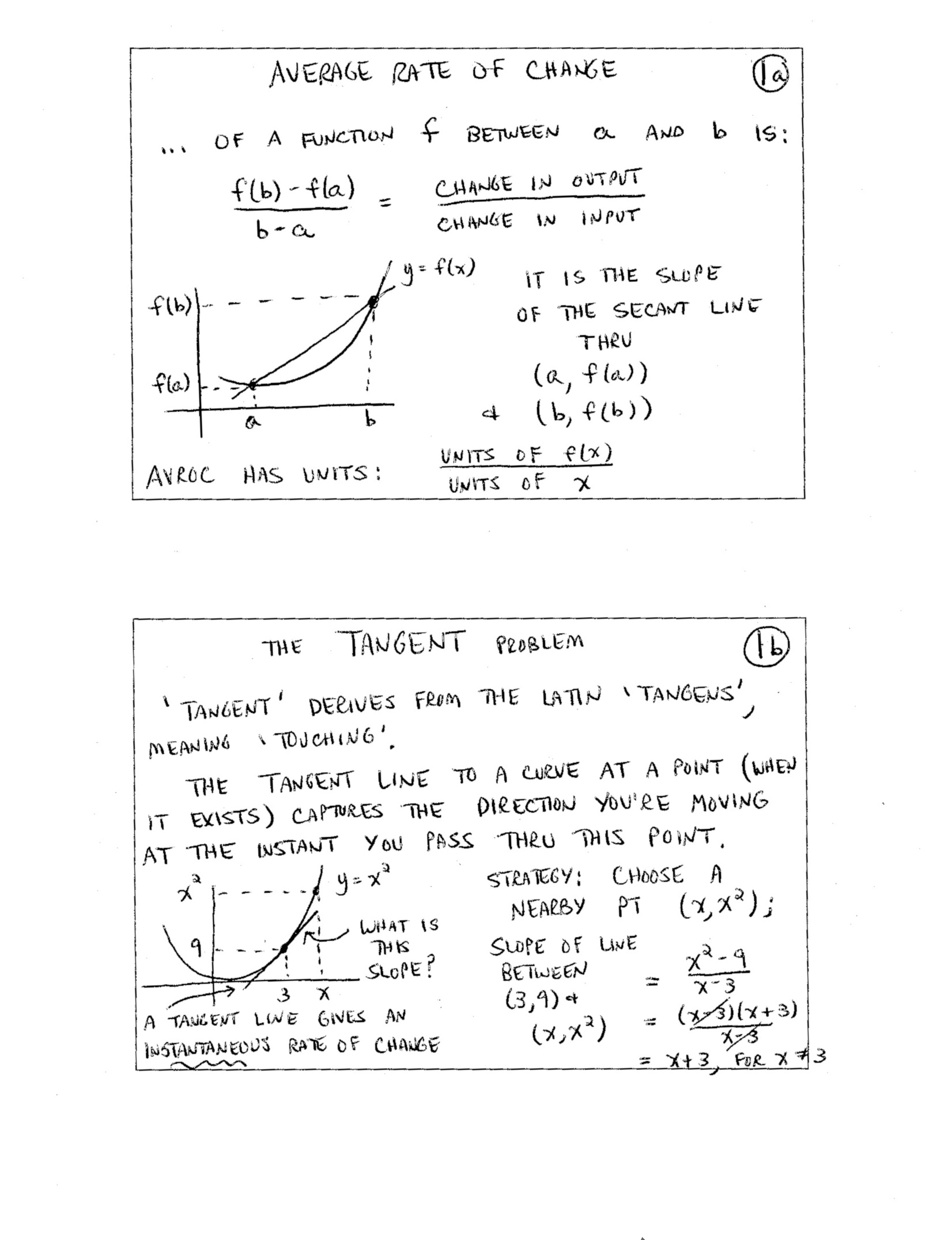2.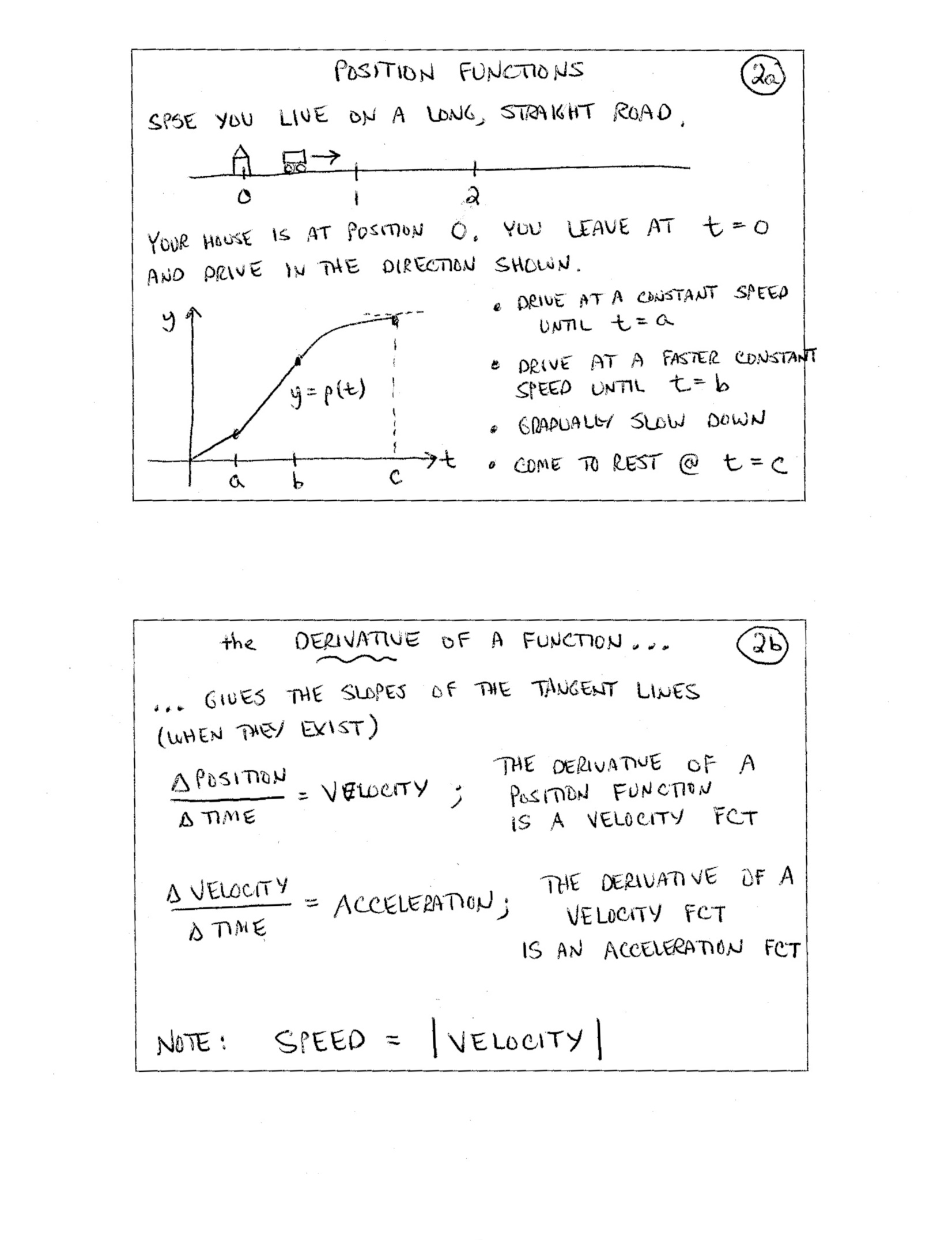3.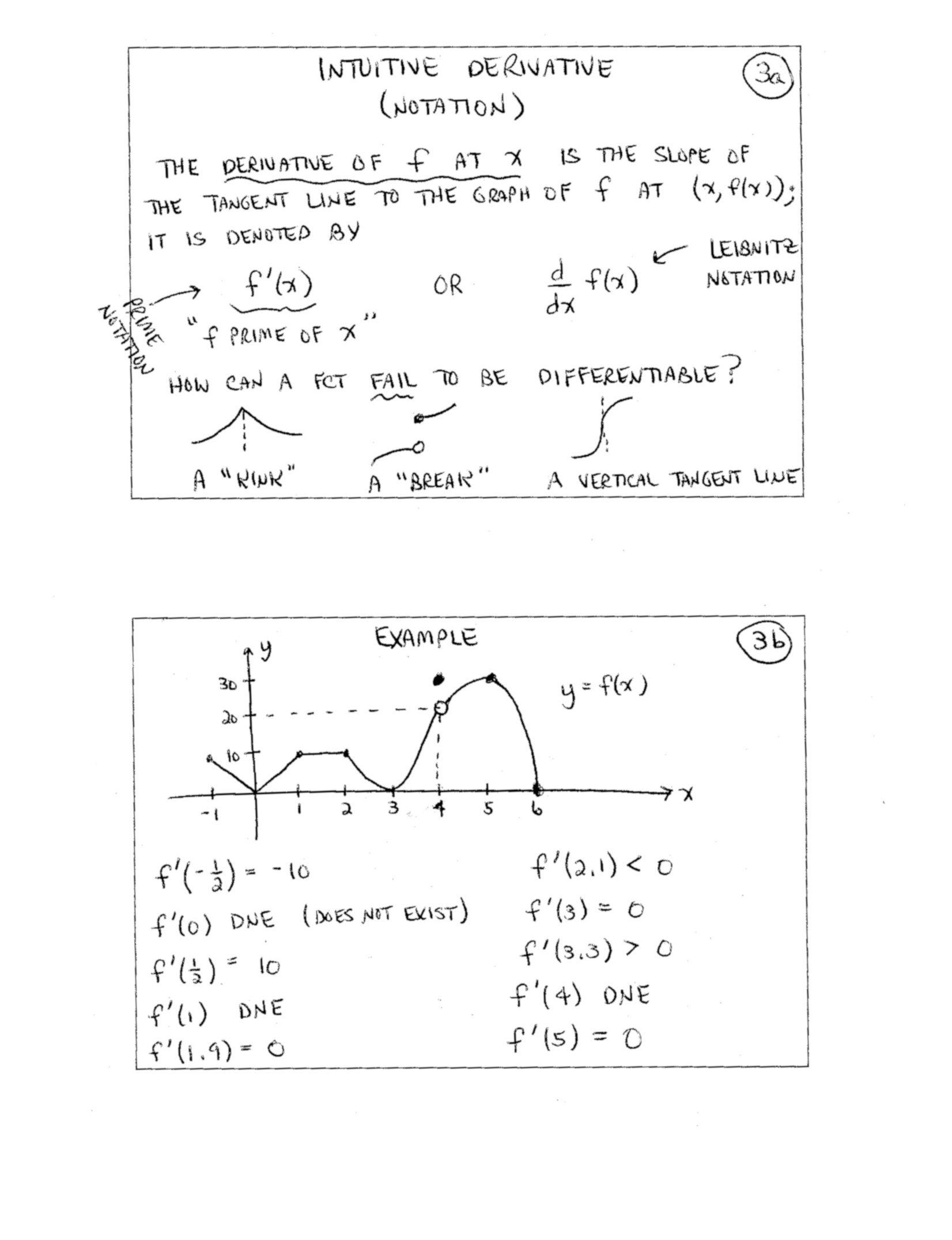4.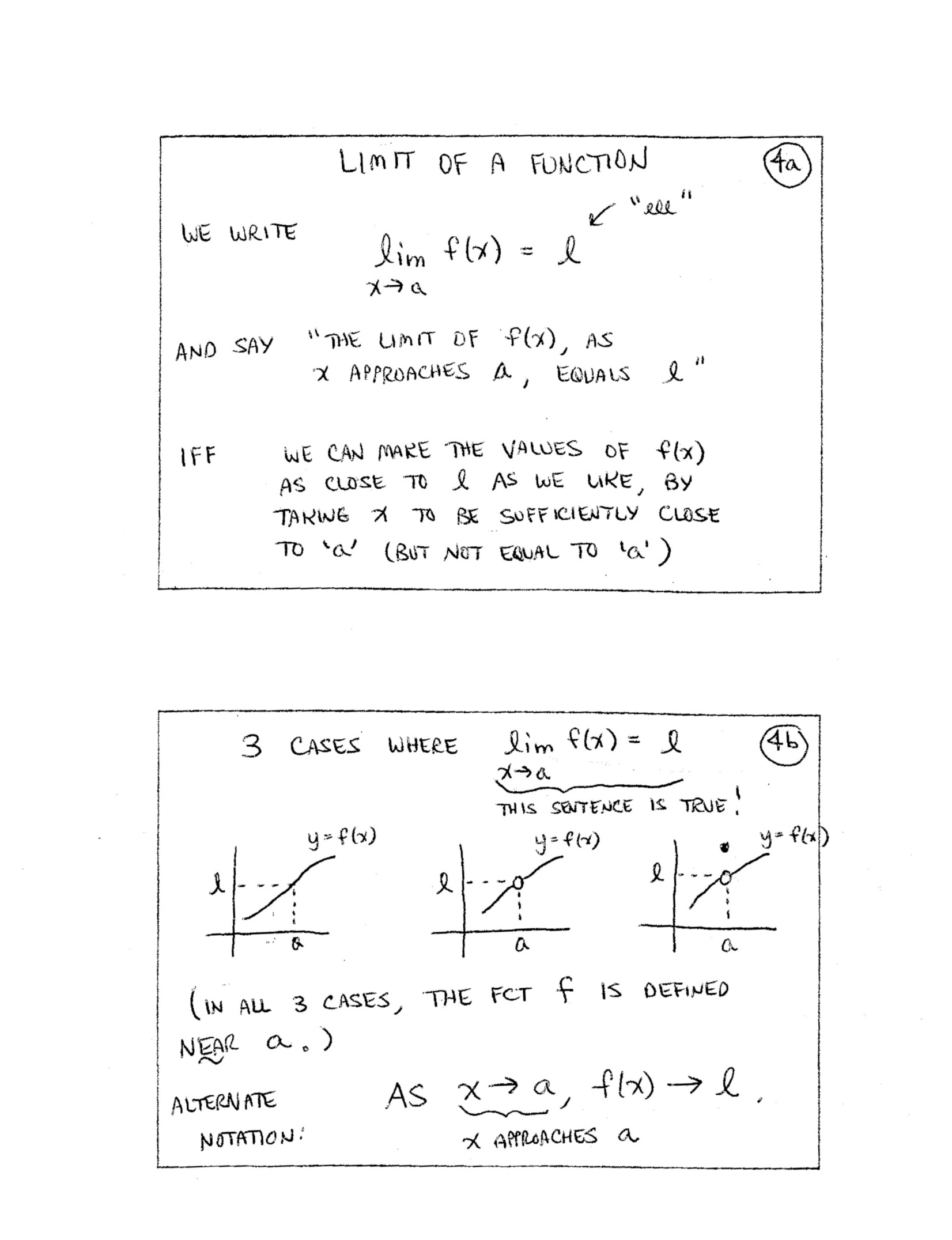5.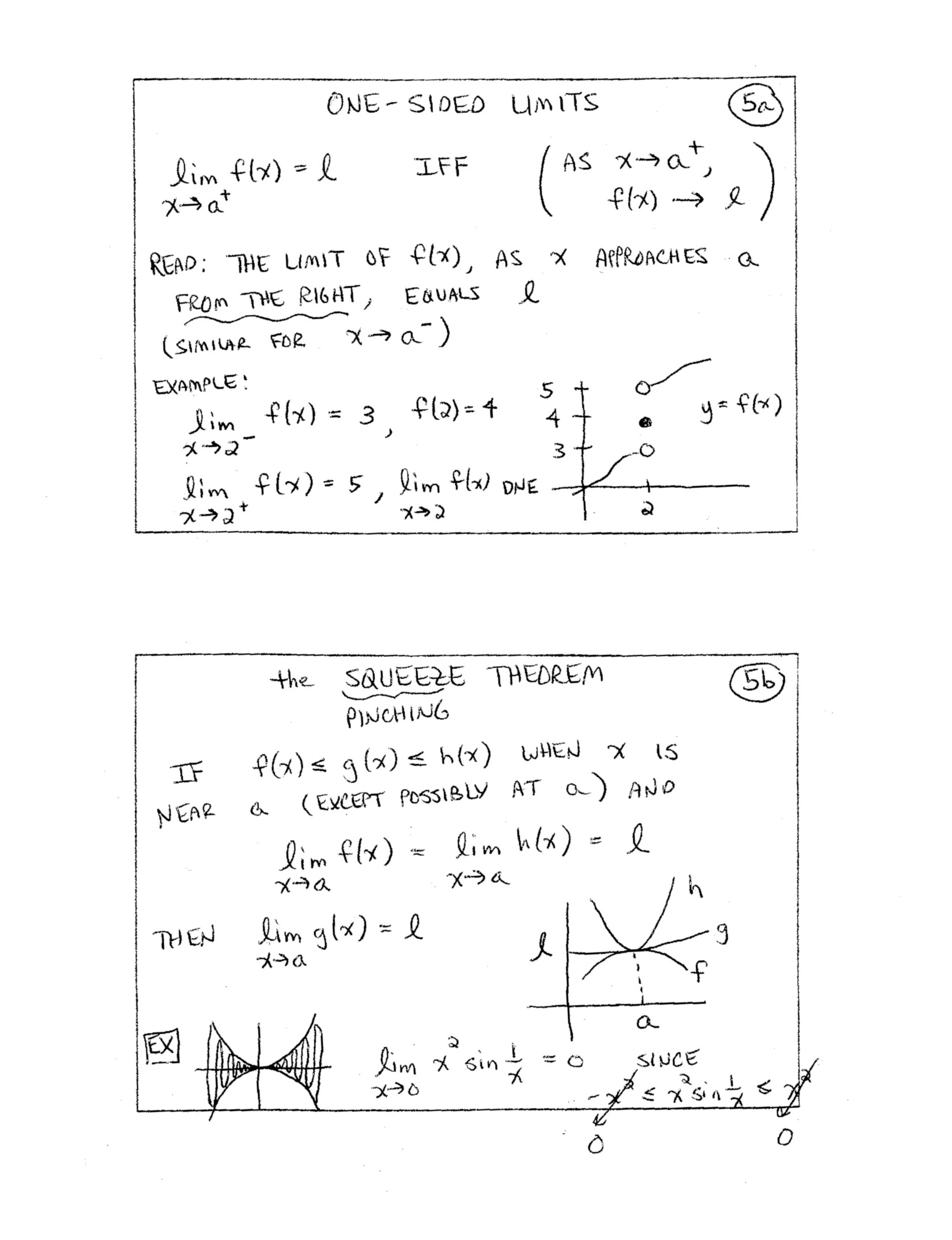6.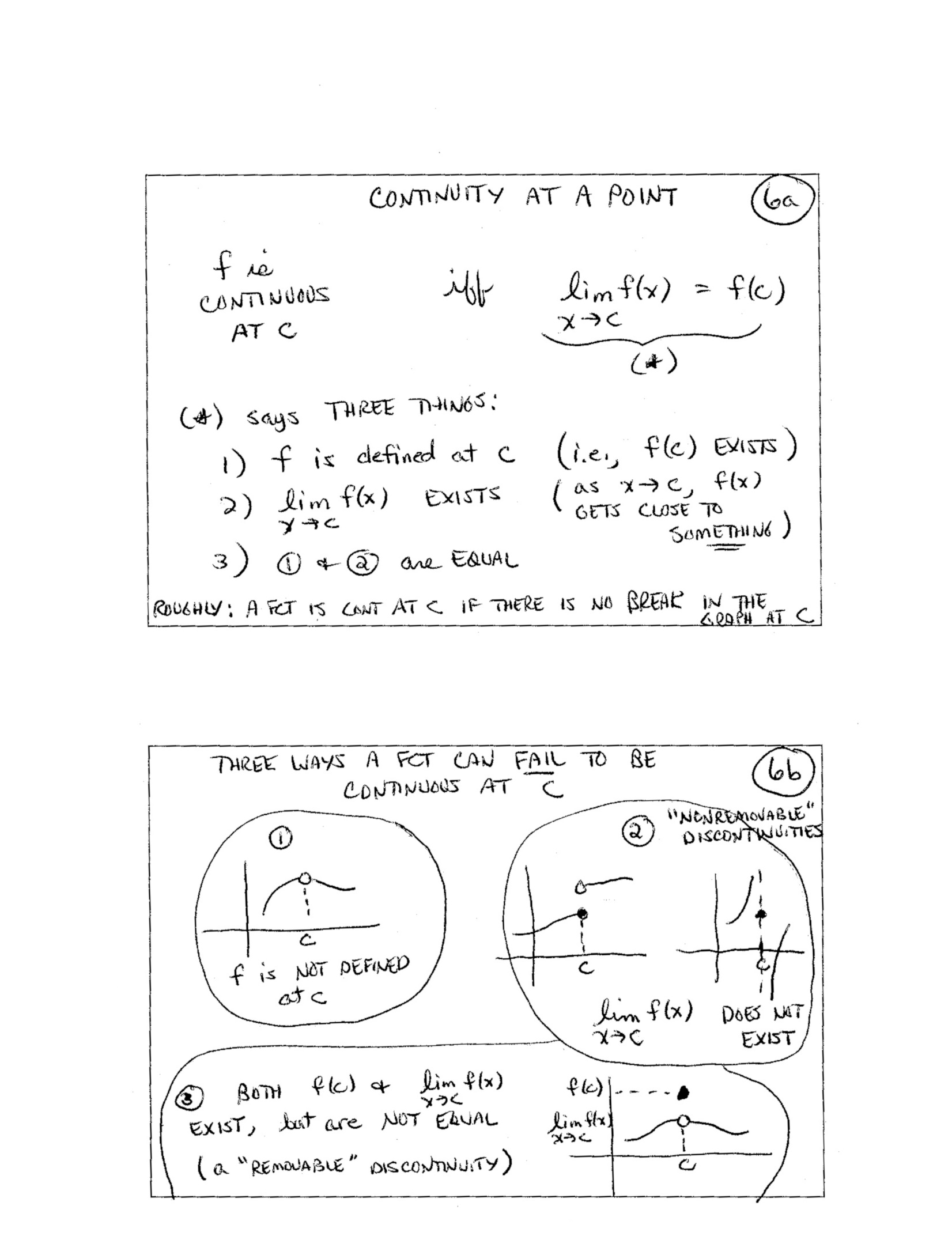7.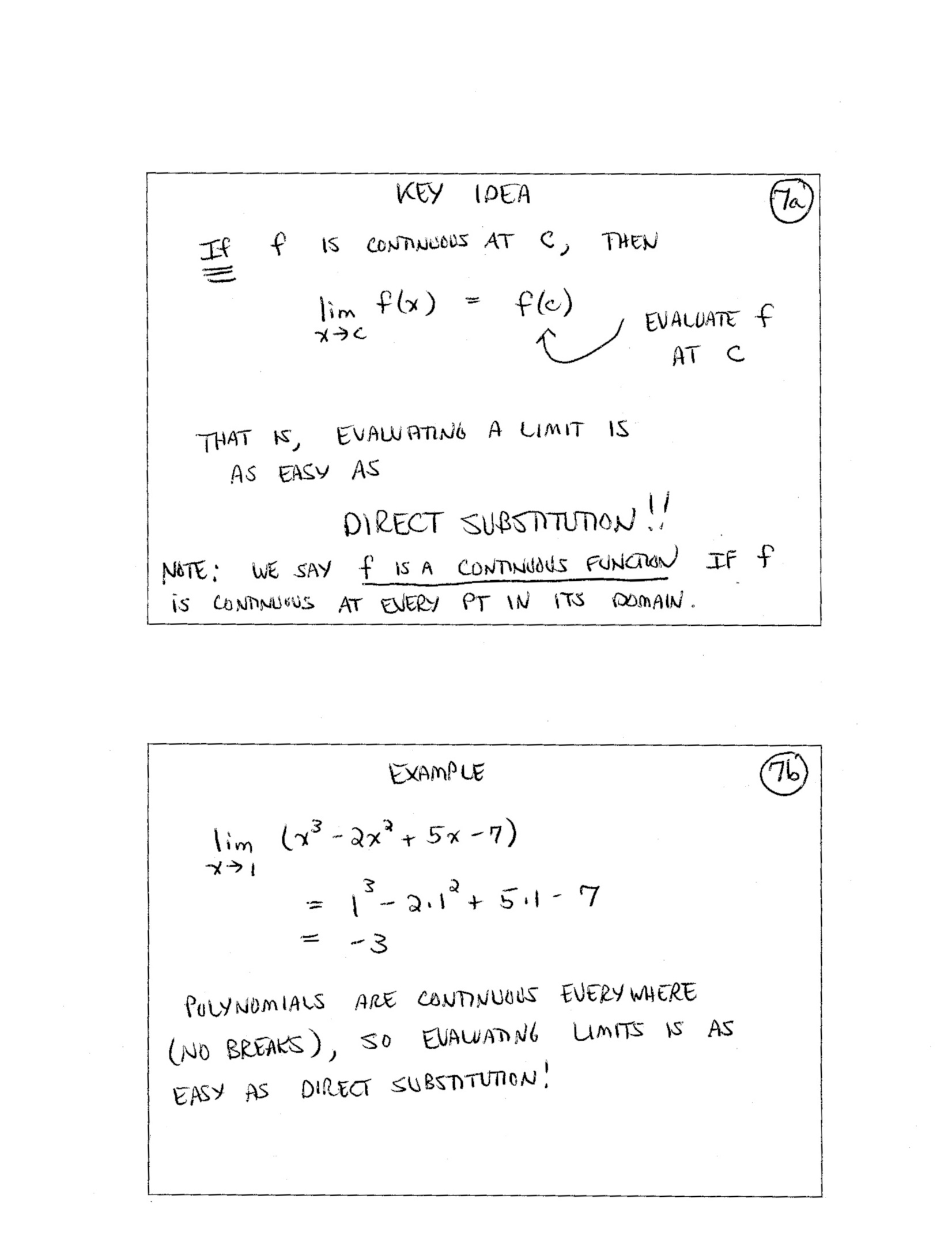8.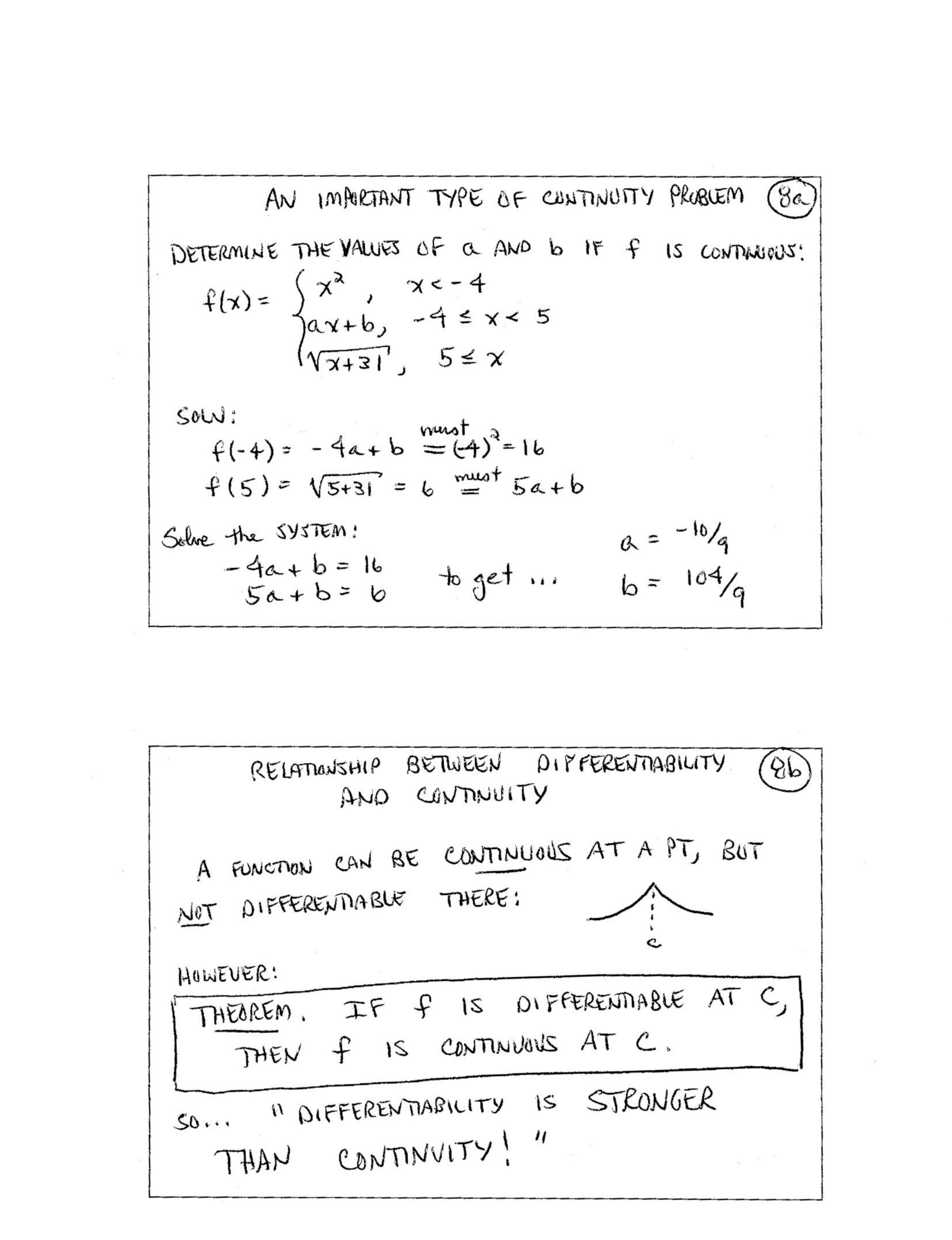9.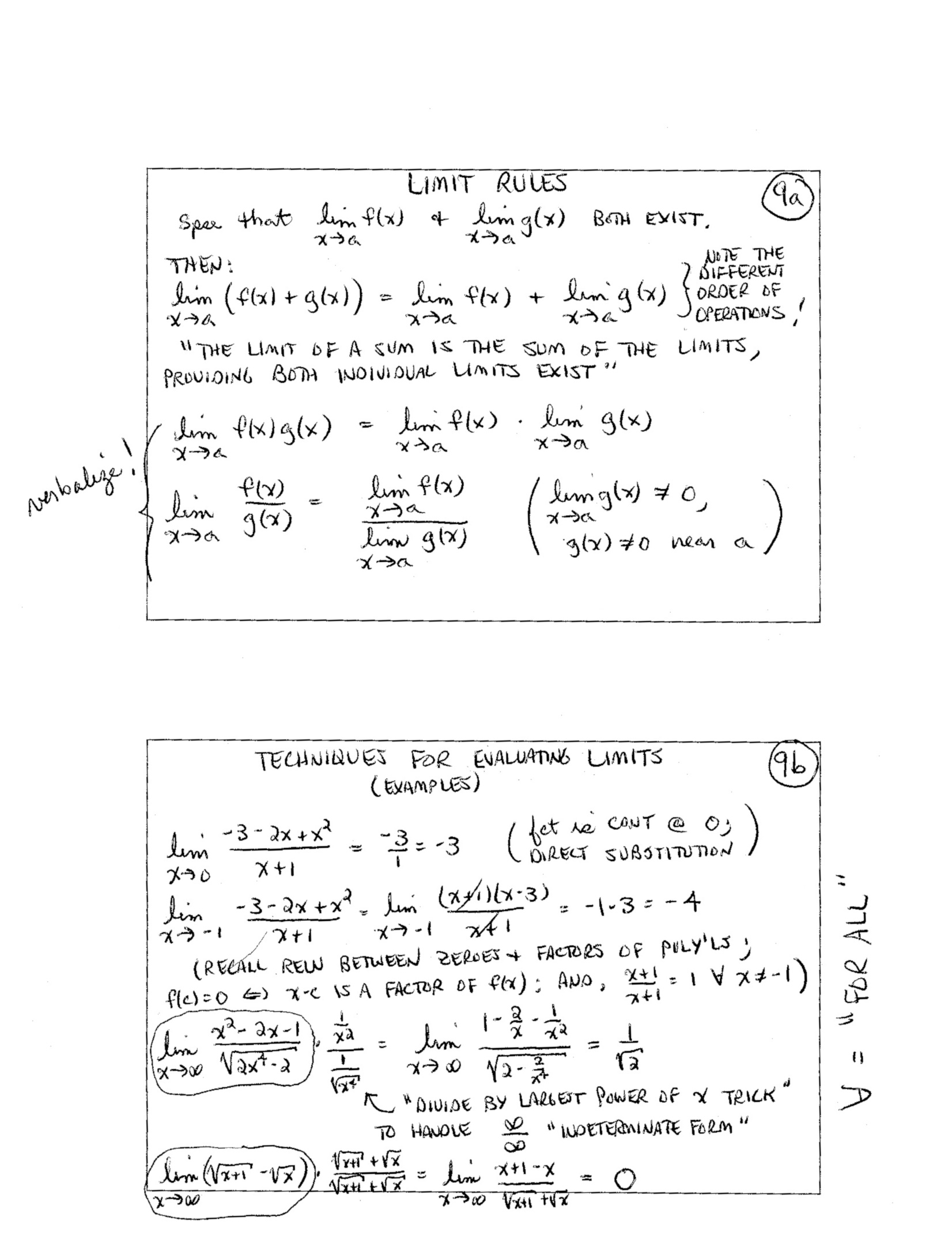10.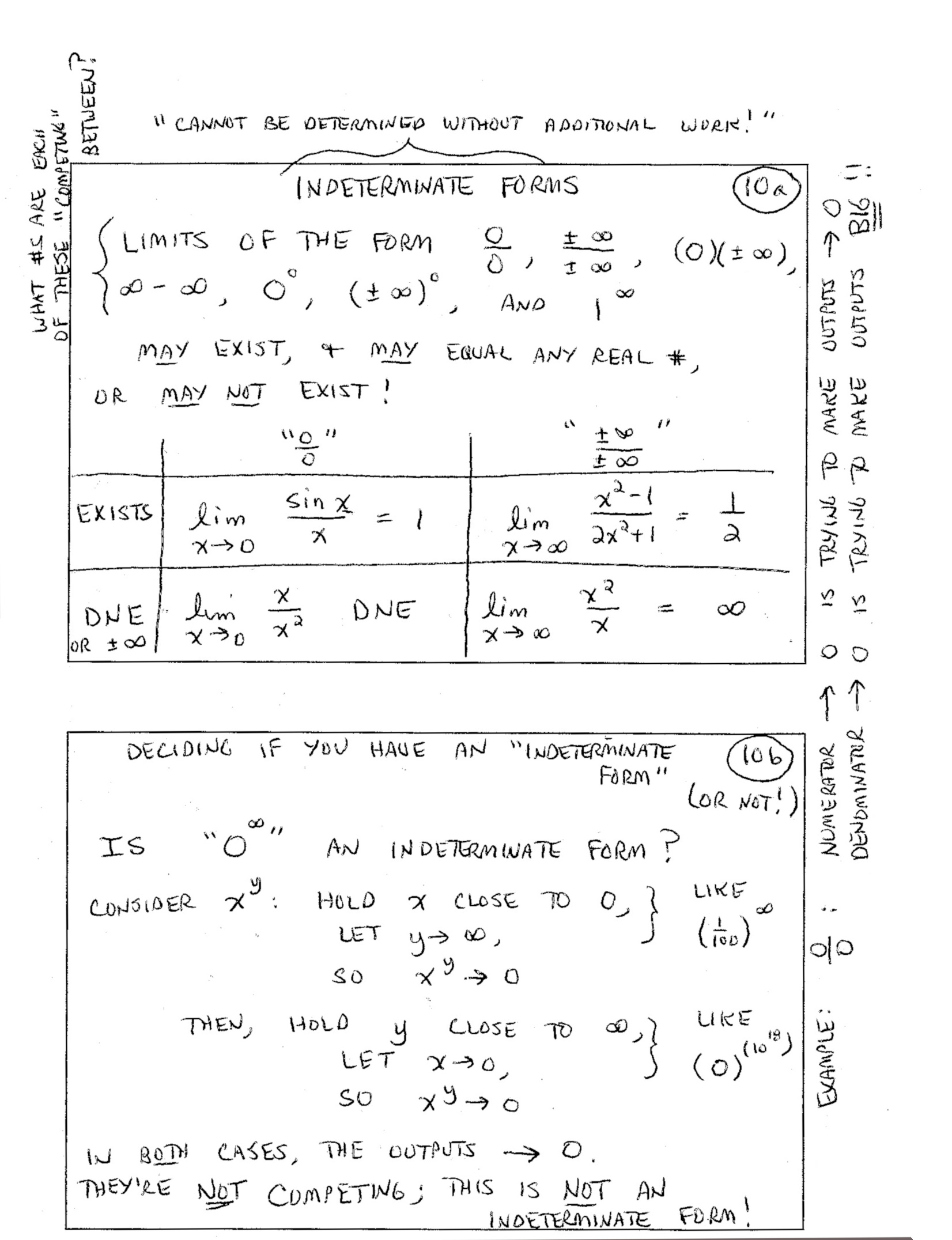11.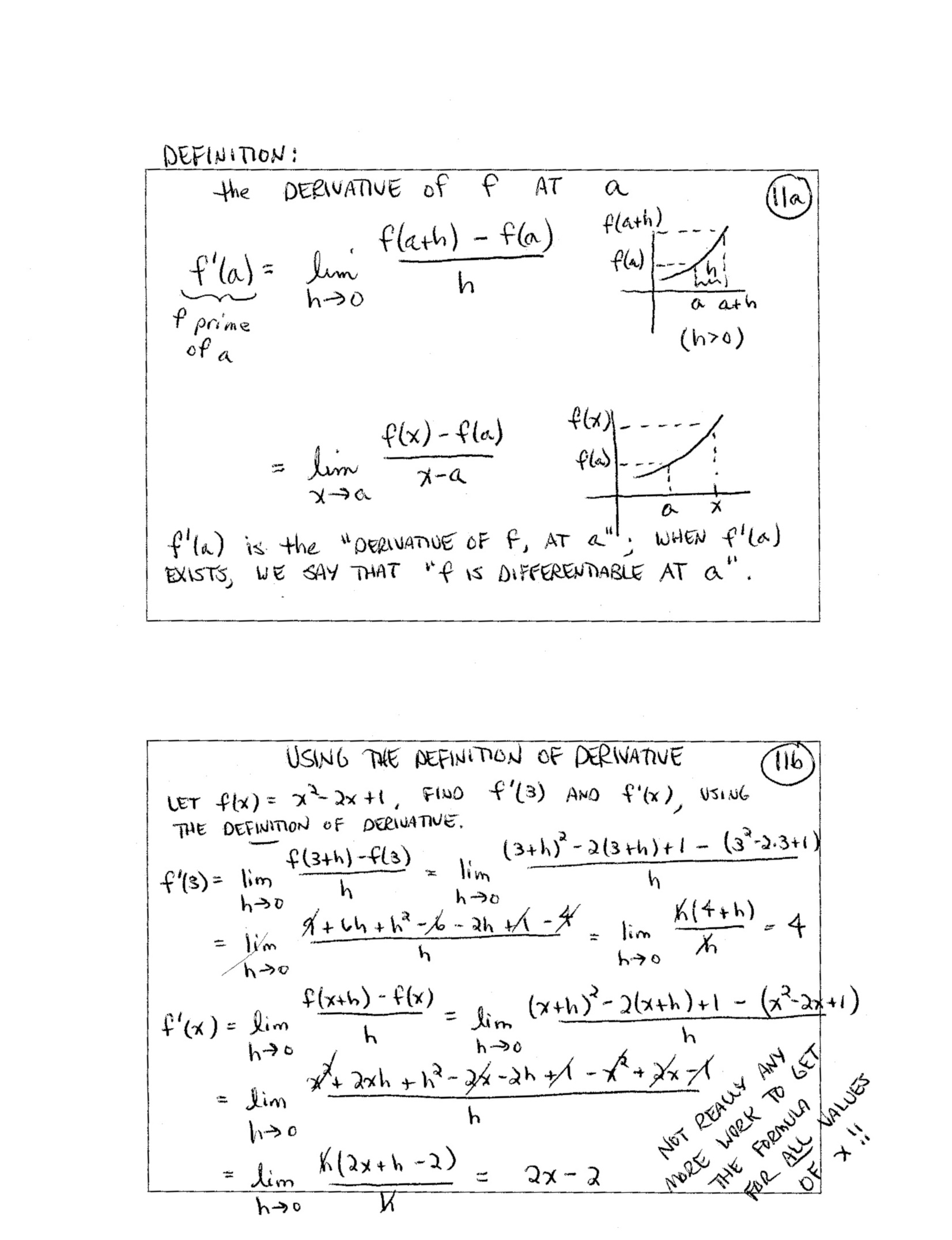12.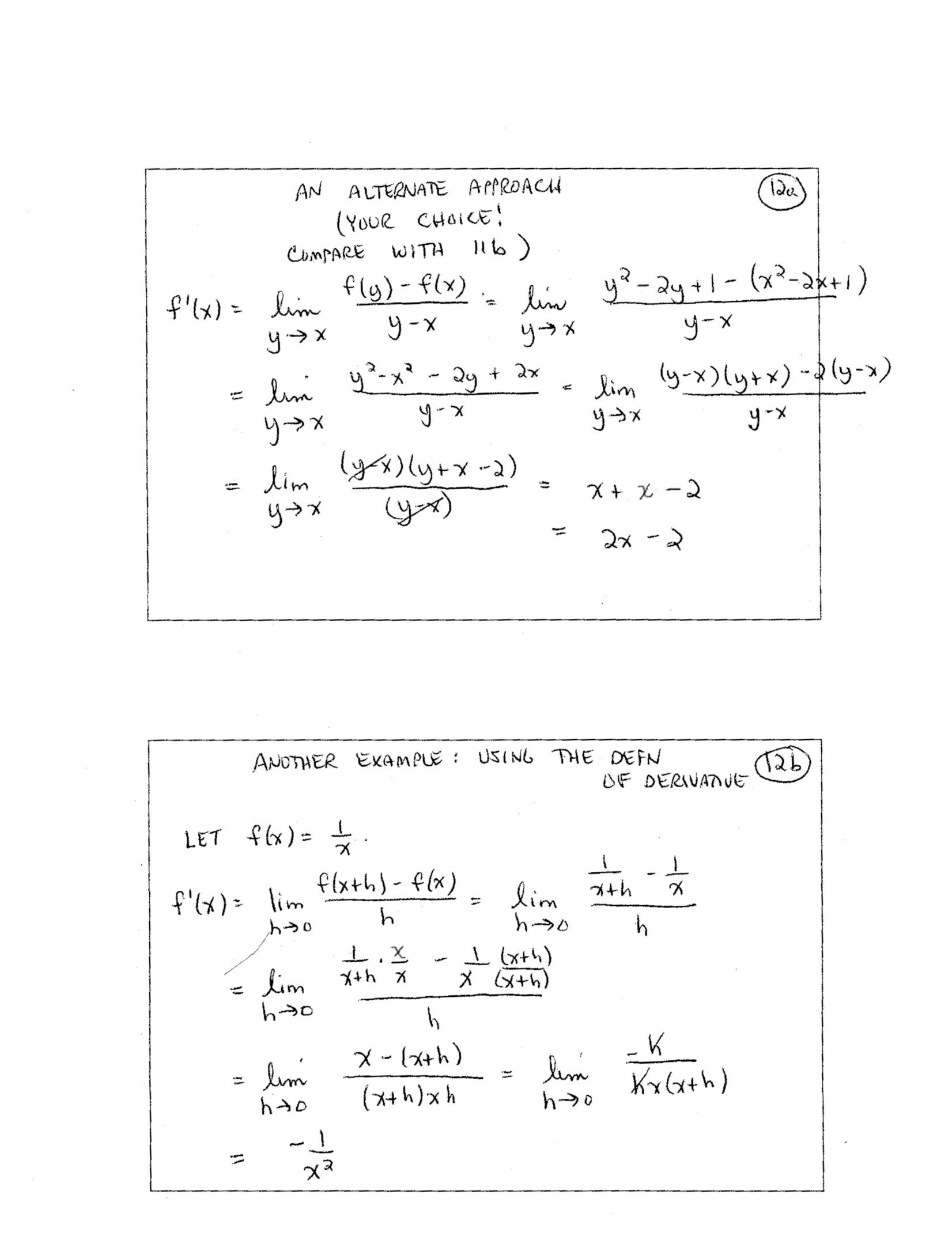13.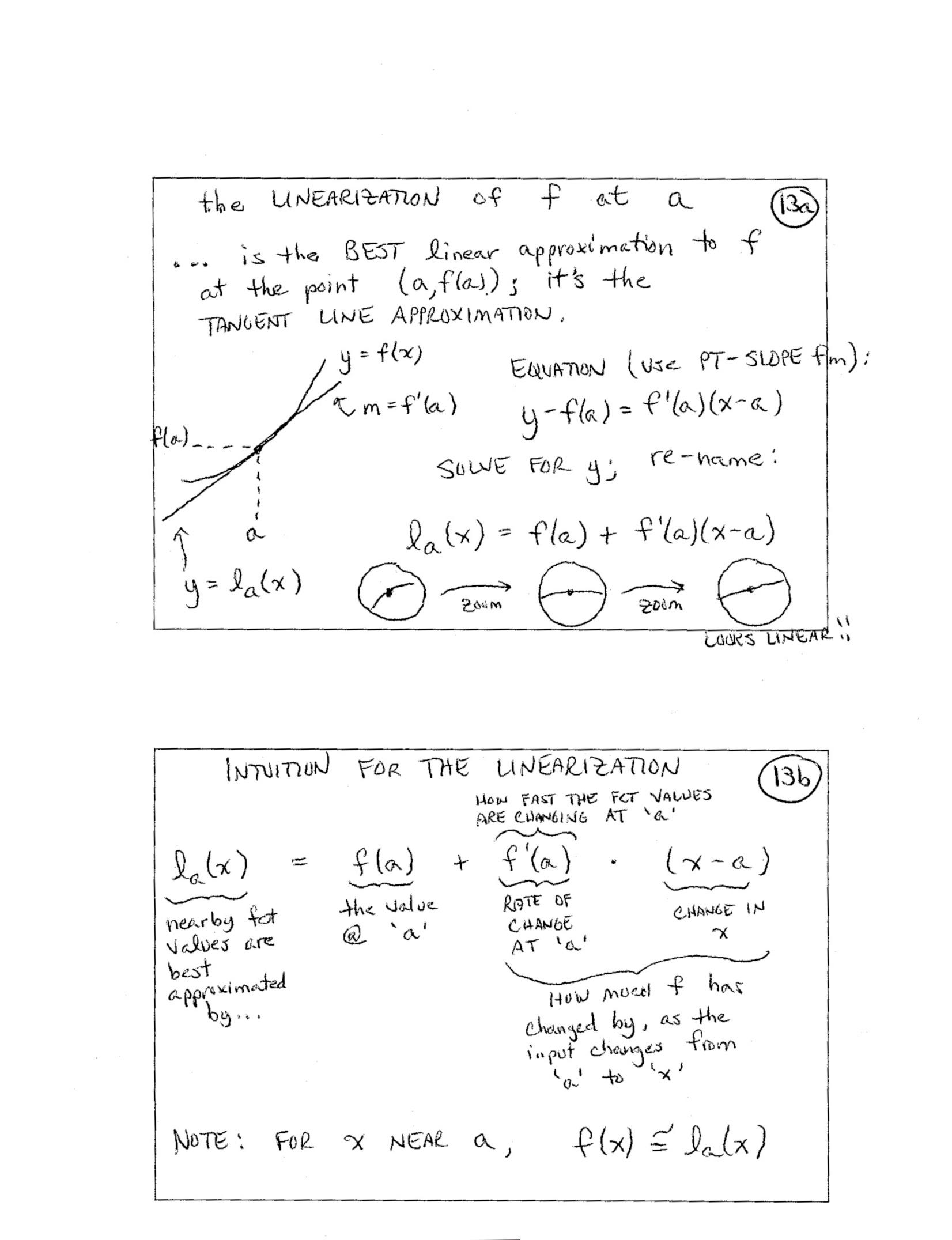14.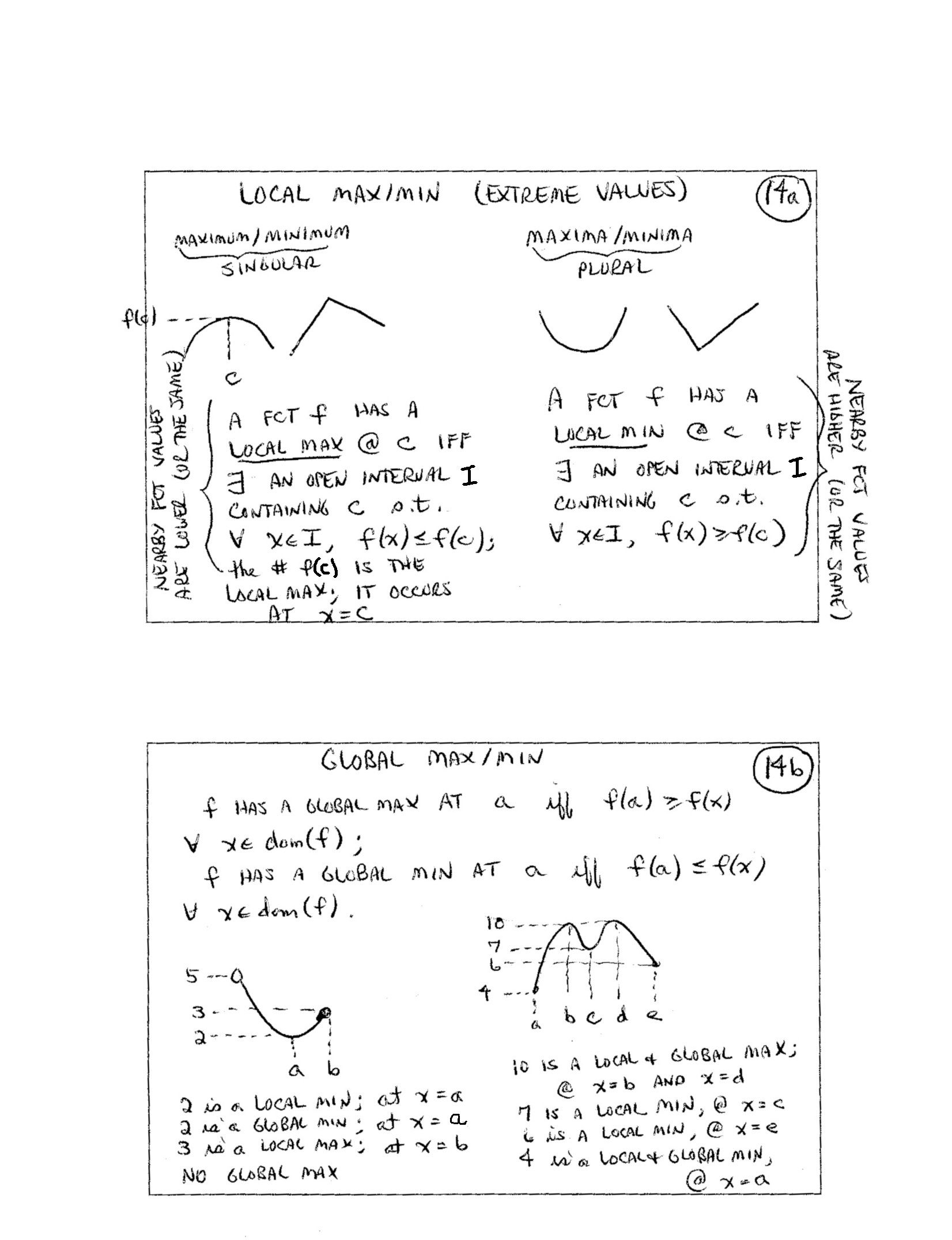15.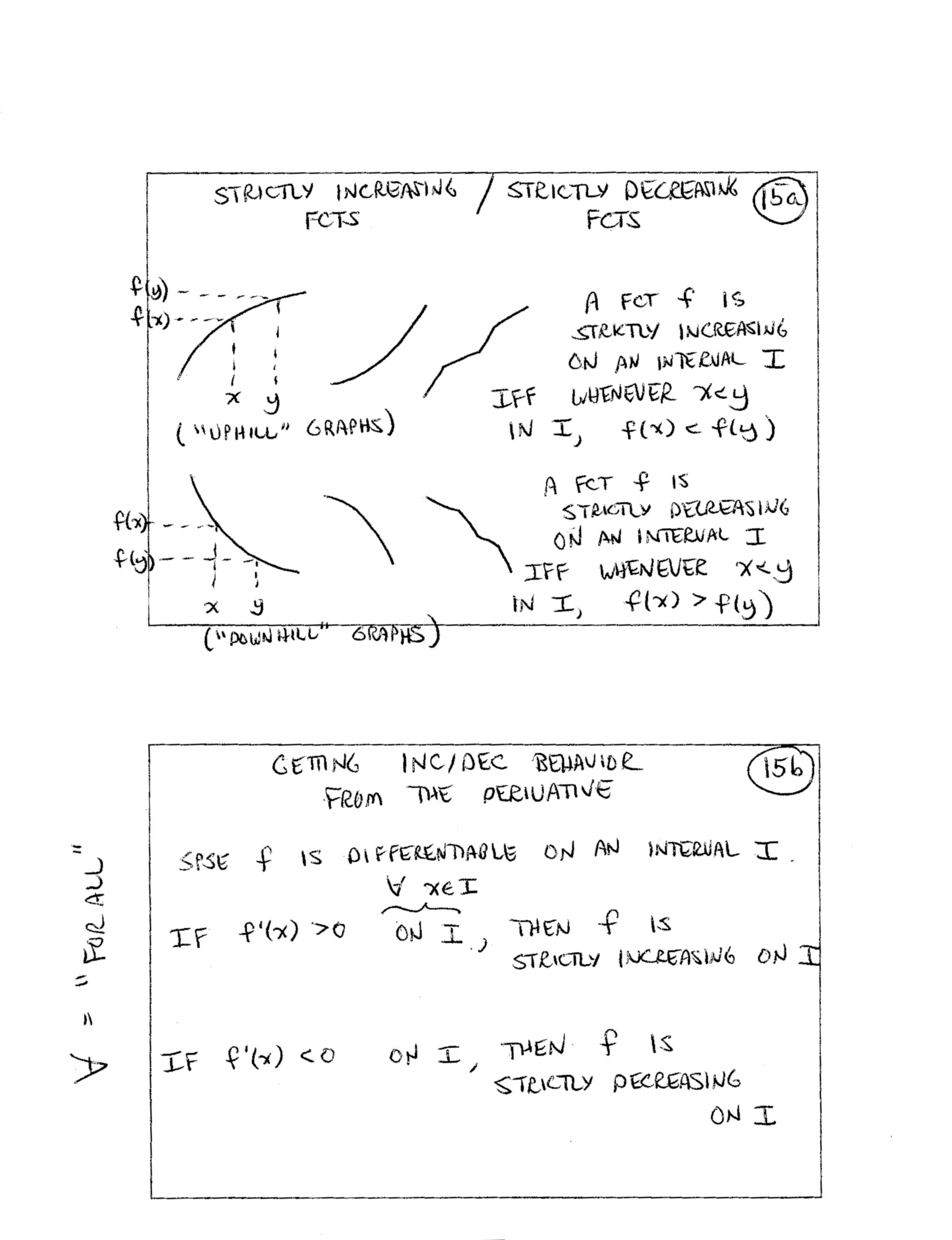16.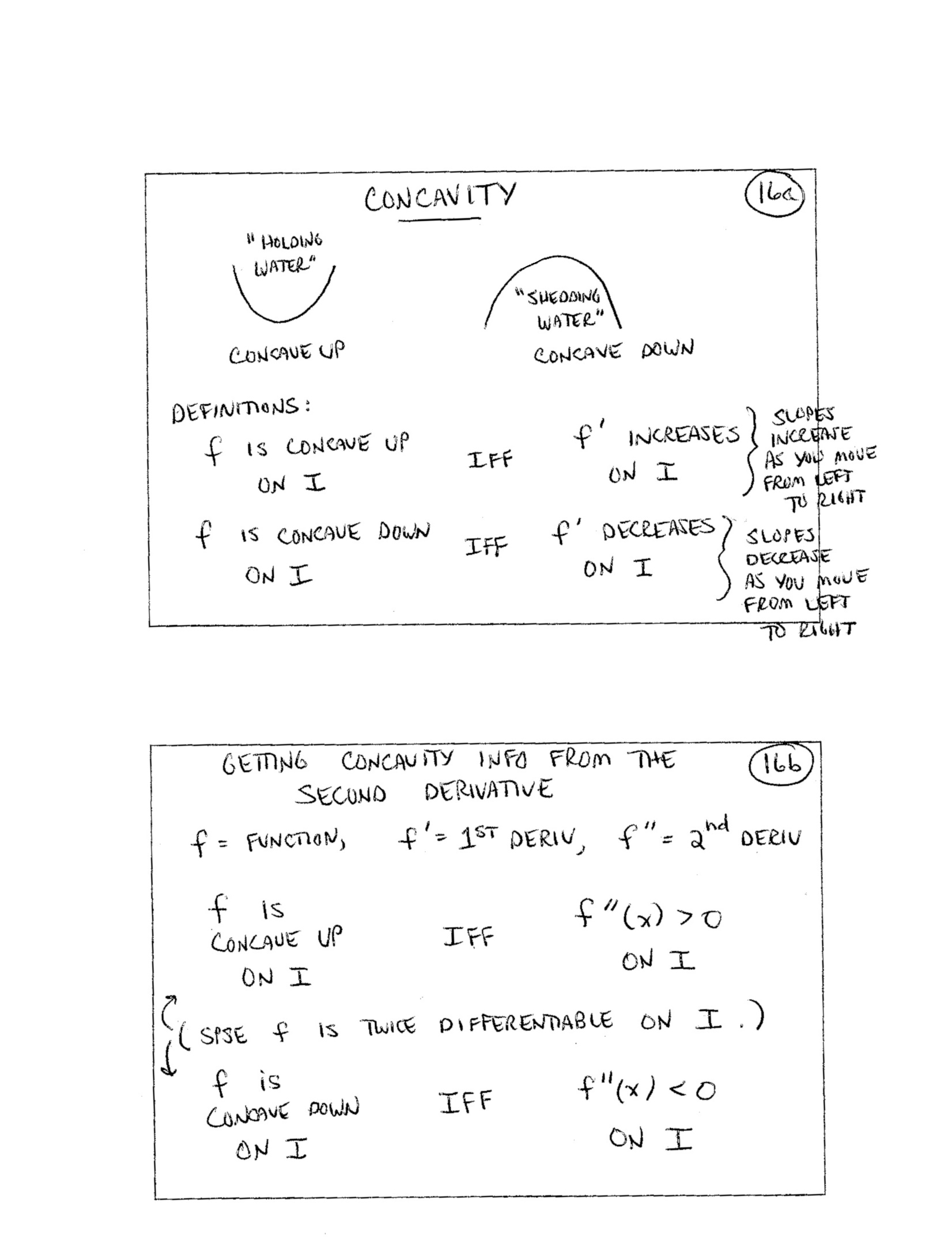17.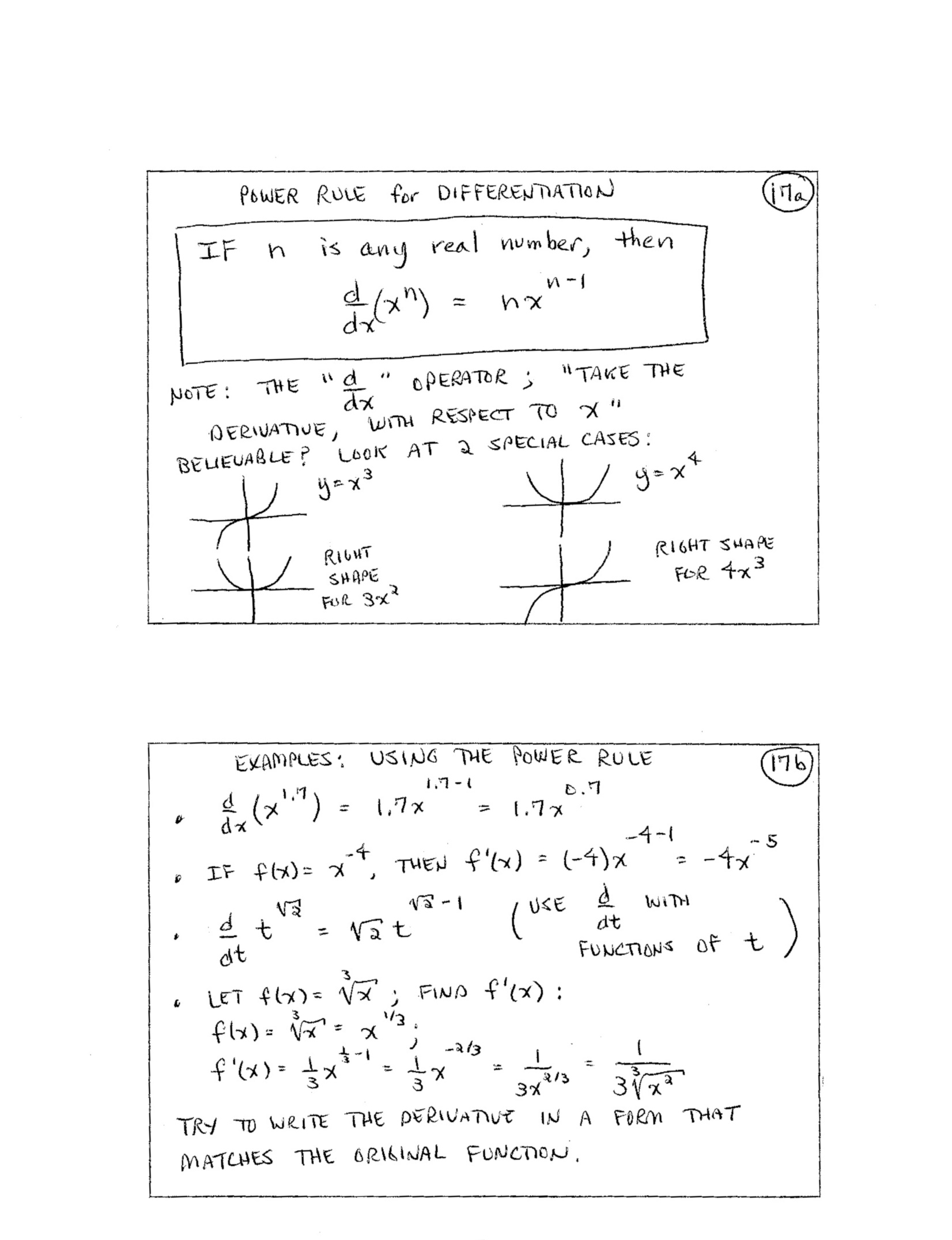18.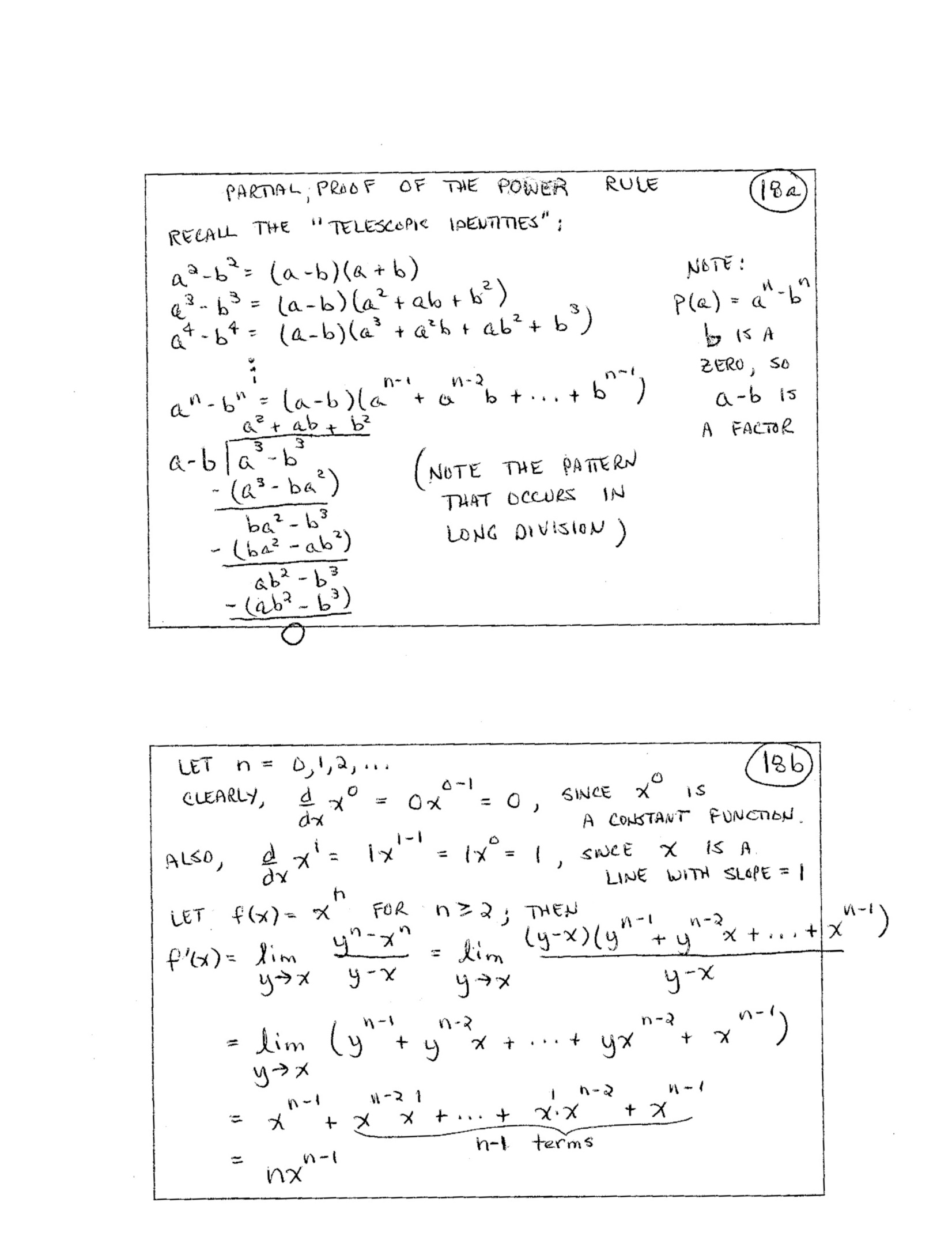19.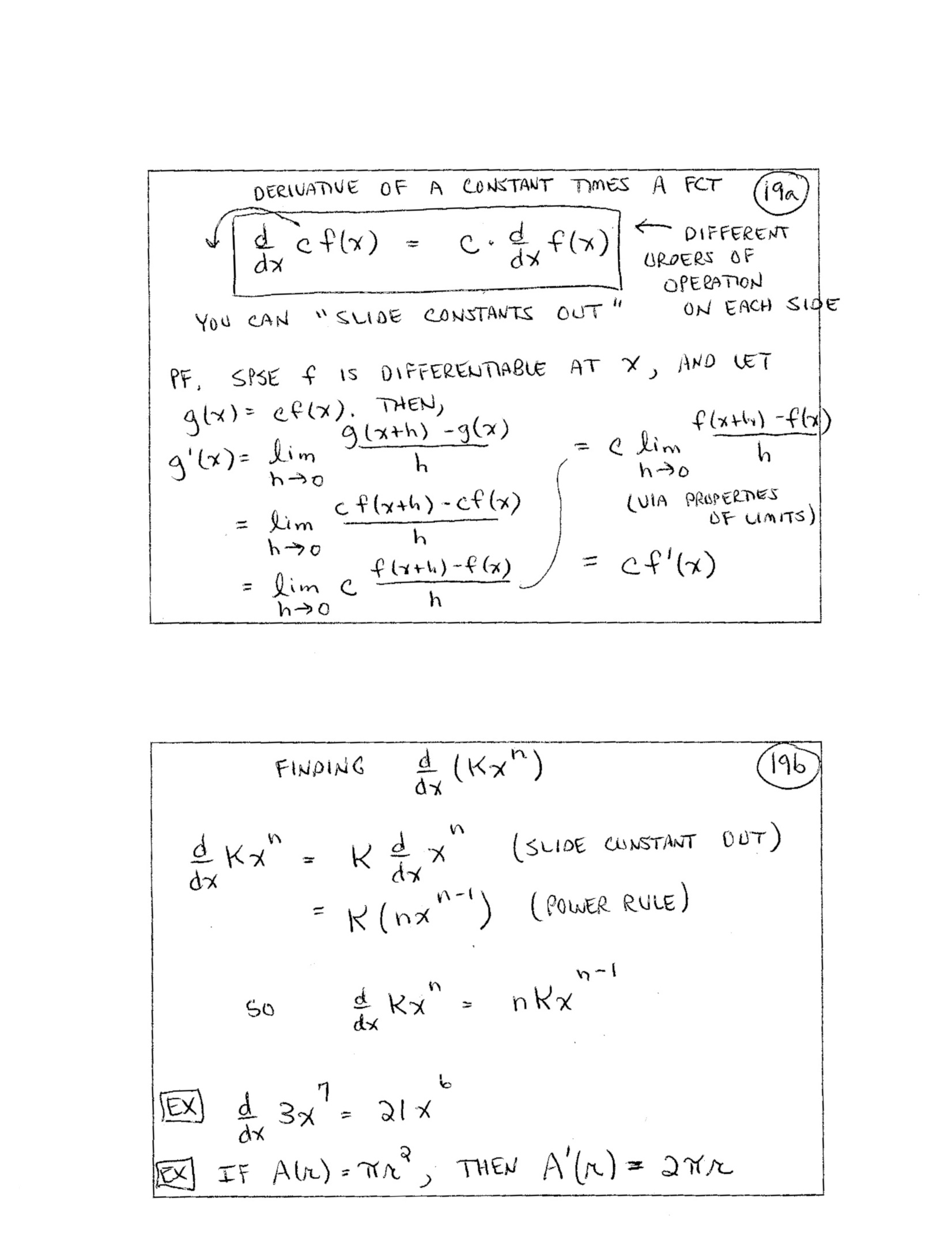20.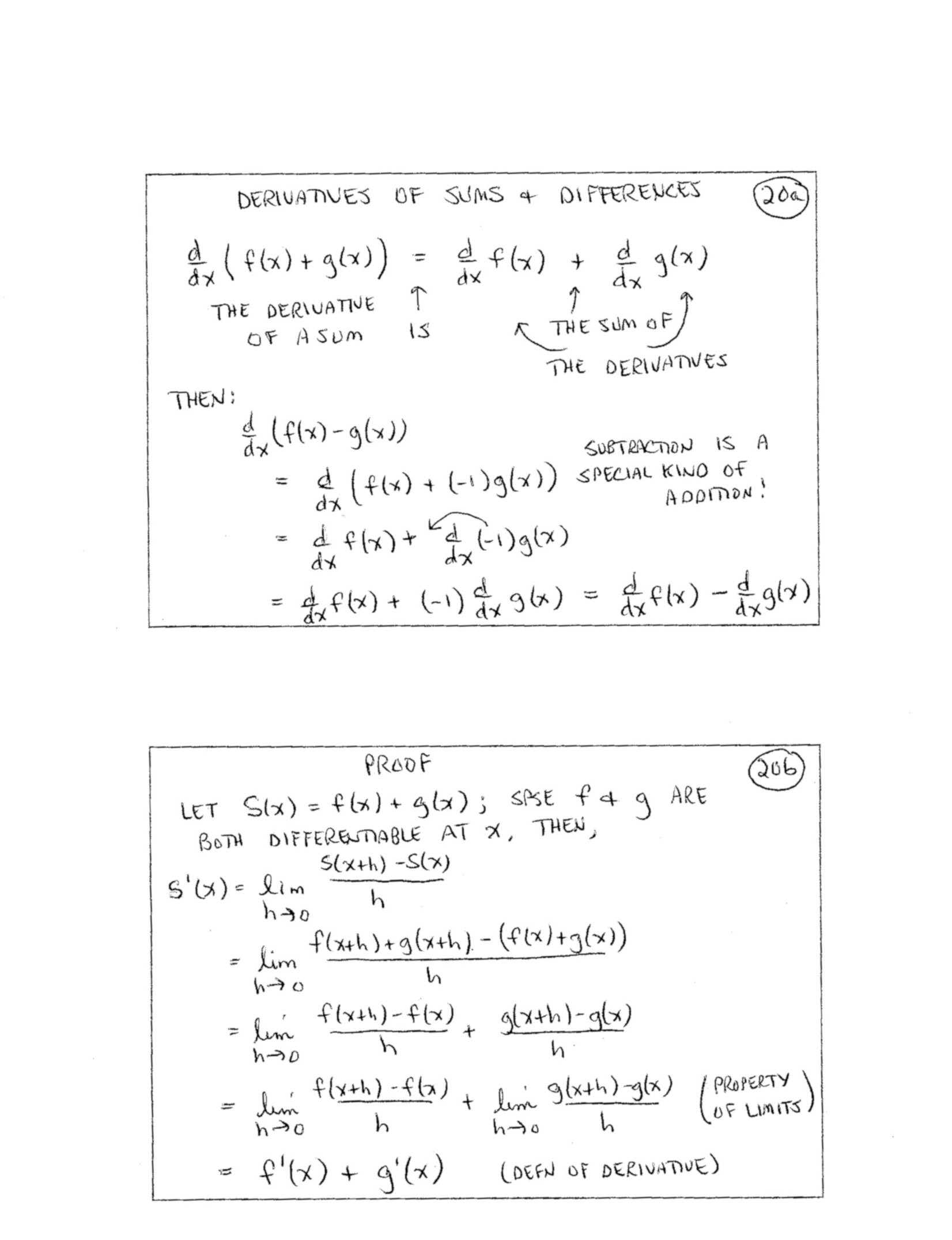21.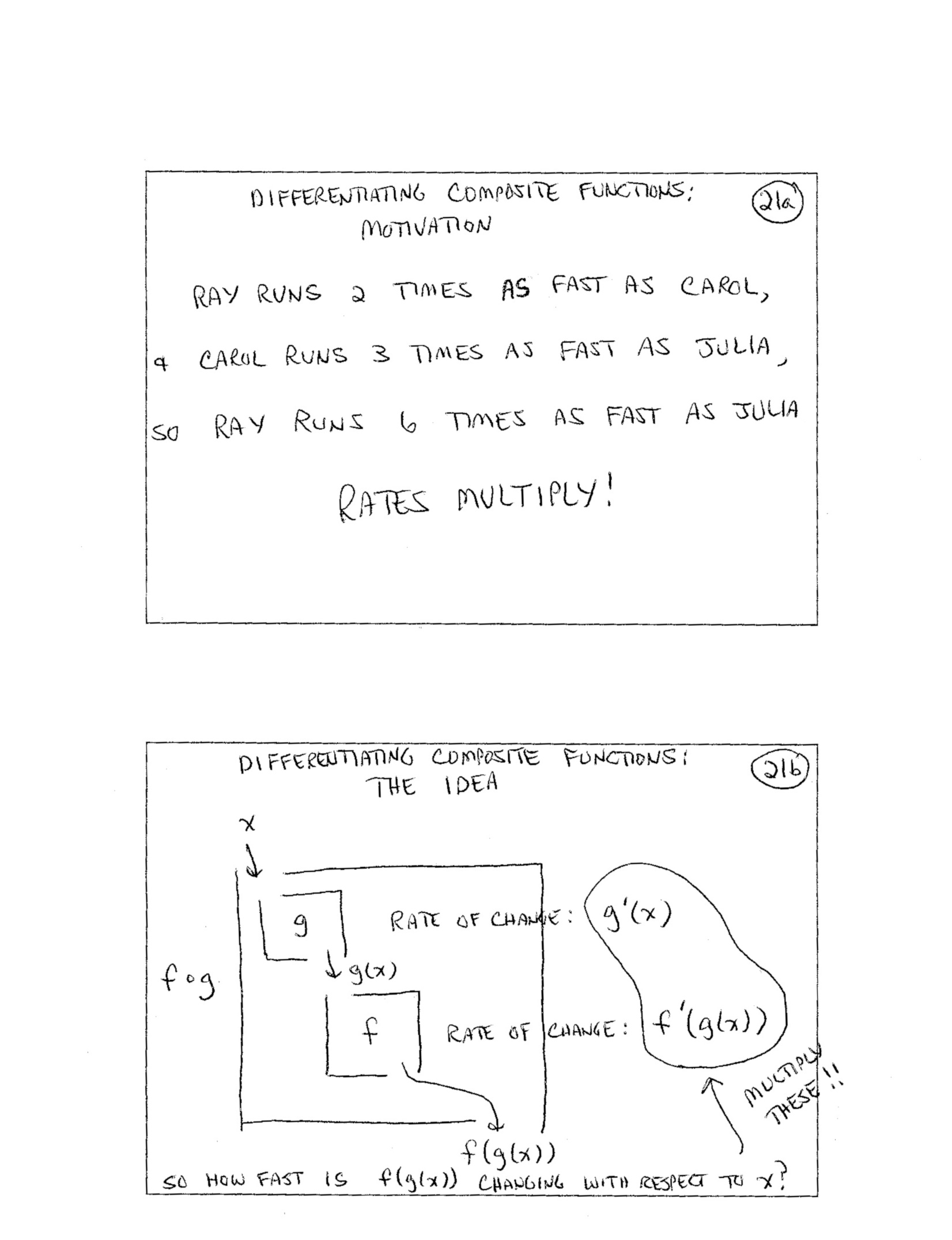22.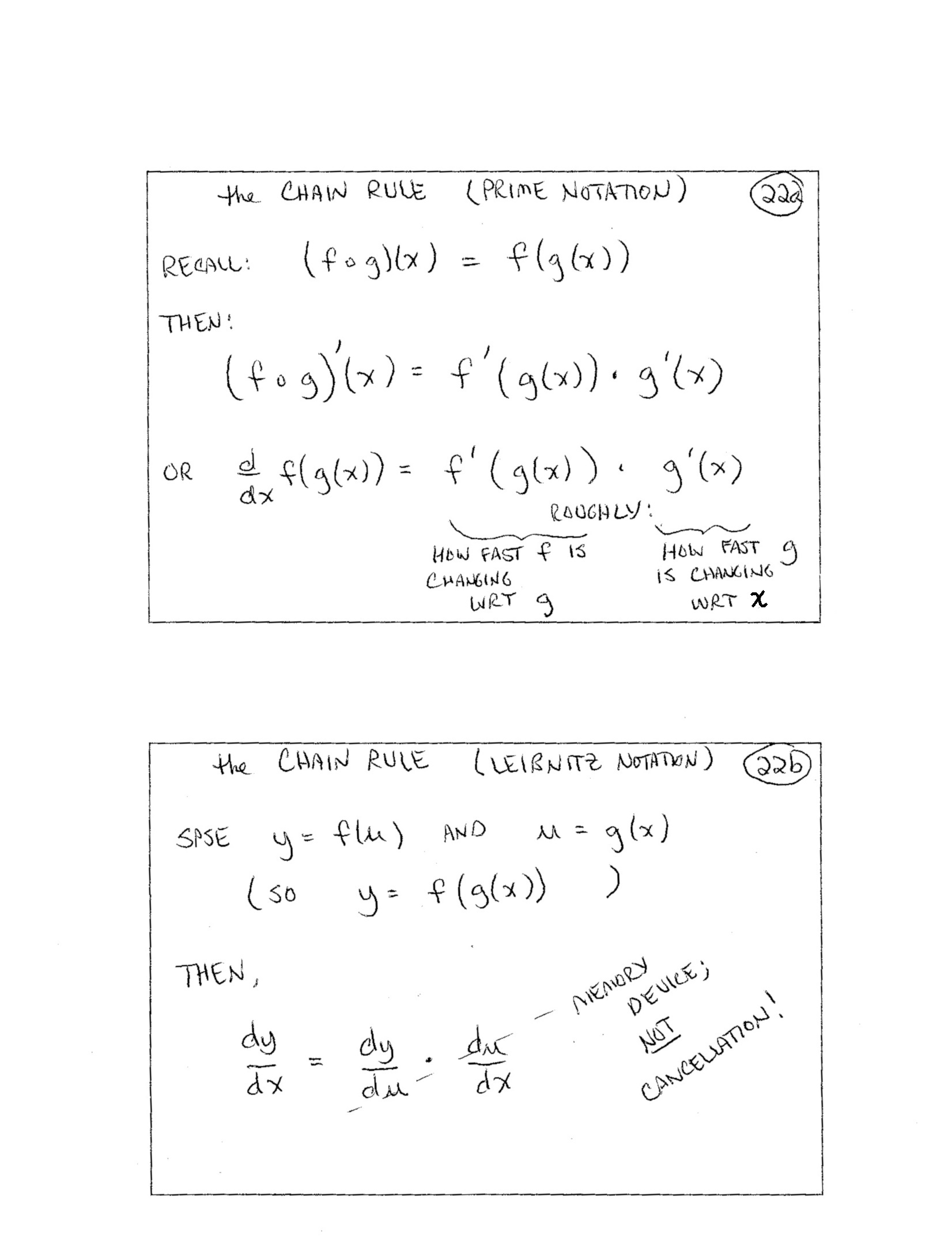23.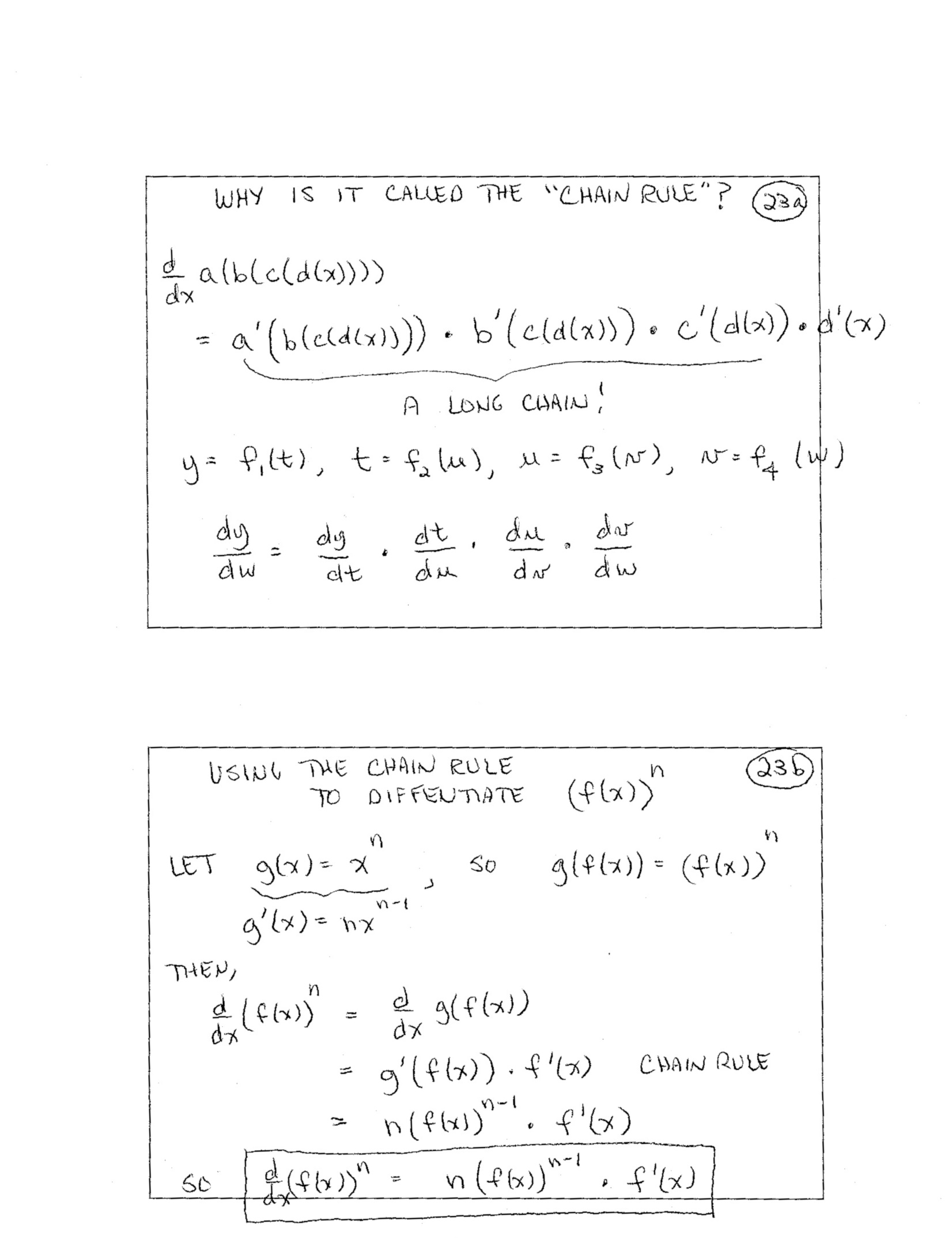24.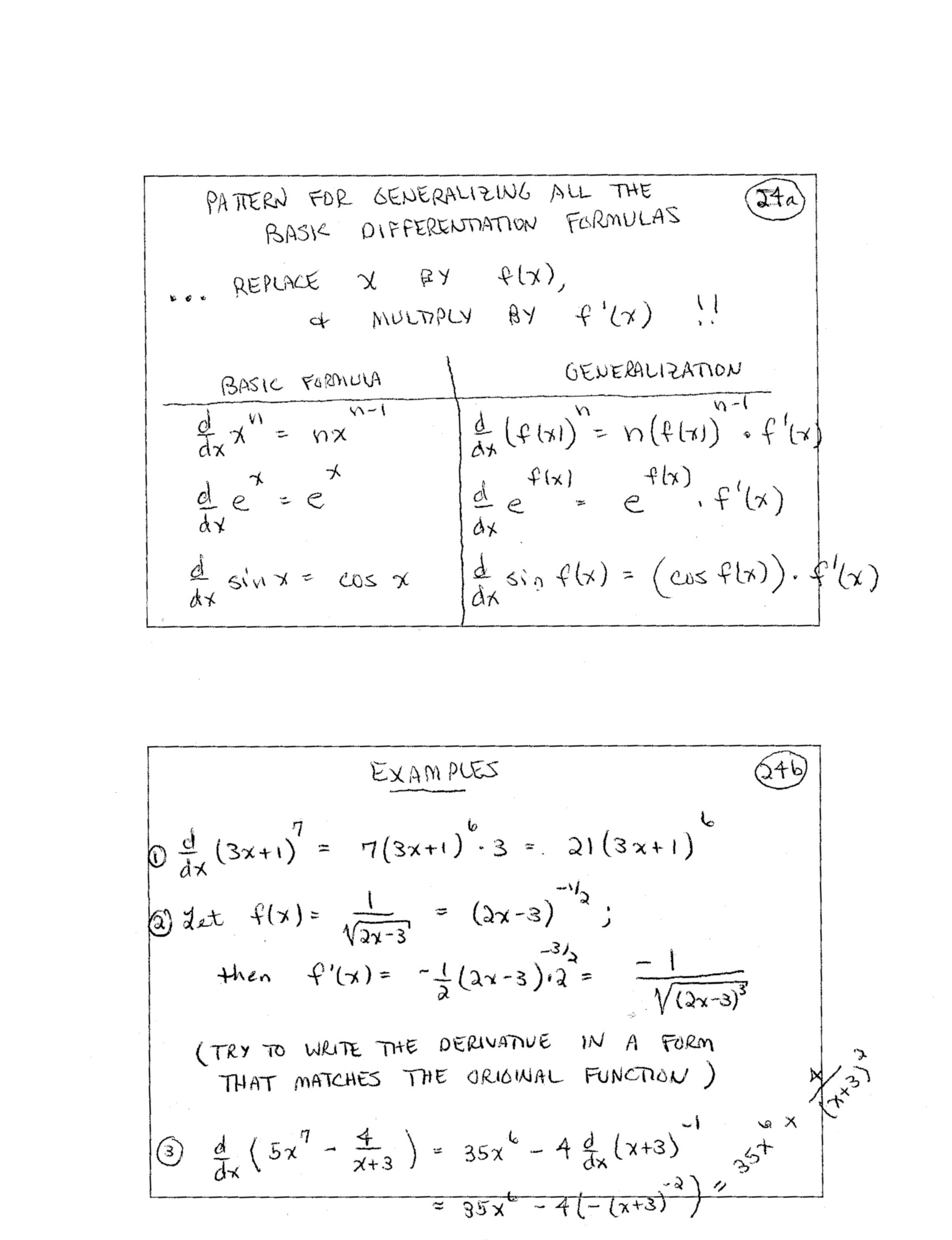25.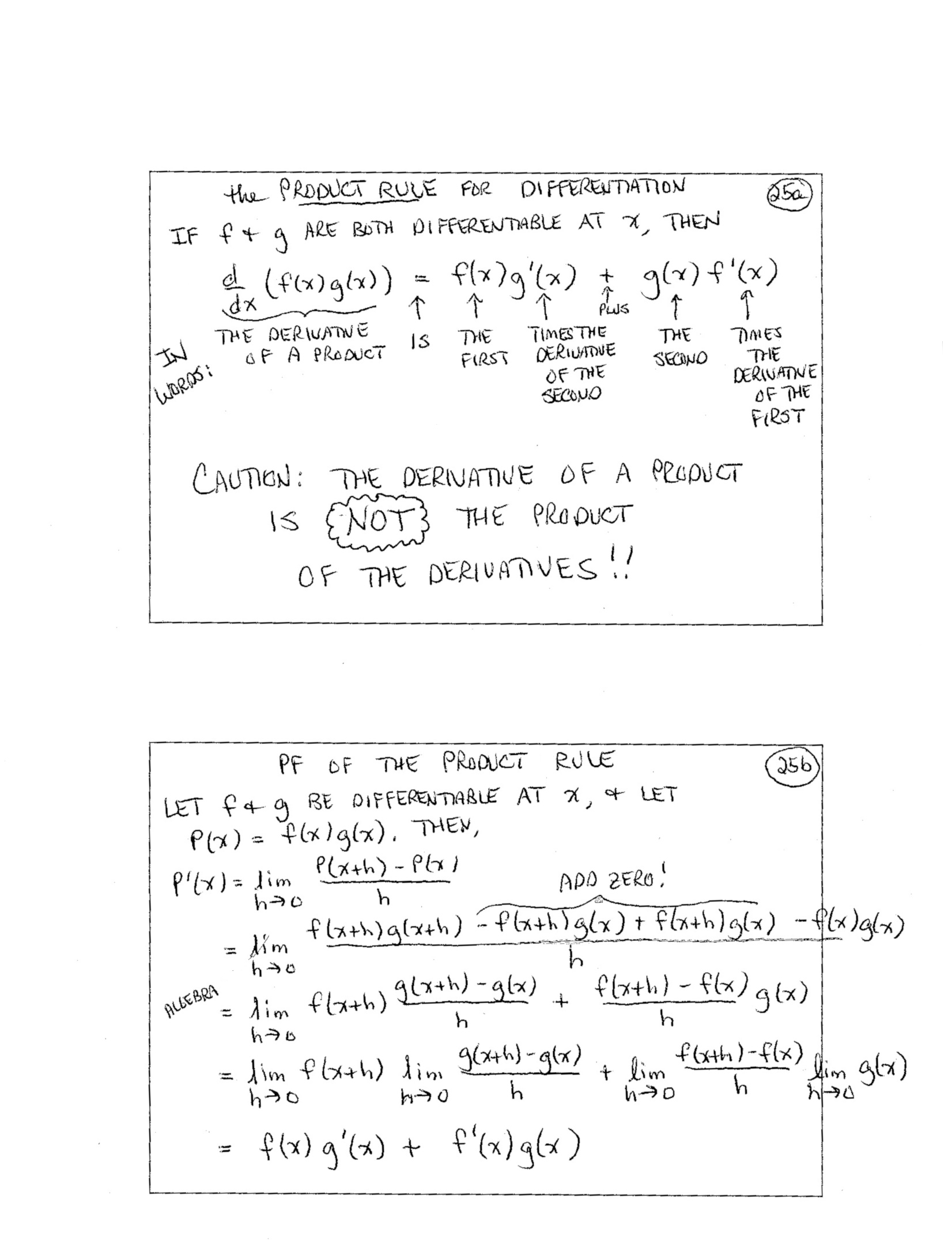26.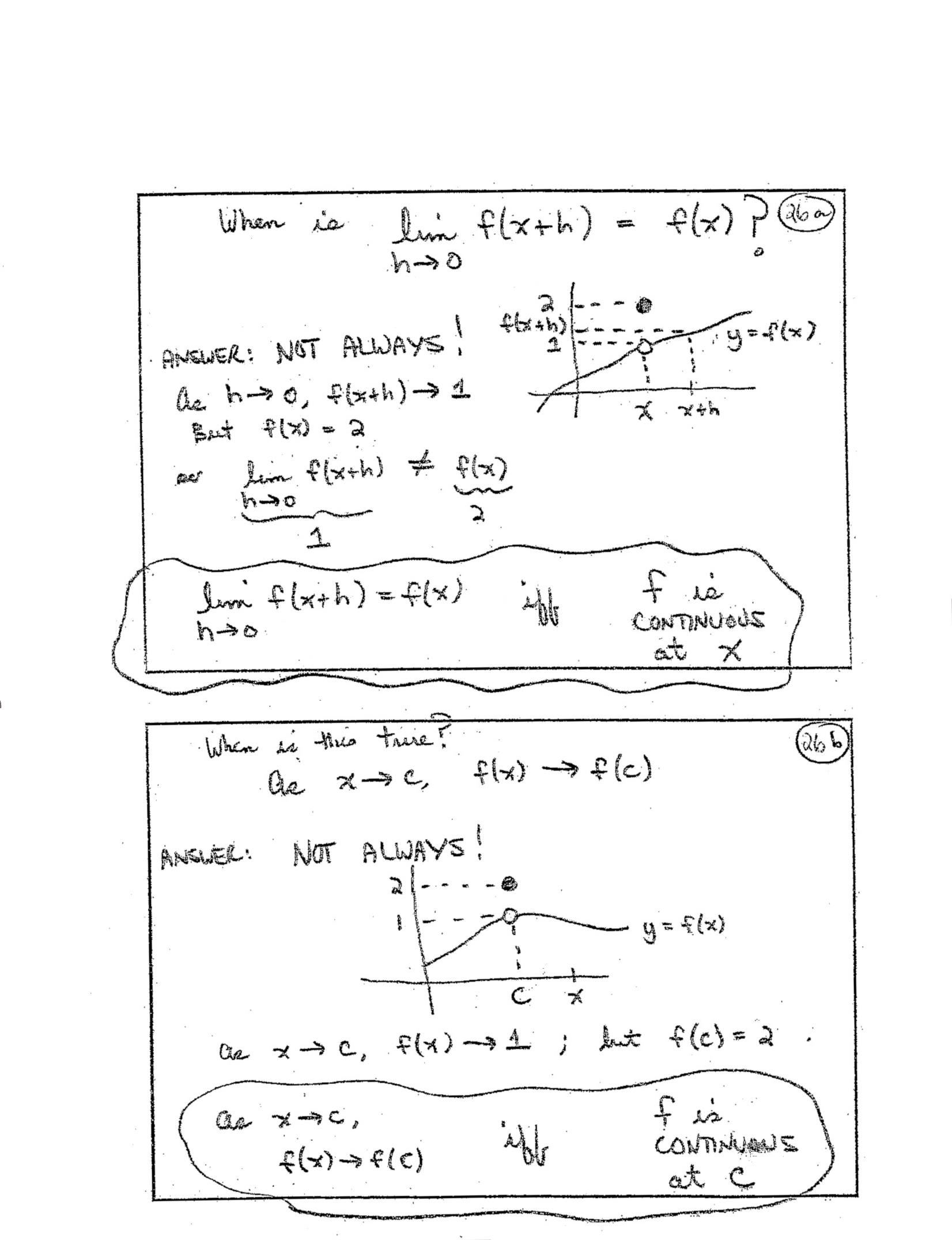27.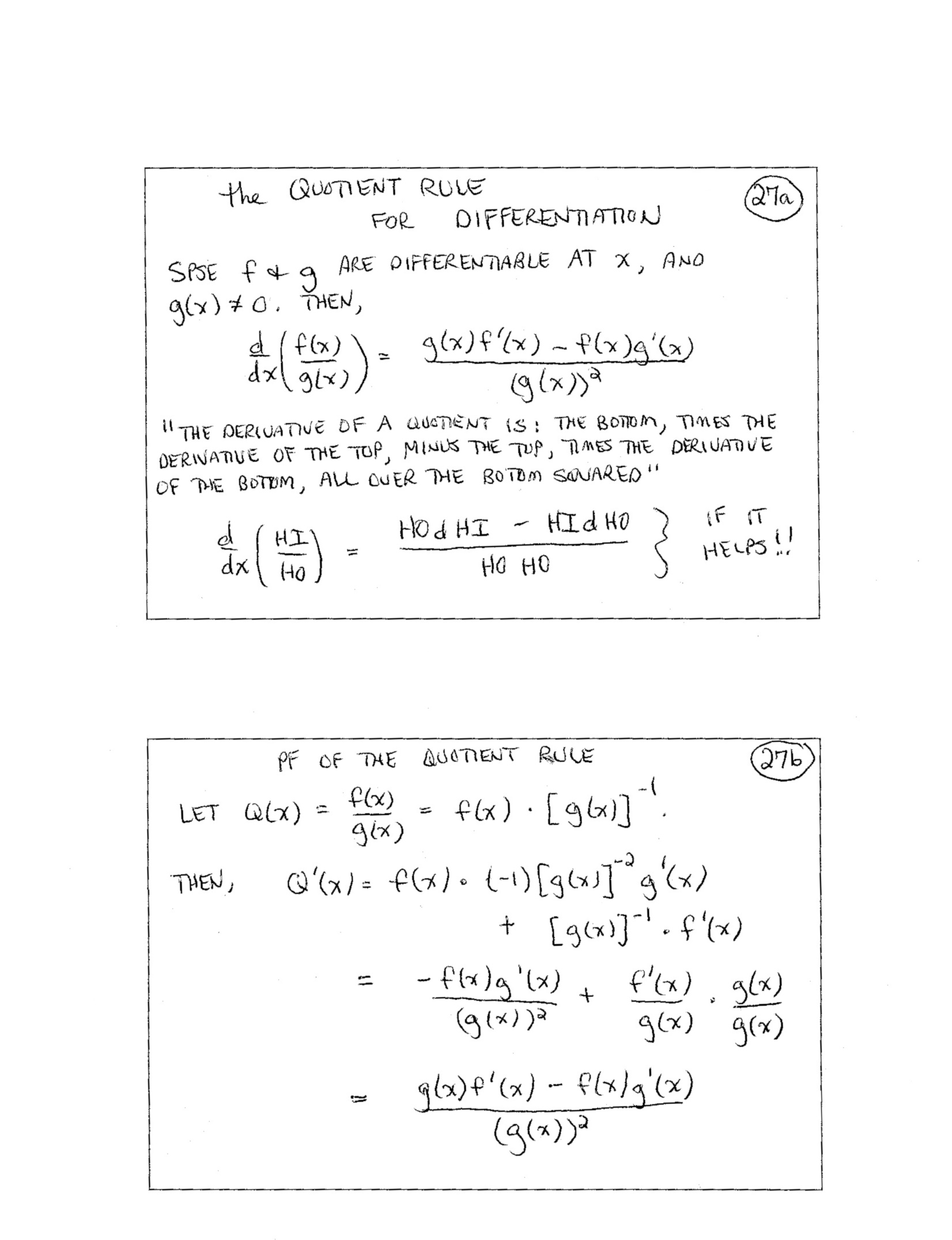28.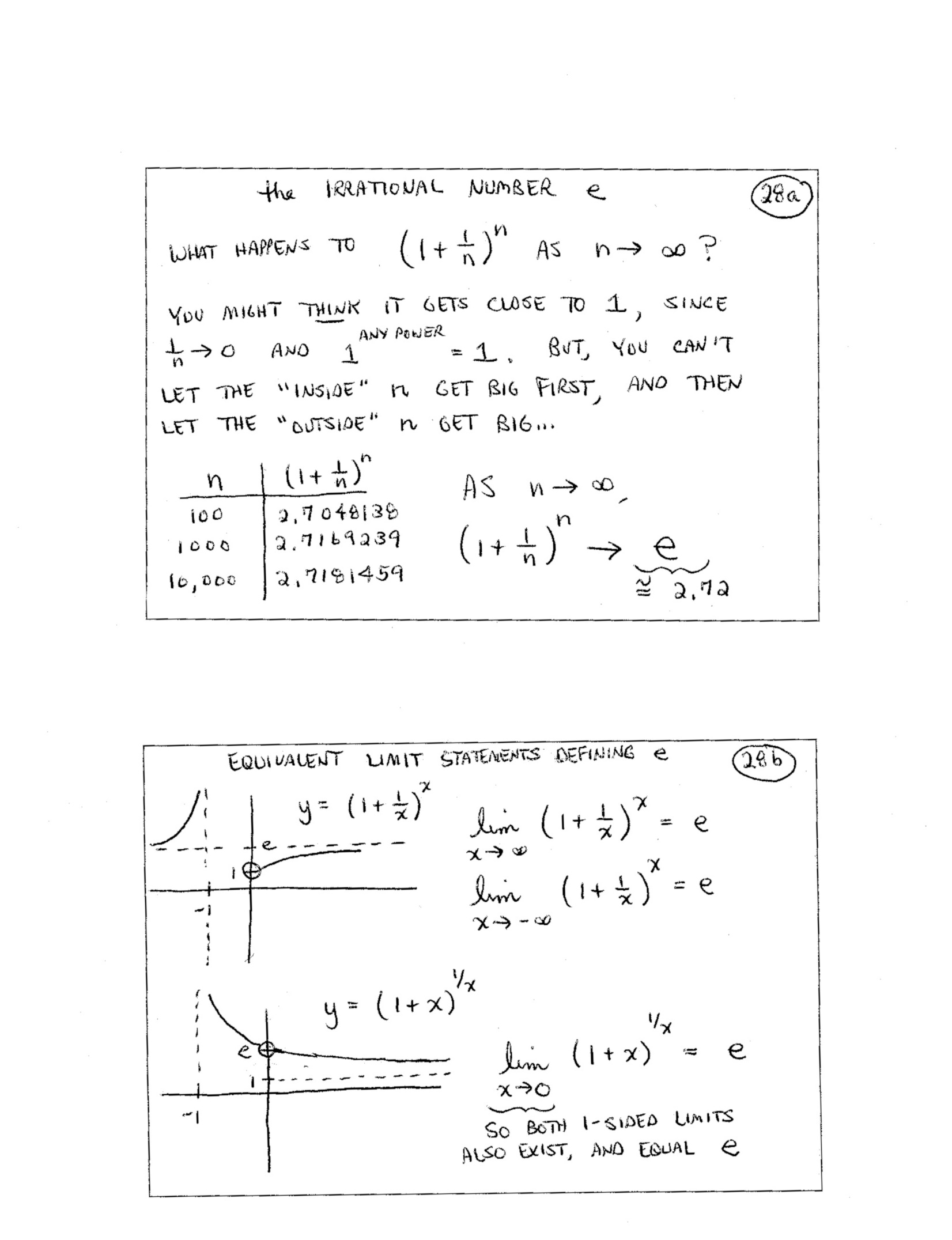29.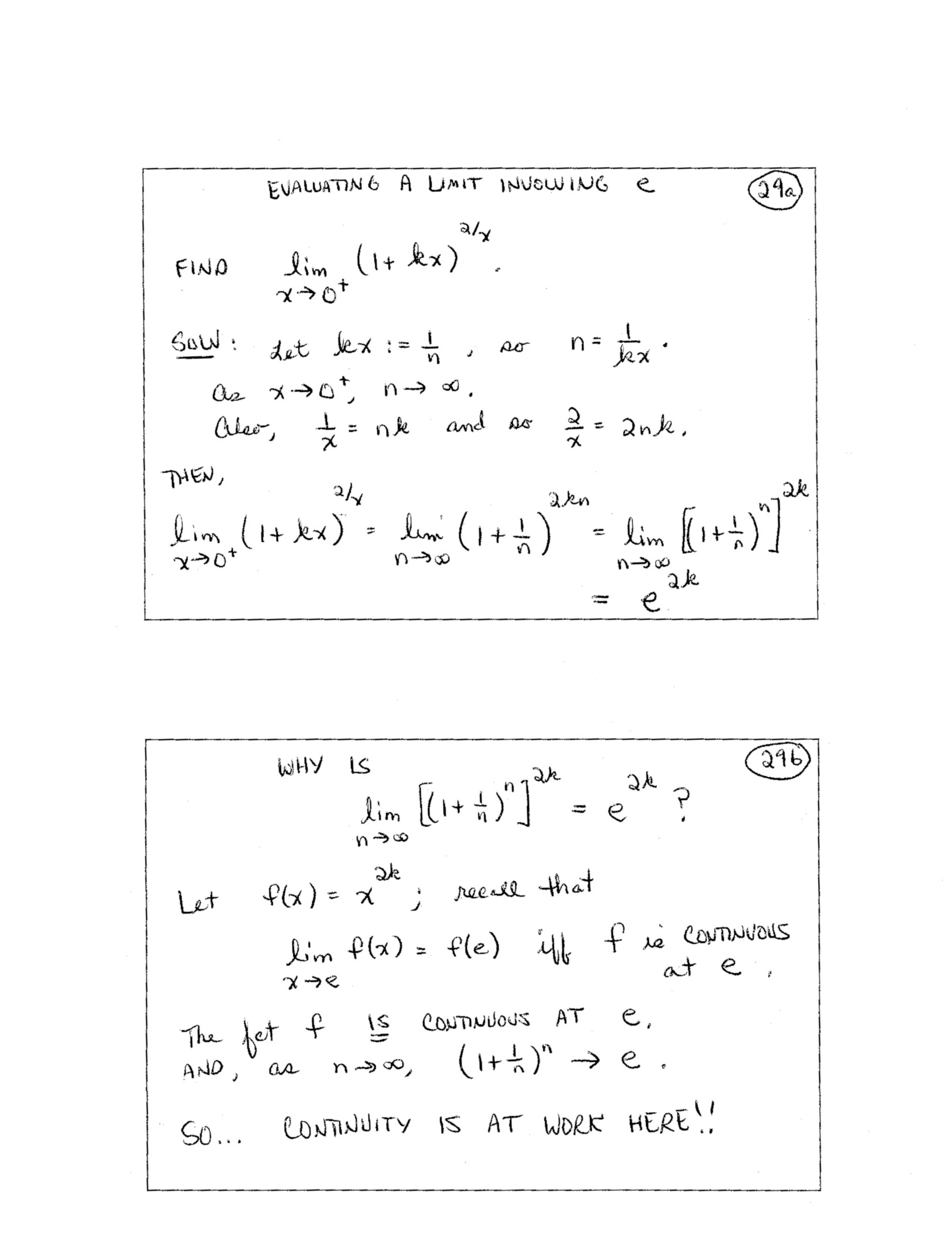(included forhigher-level course) 30.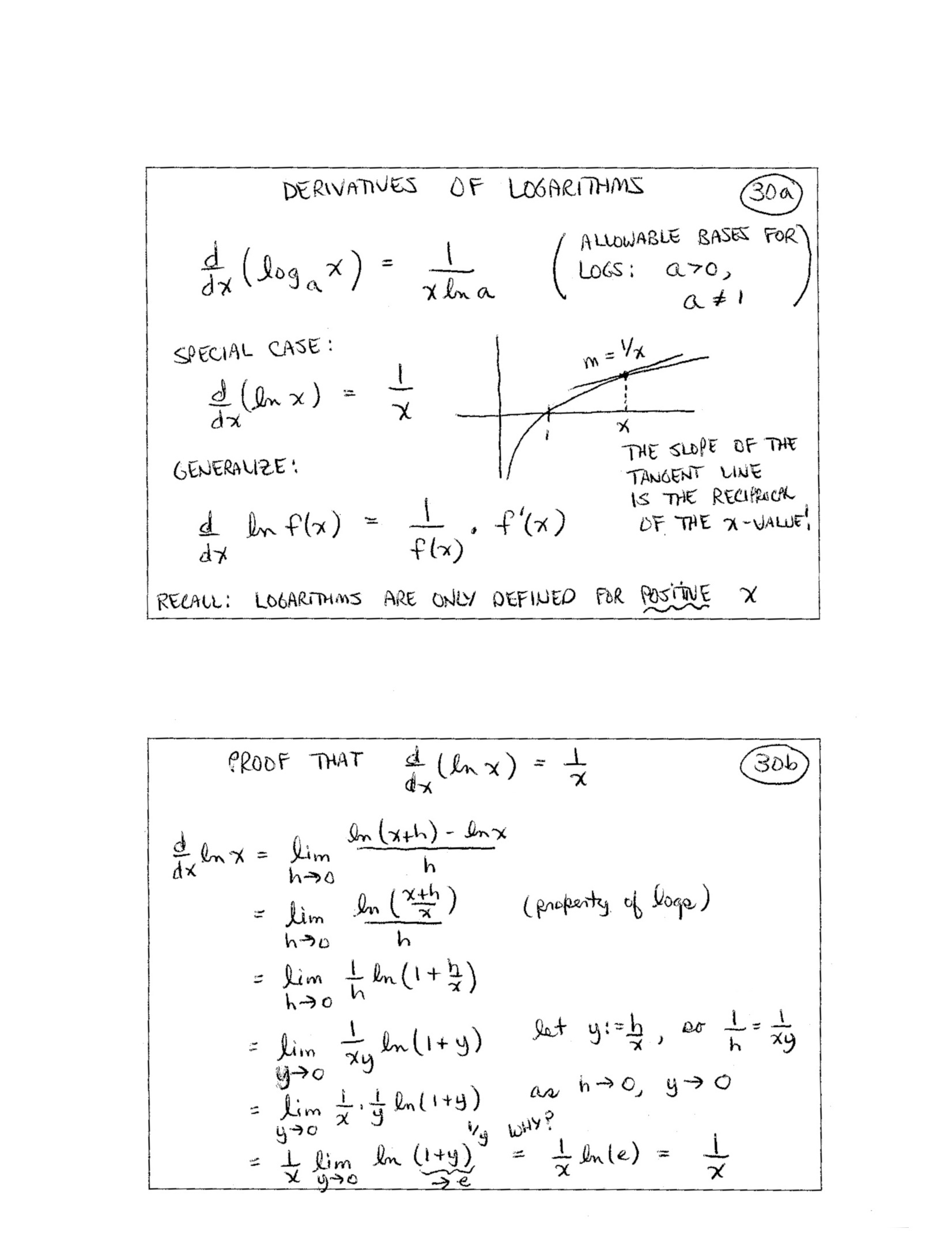31.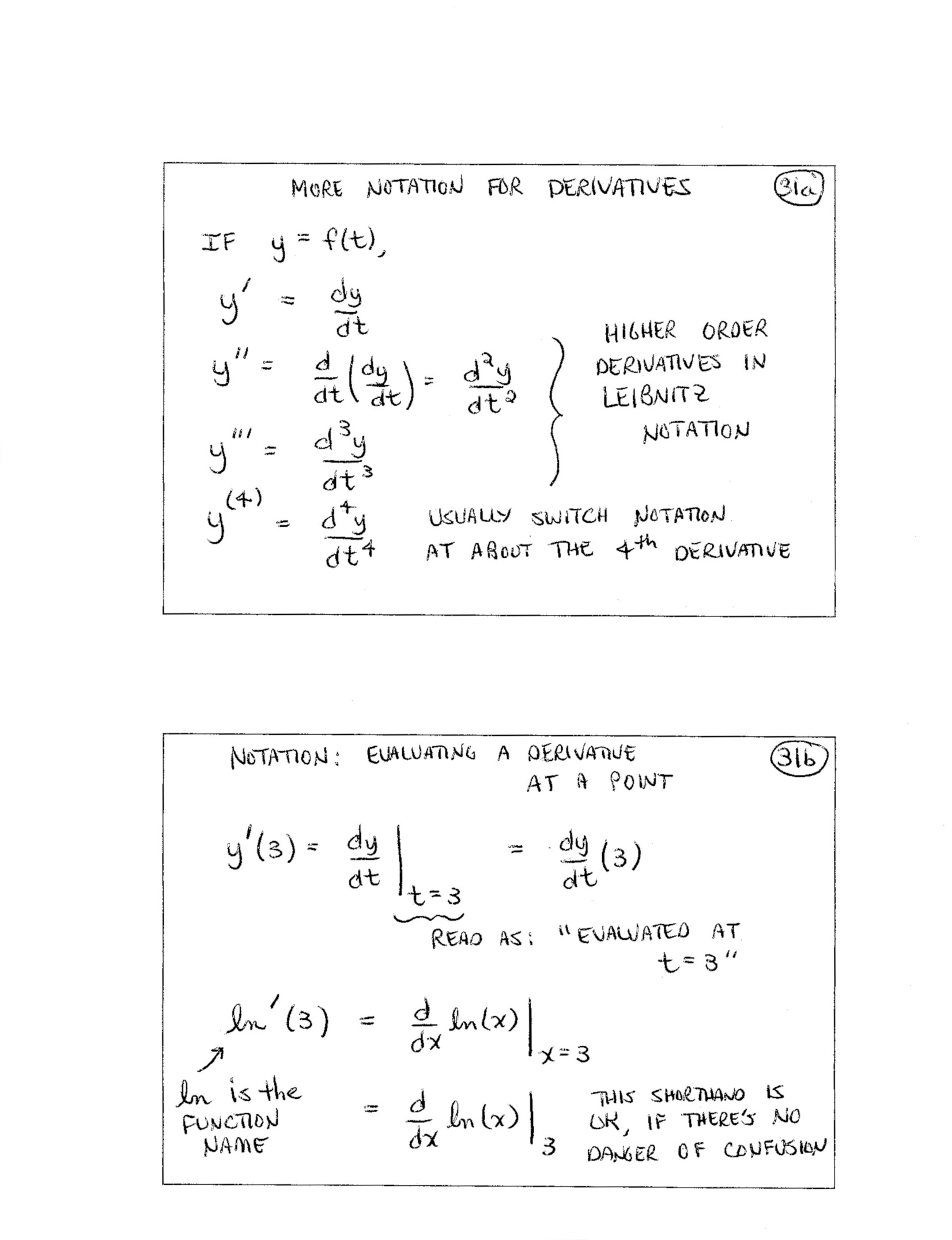32.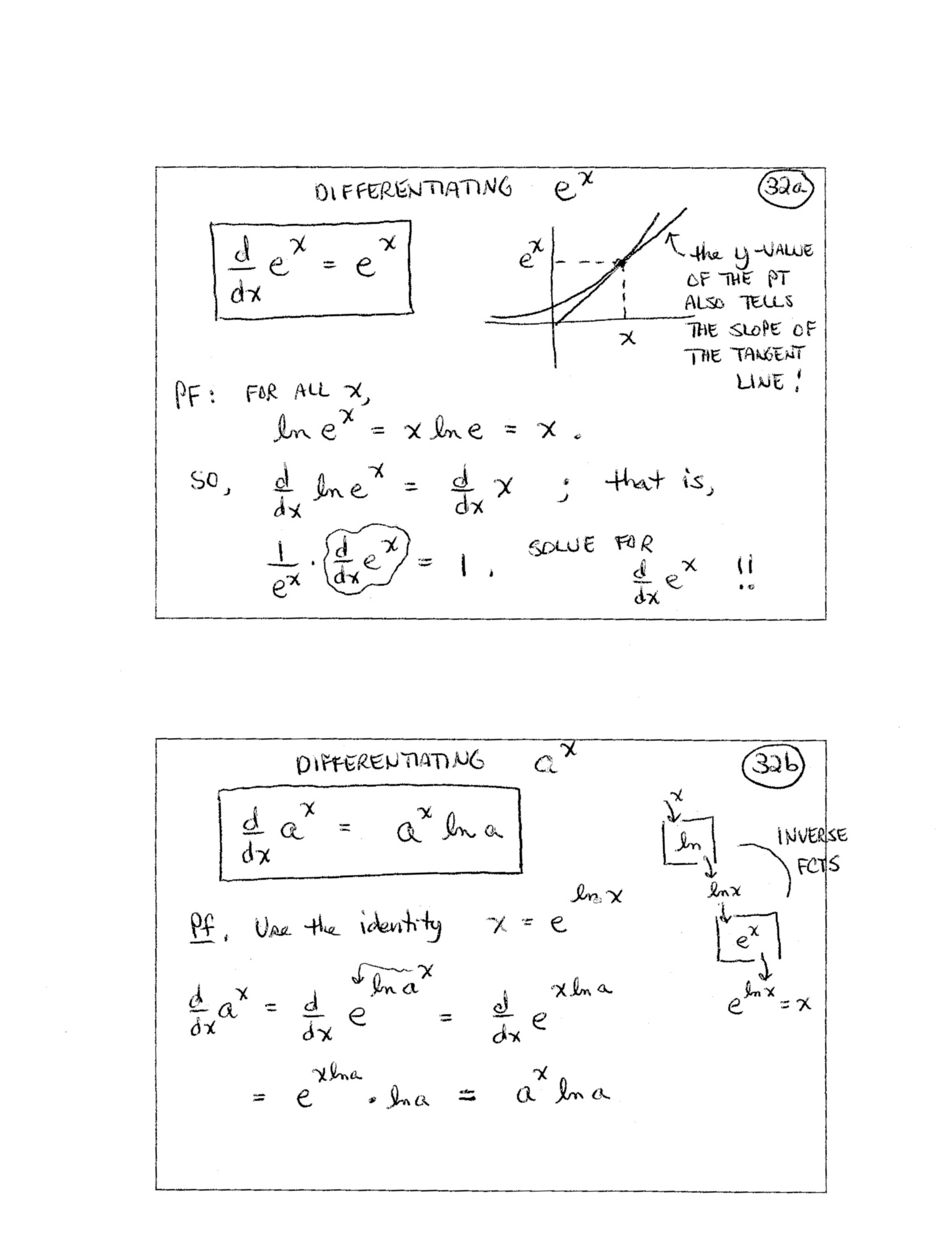33.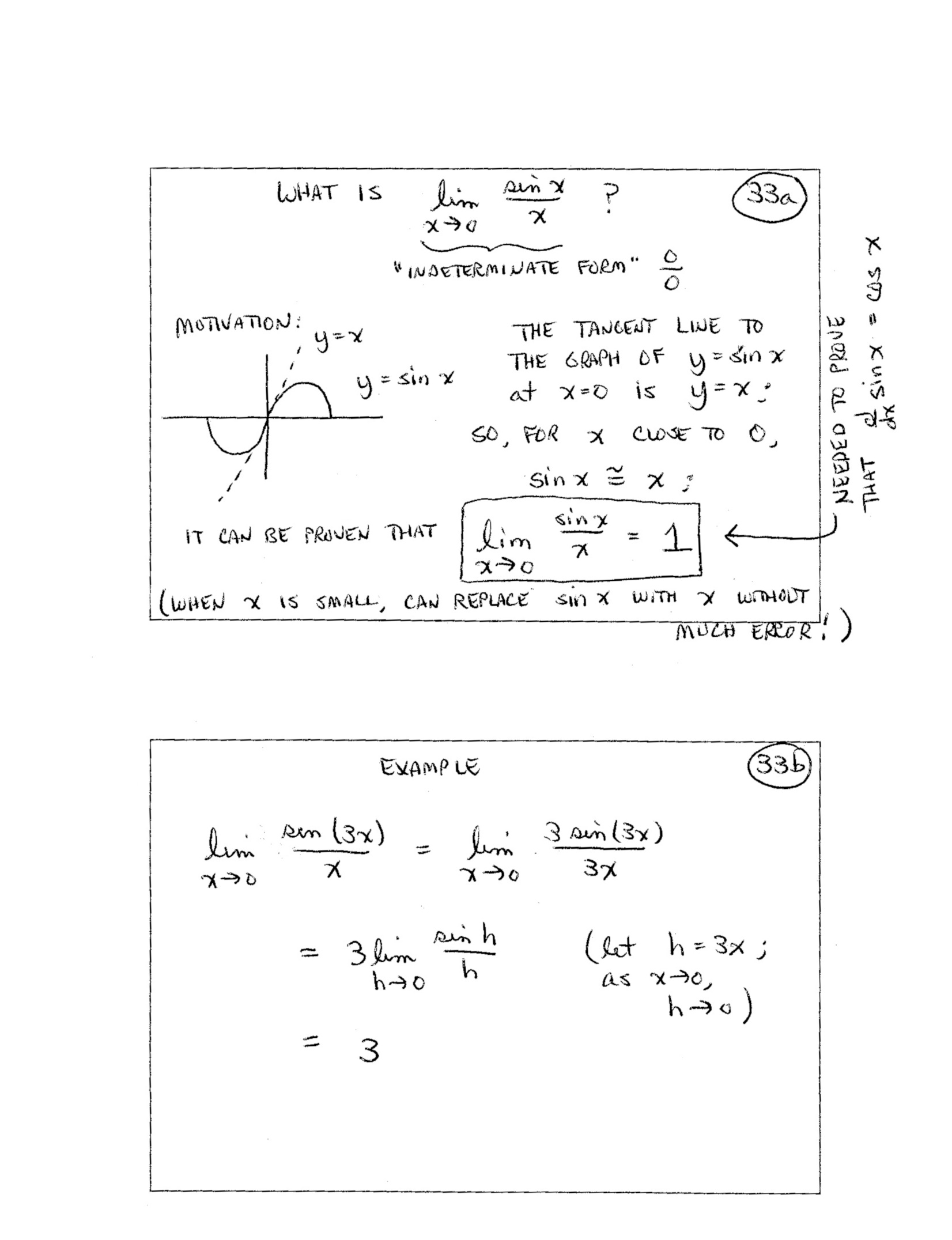(included forhigher-level course) 34.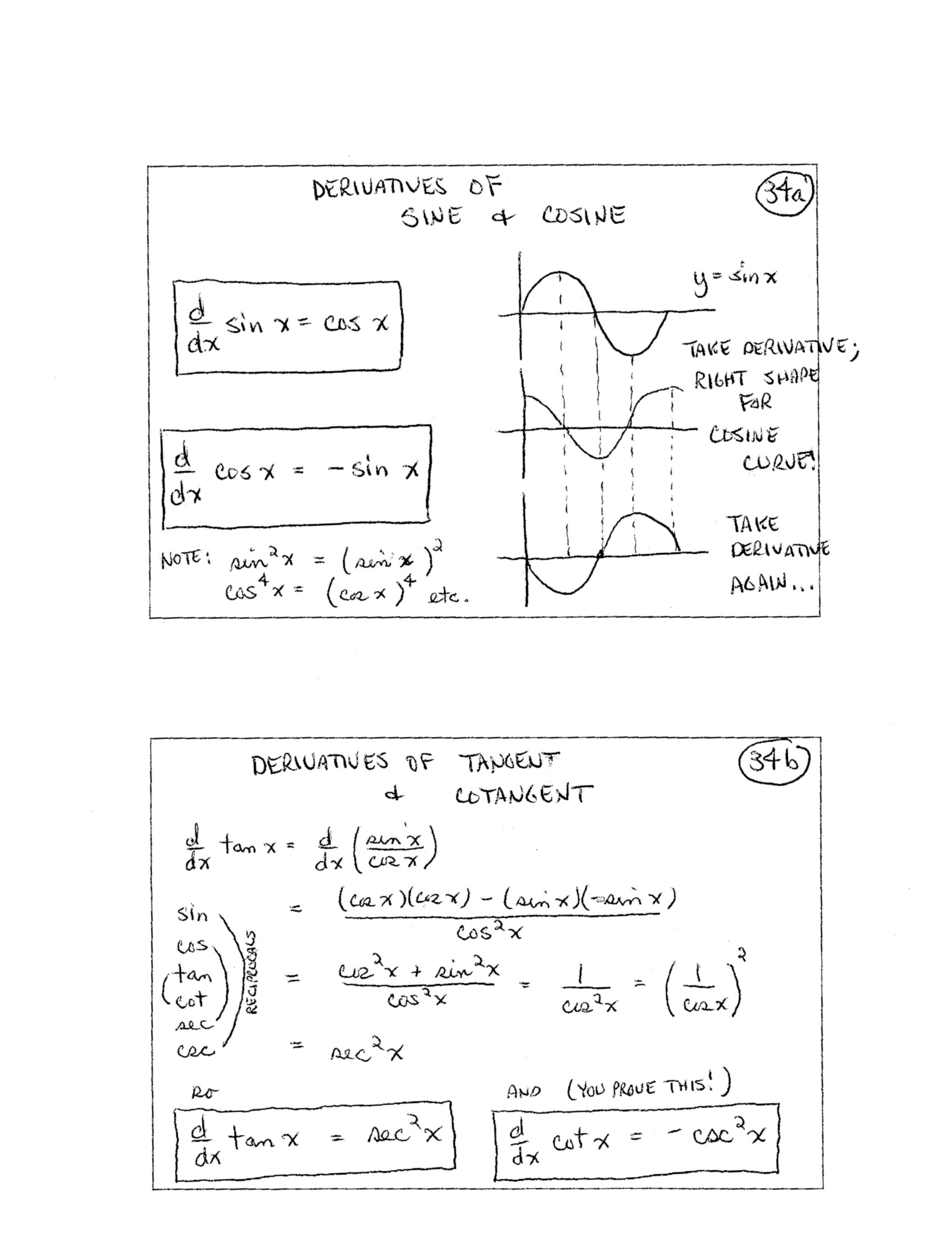(included forhigher-level course) 35.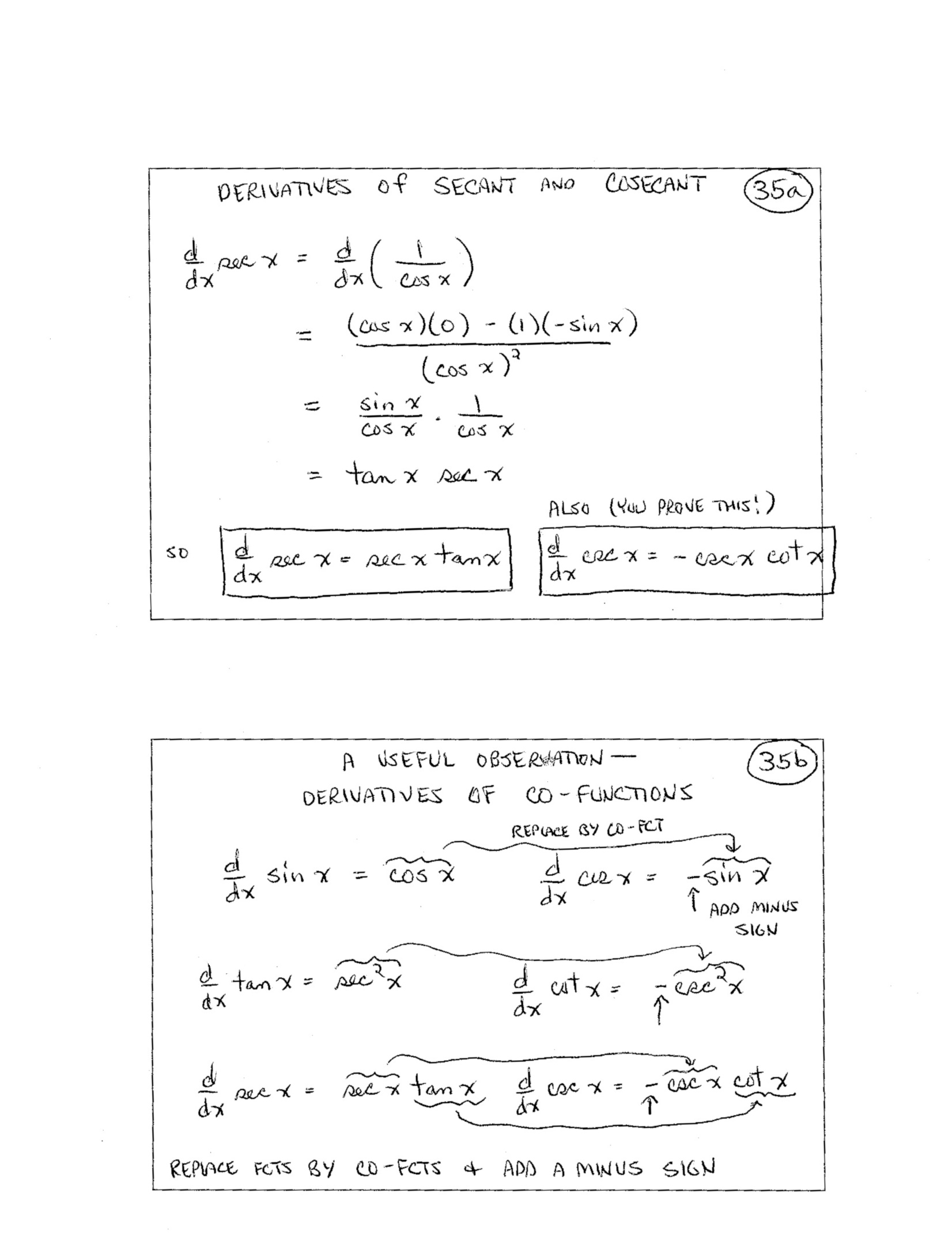(included forhigher-level course) 36.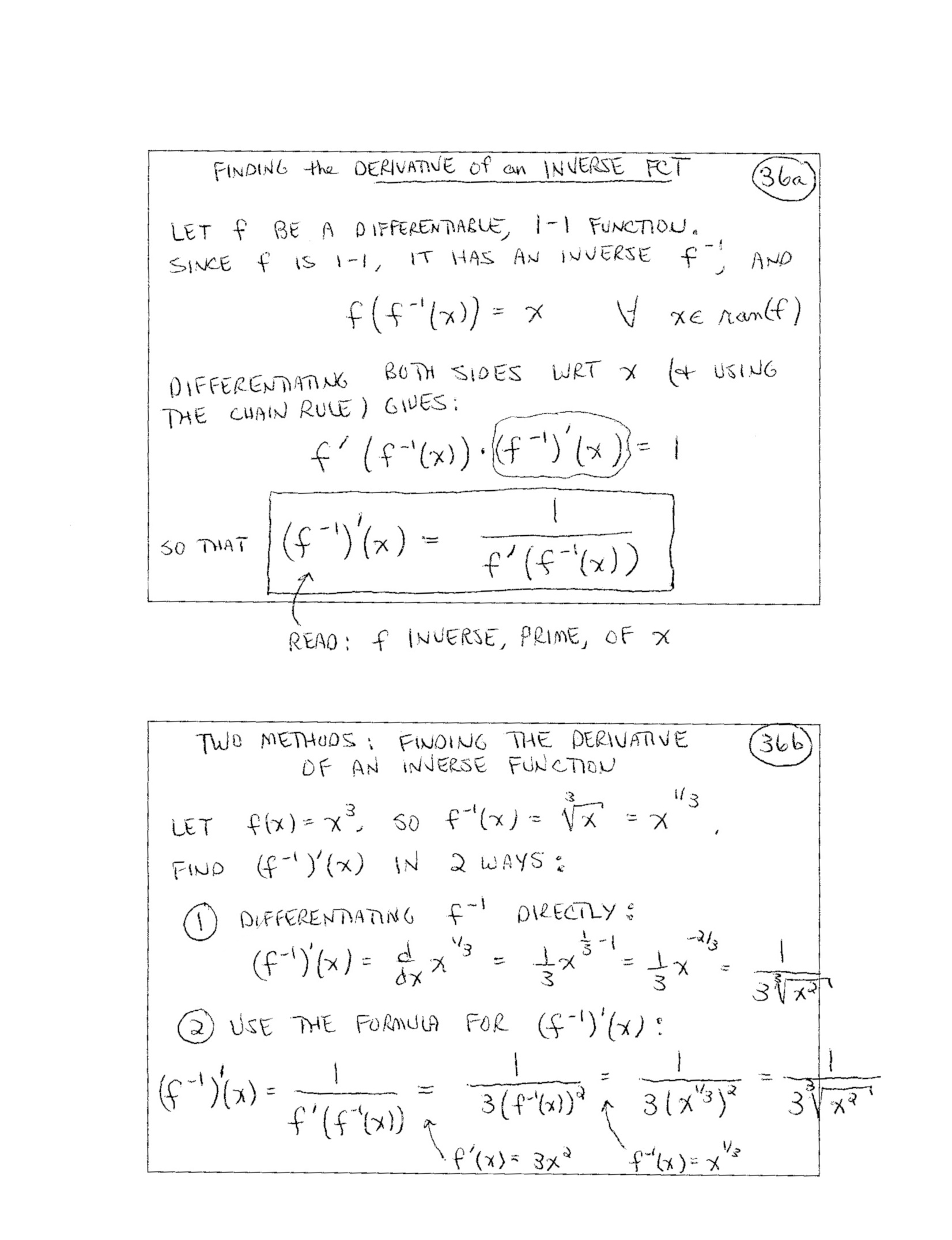(included forhigher-level course) 37.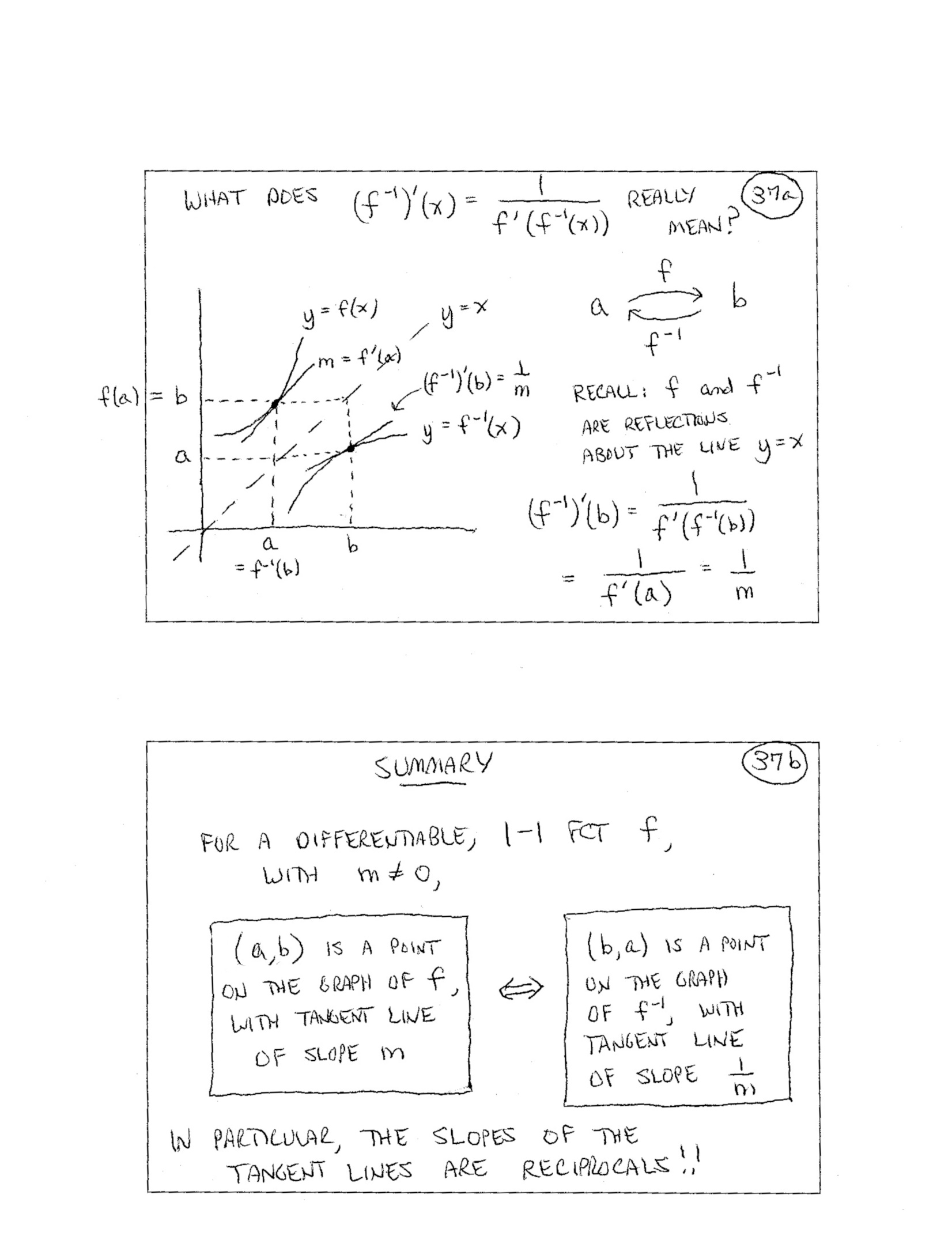(included forhigher-level course) 38.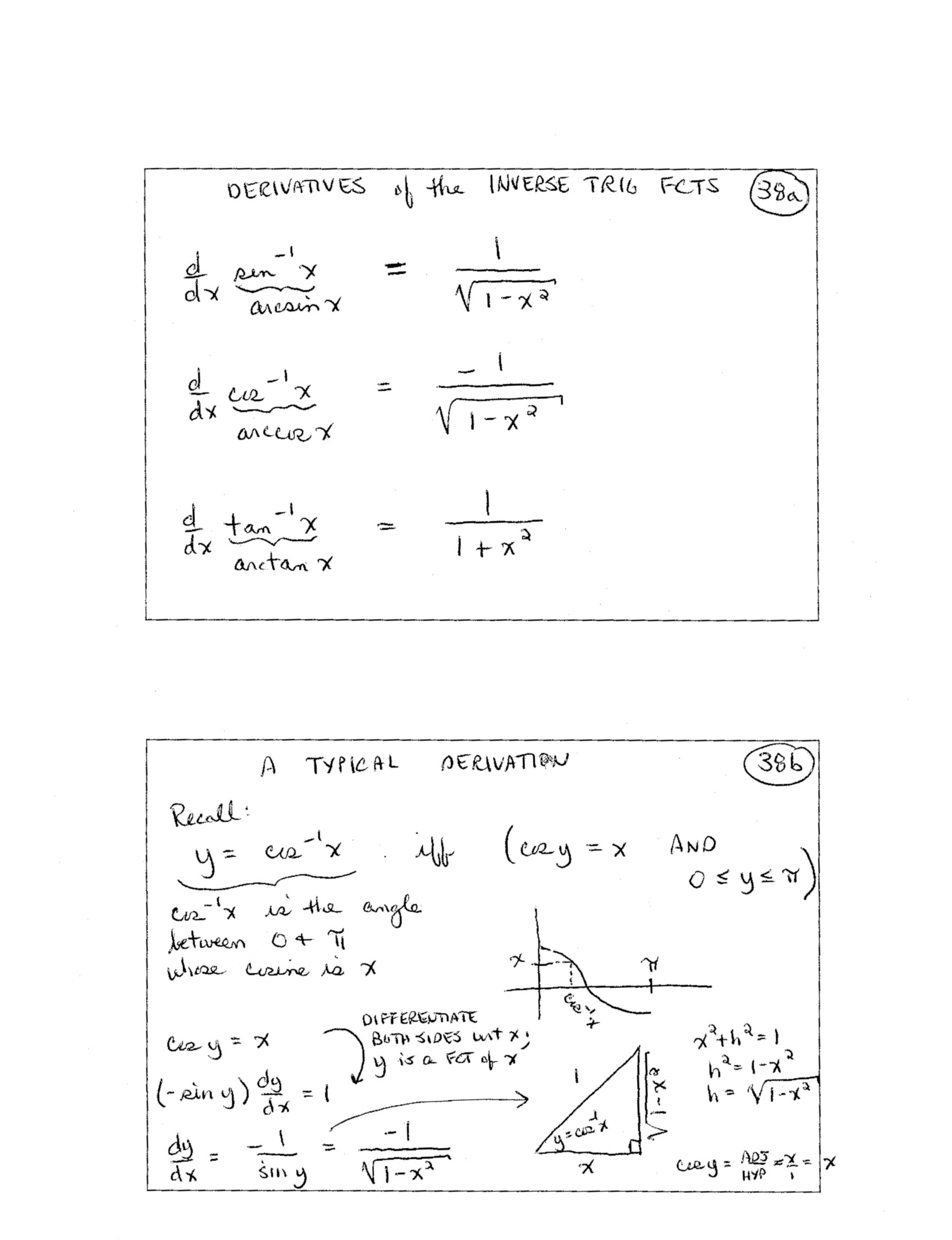(included forhigher-level course) 39.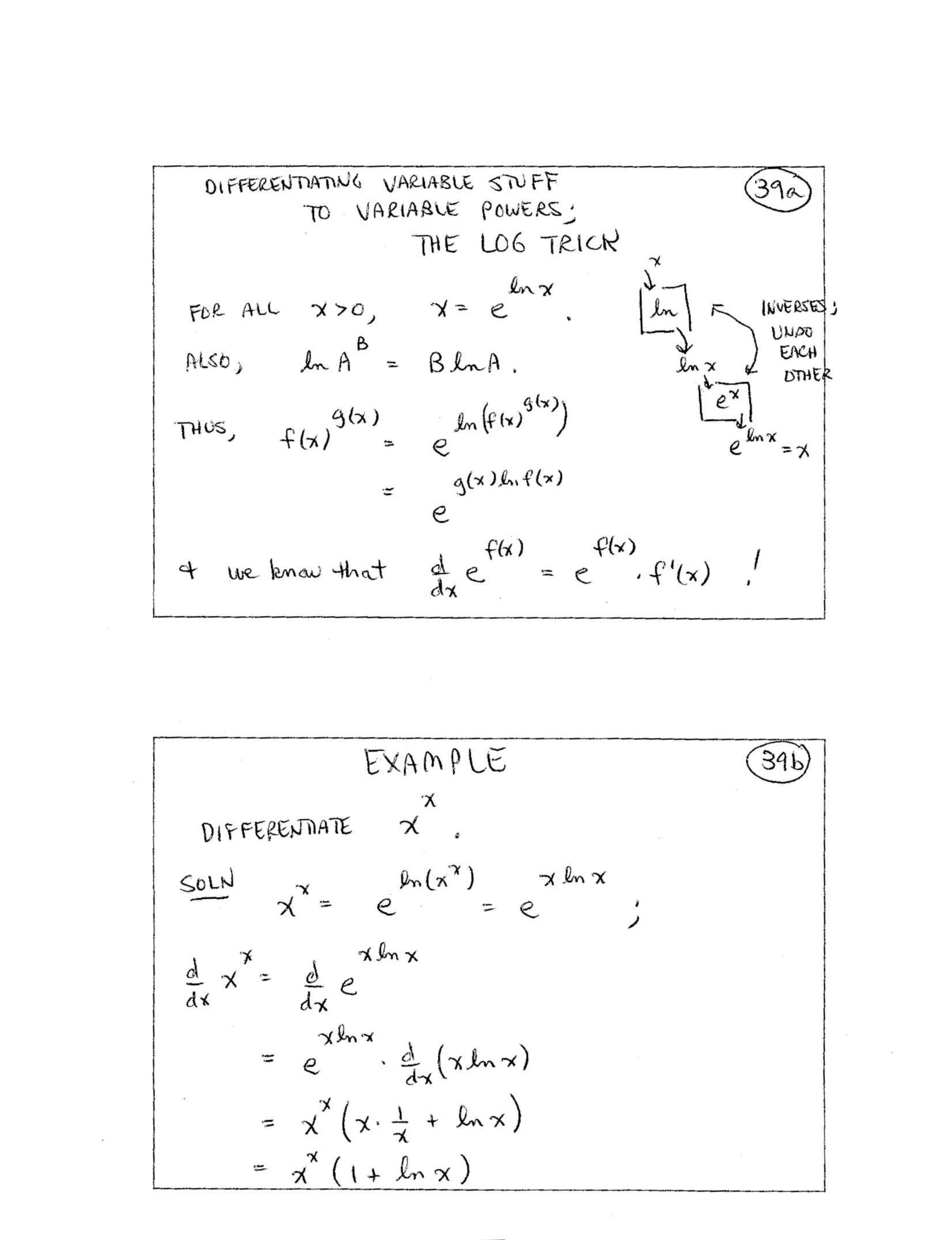40.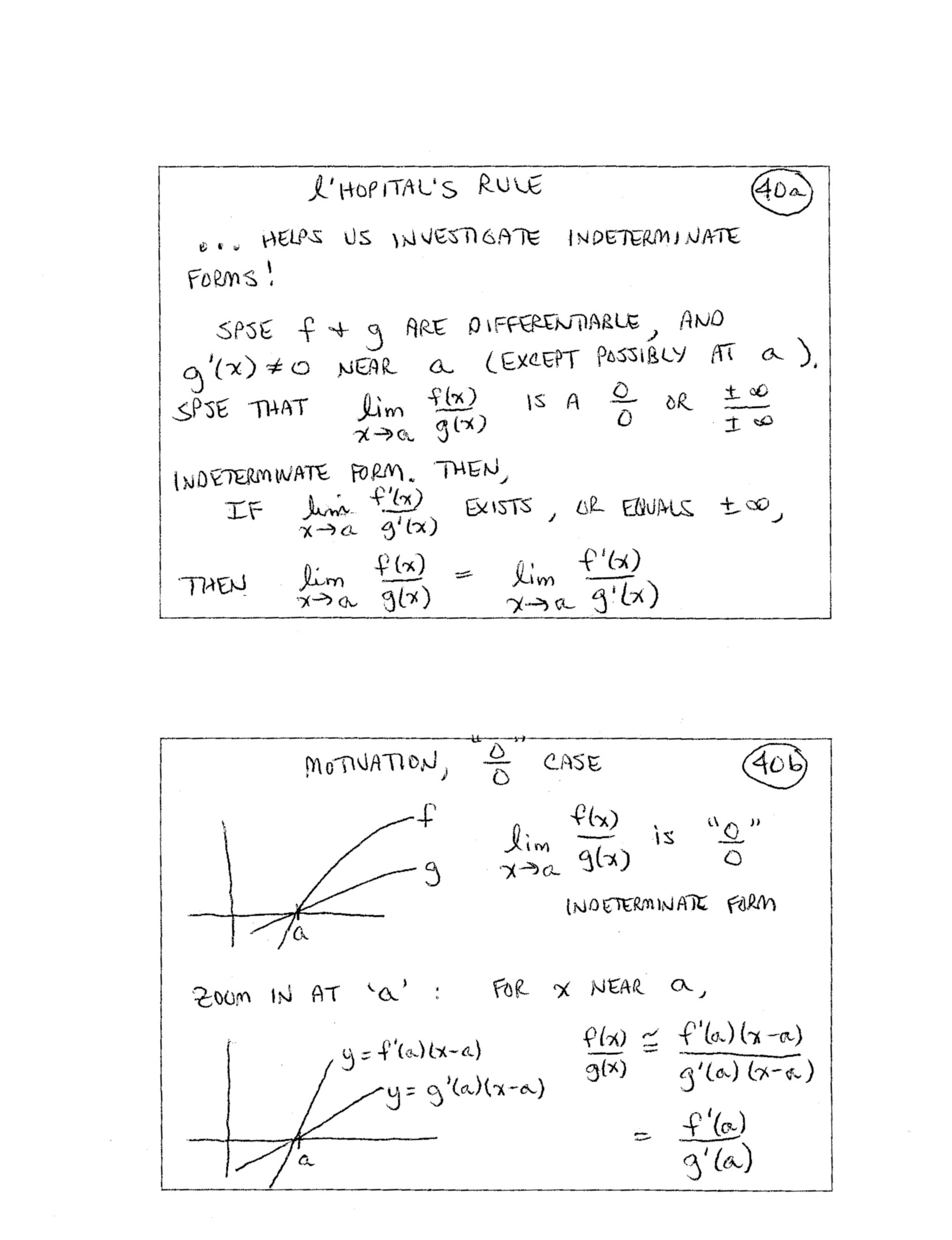(included forhigher-level course) 41.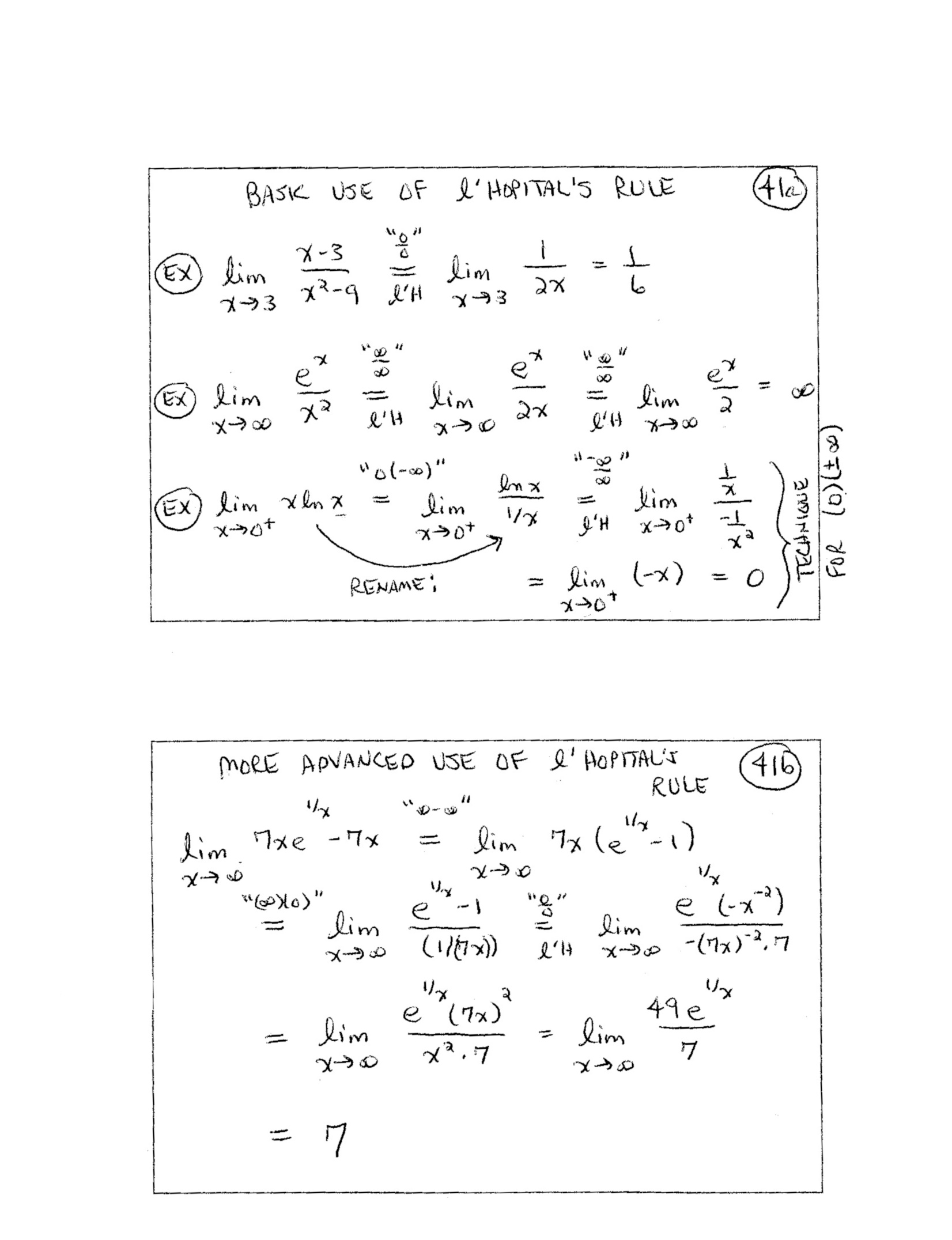(included forhigher-level course) 42.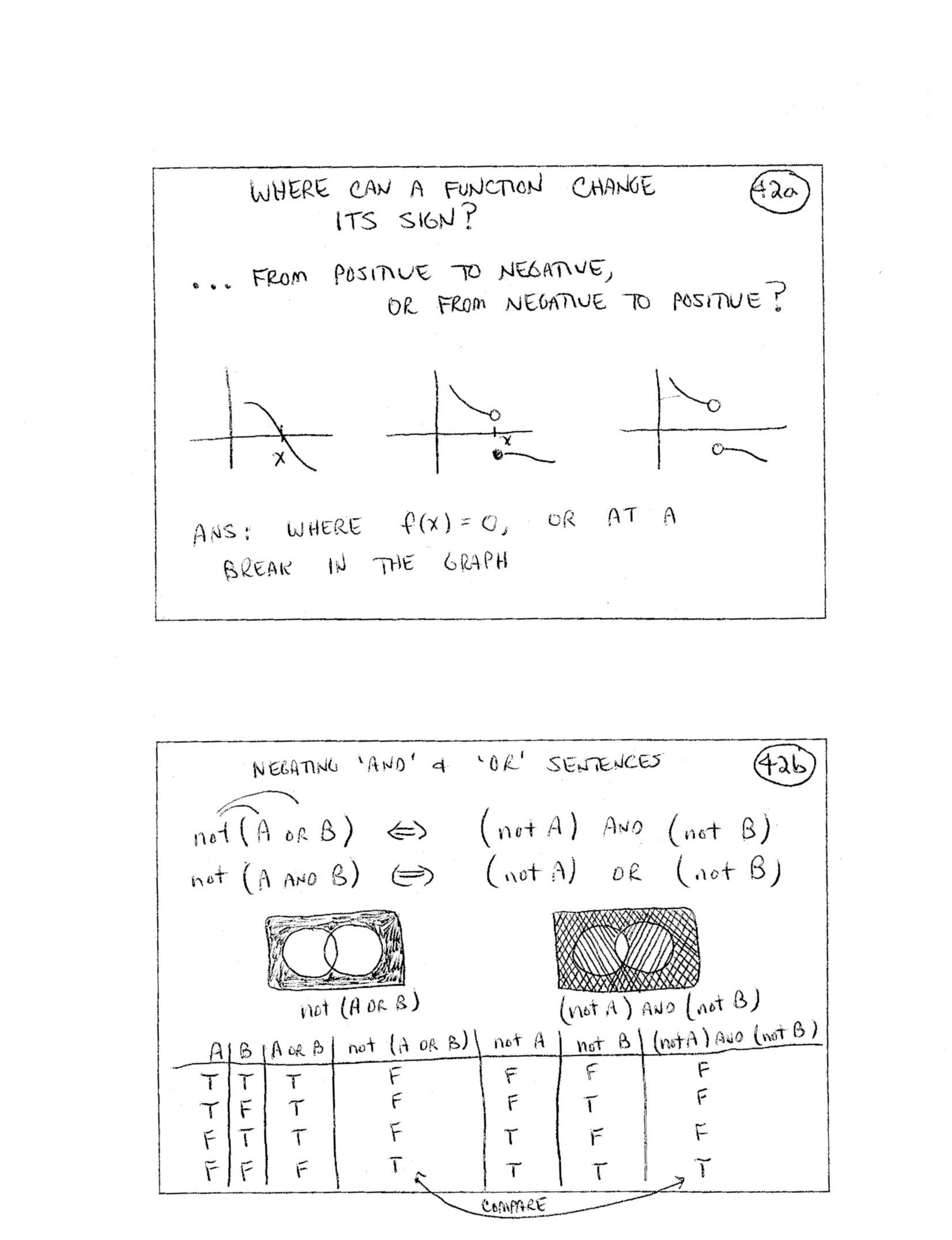43ab.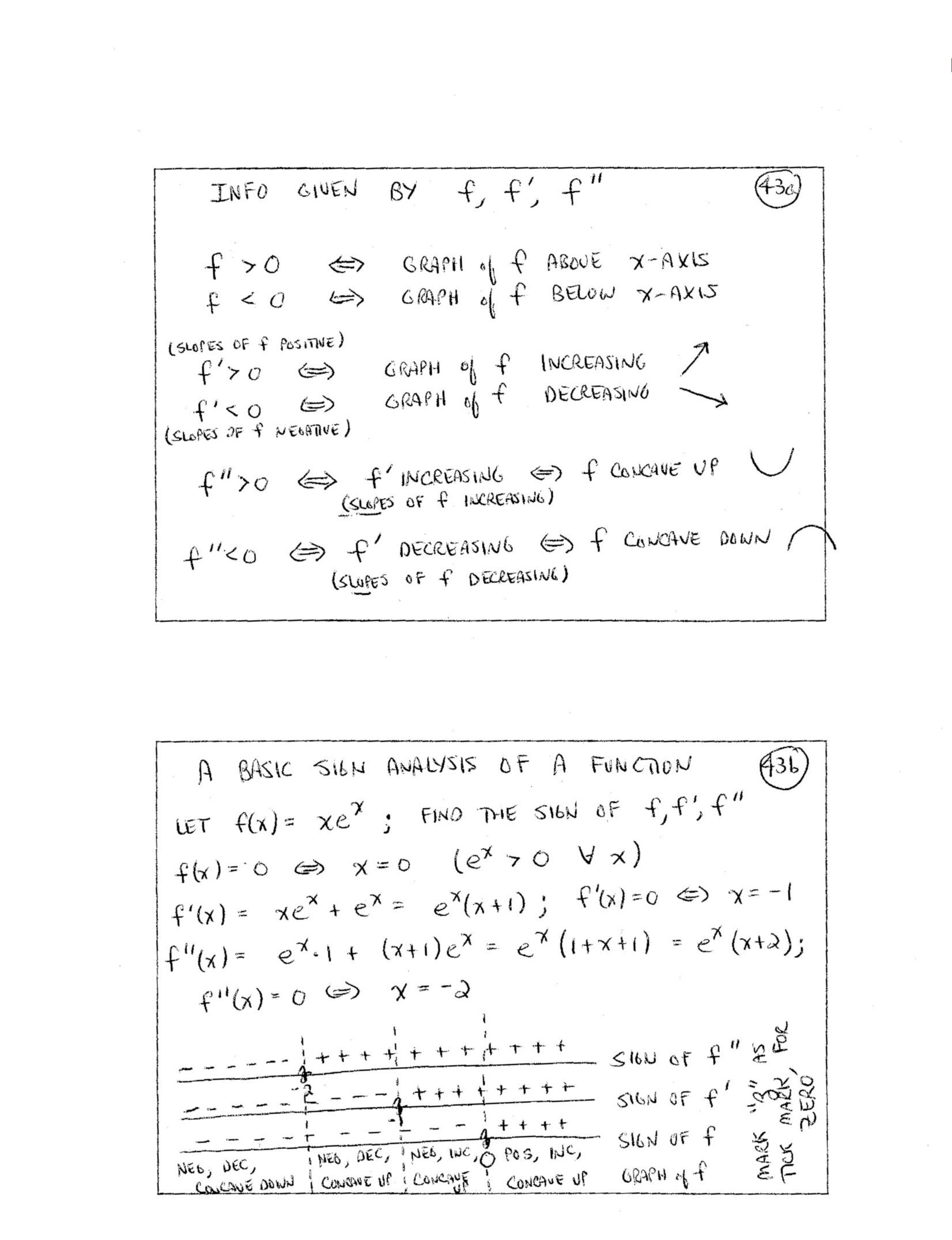43cd.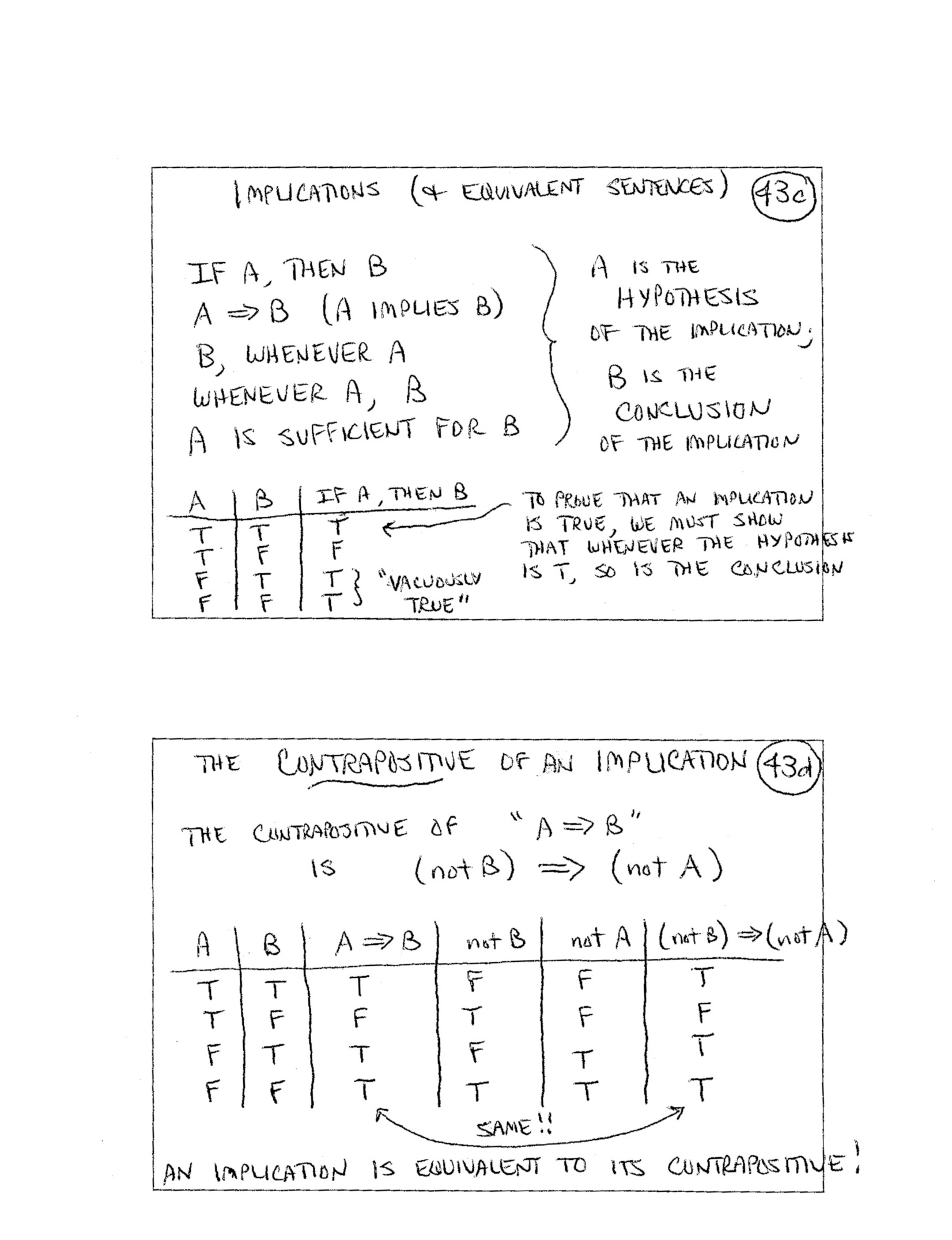44.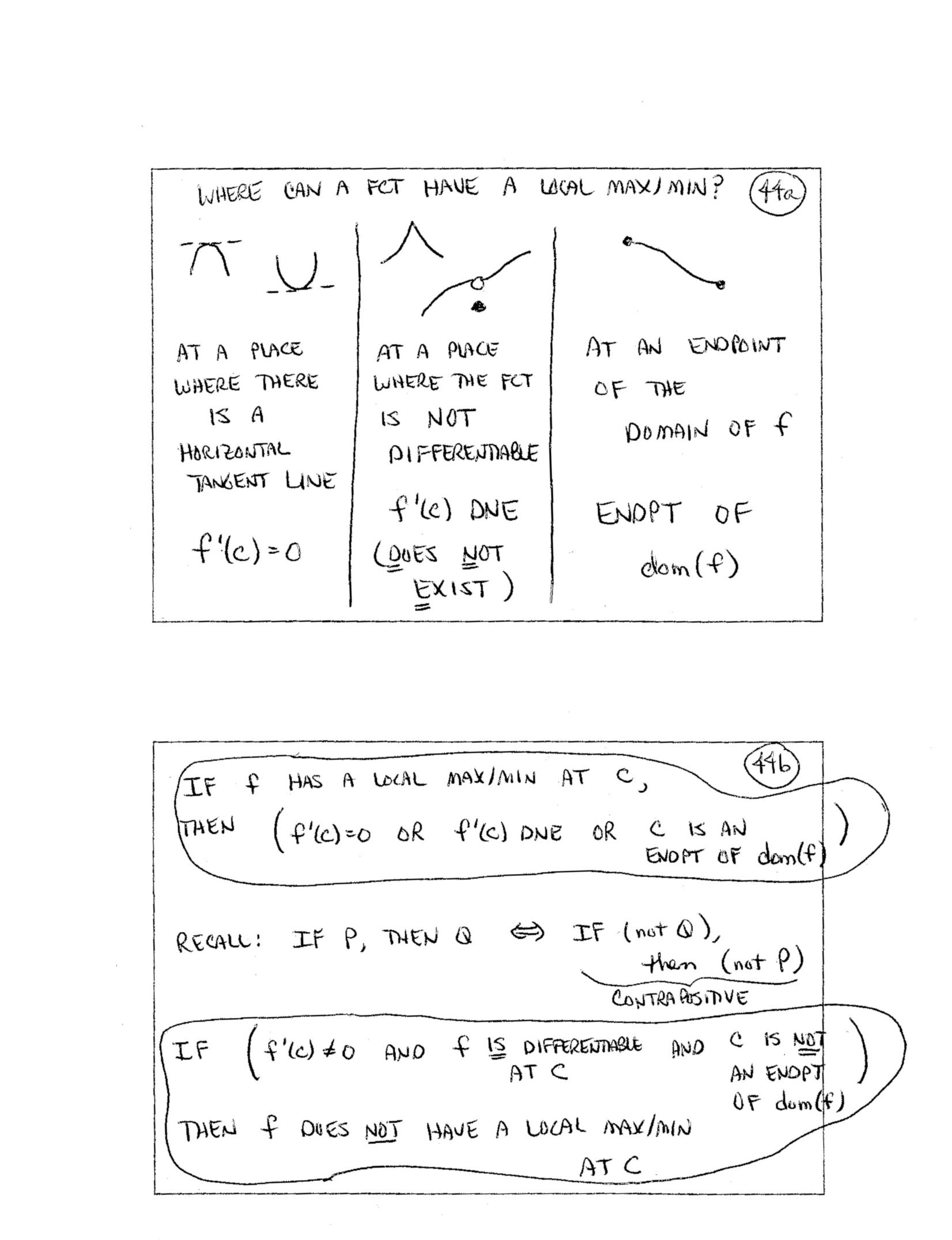45.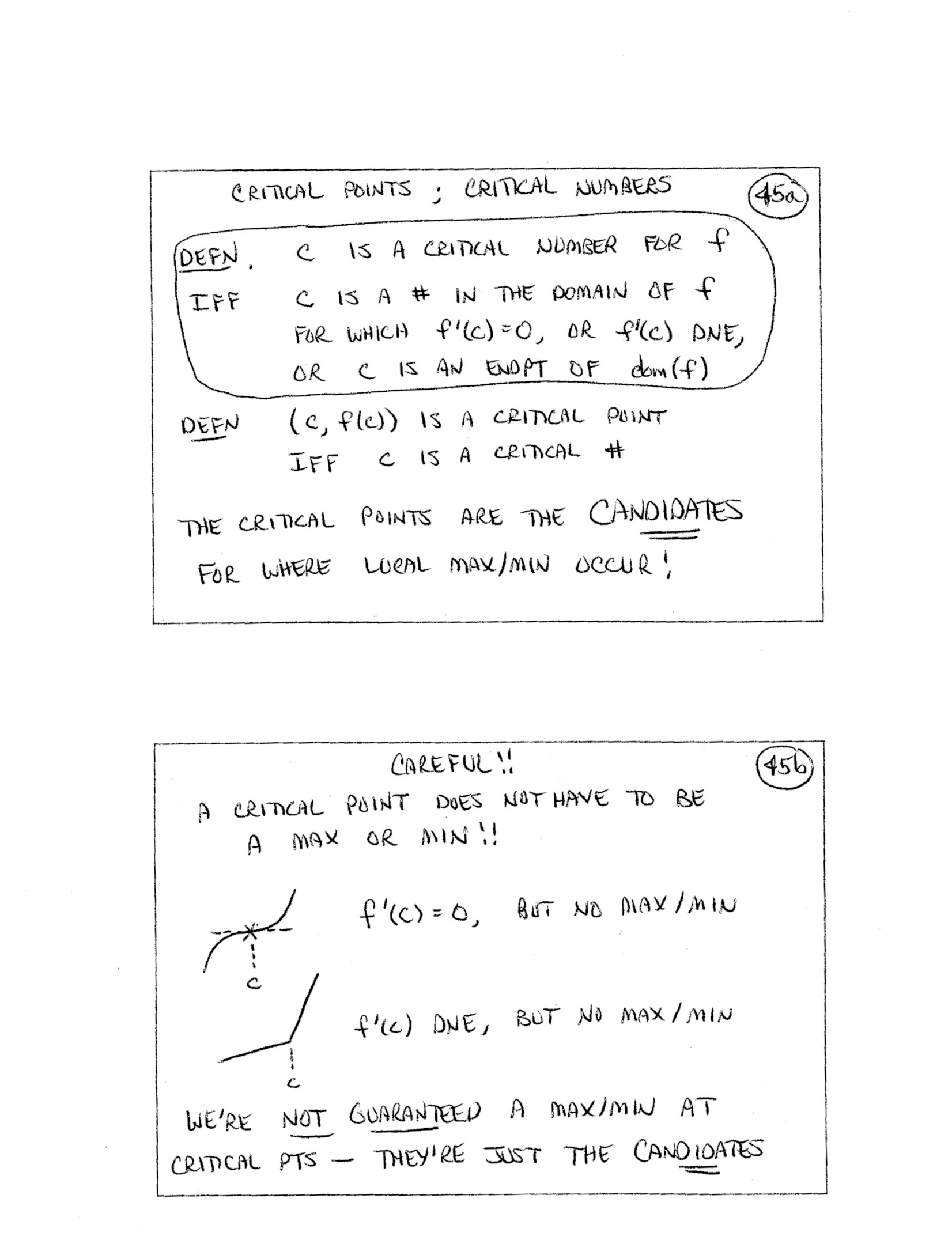46.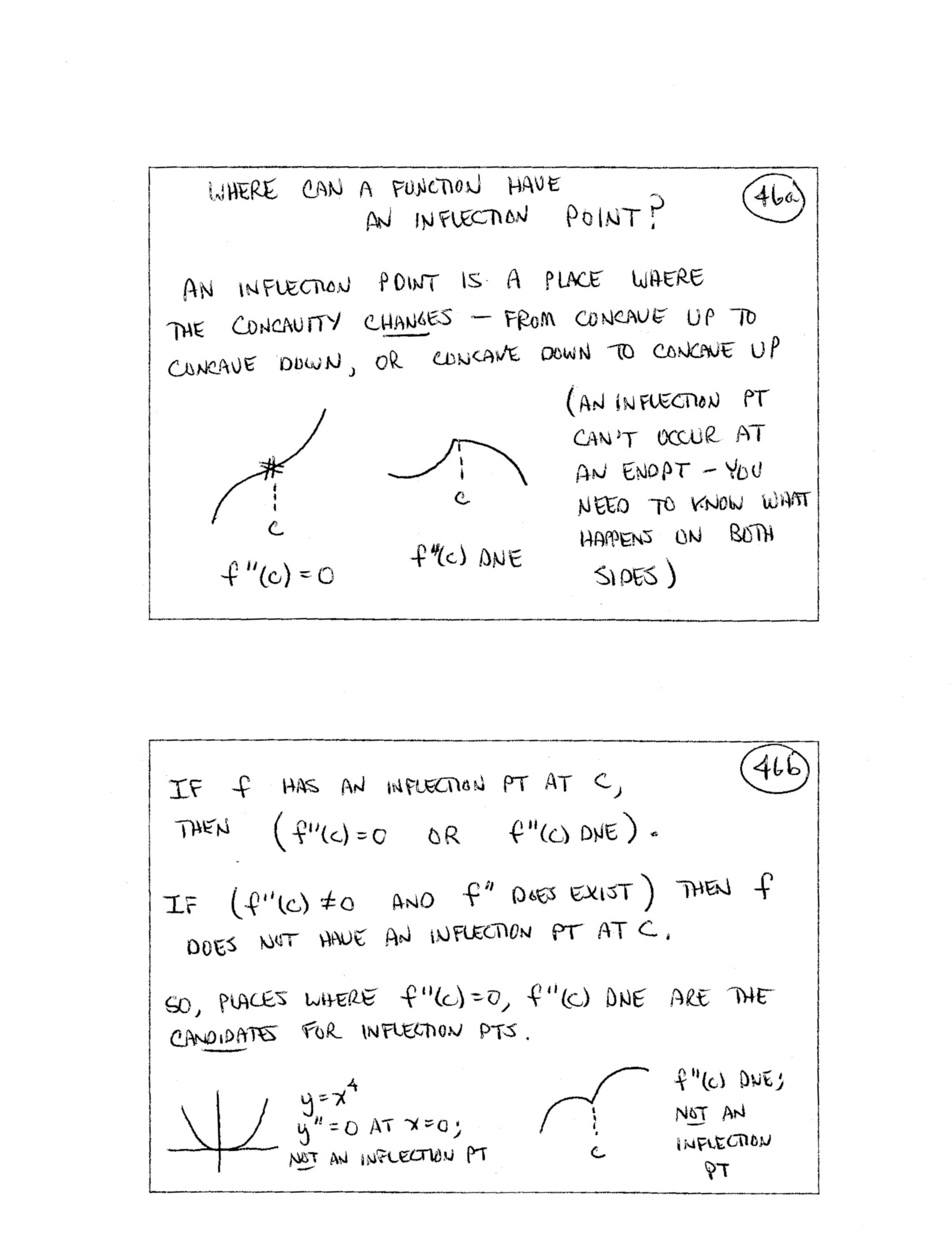47.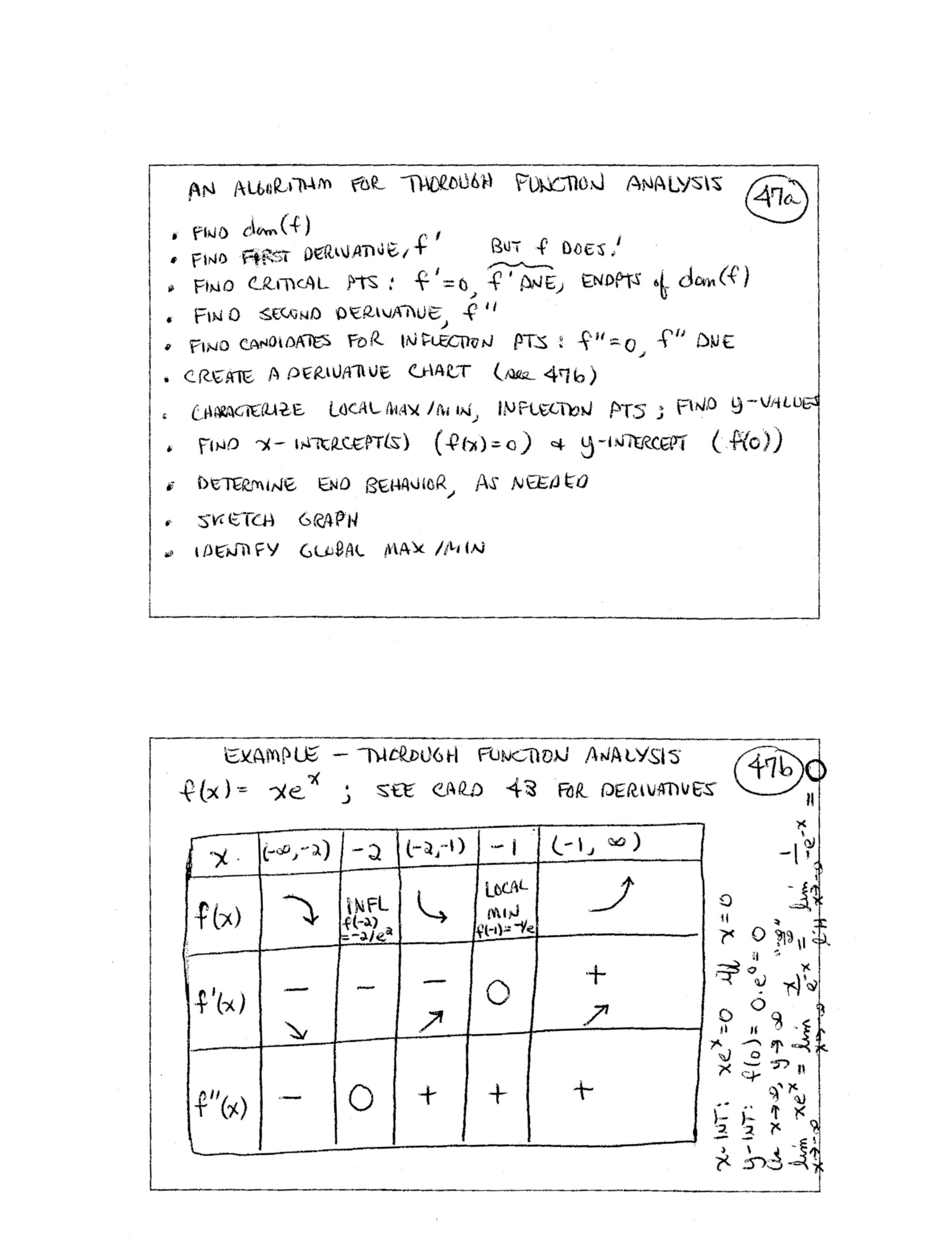48.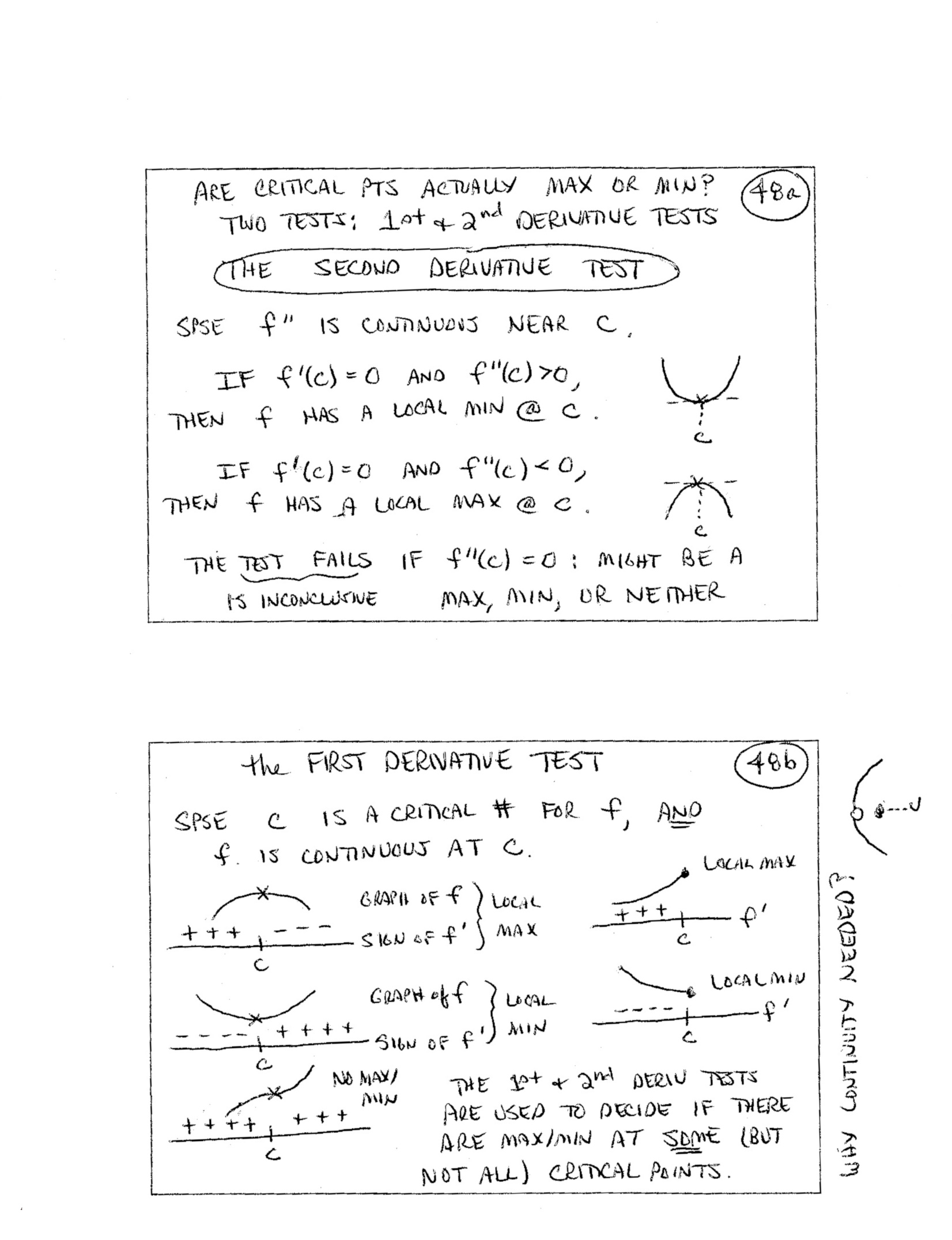49.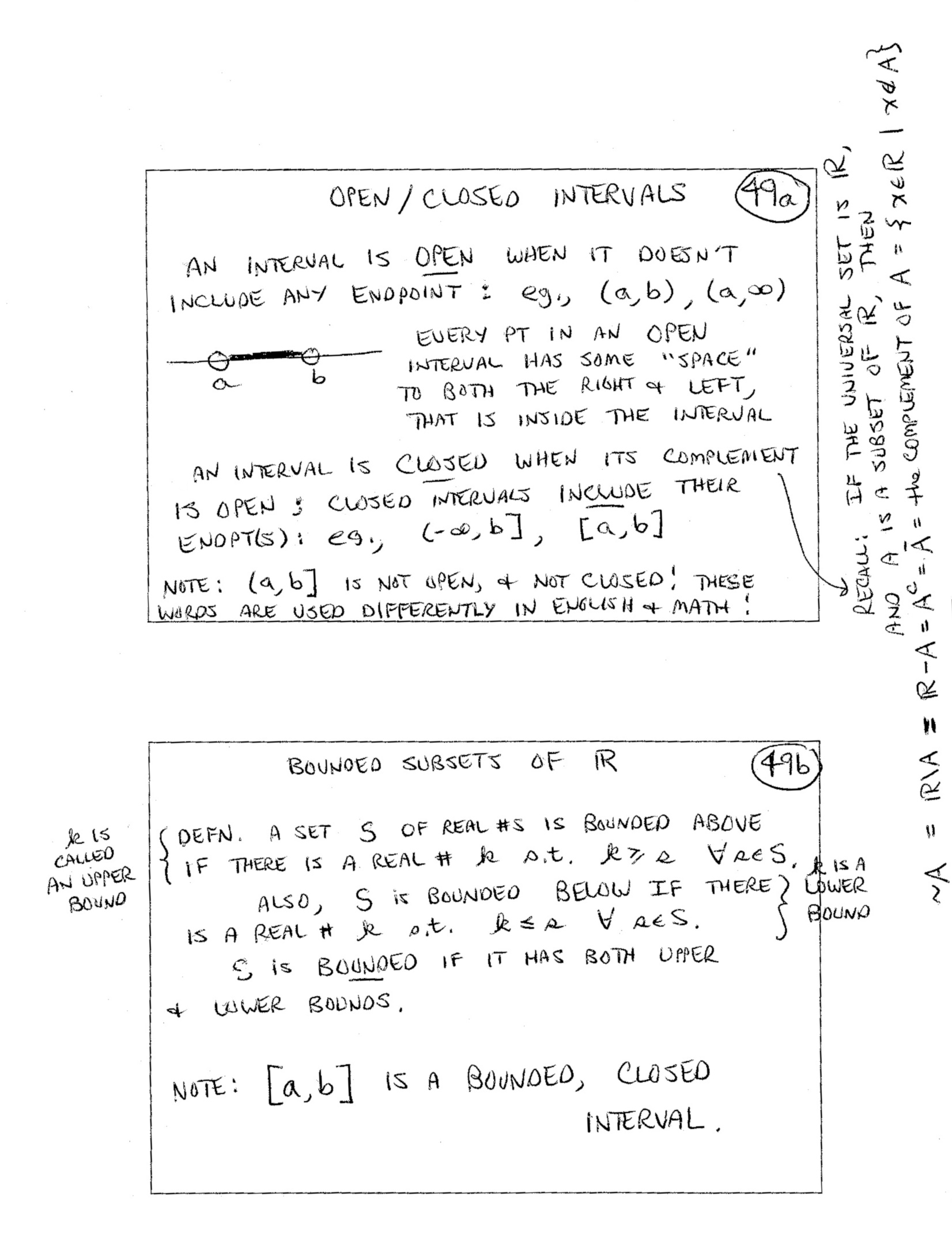50.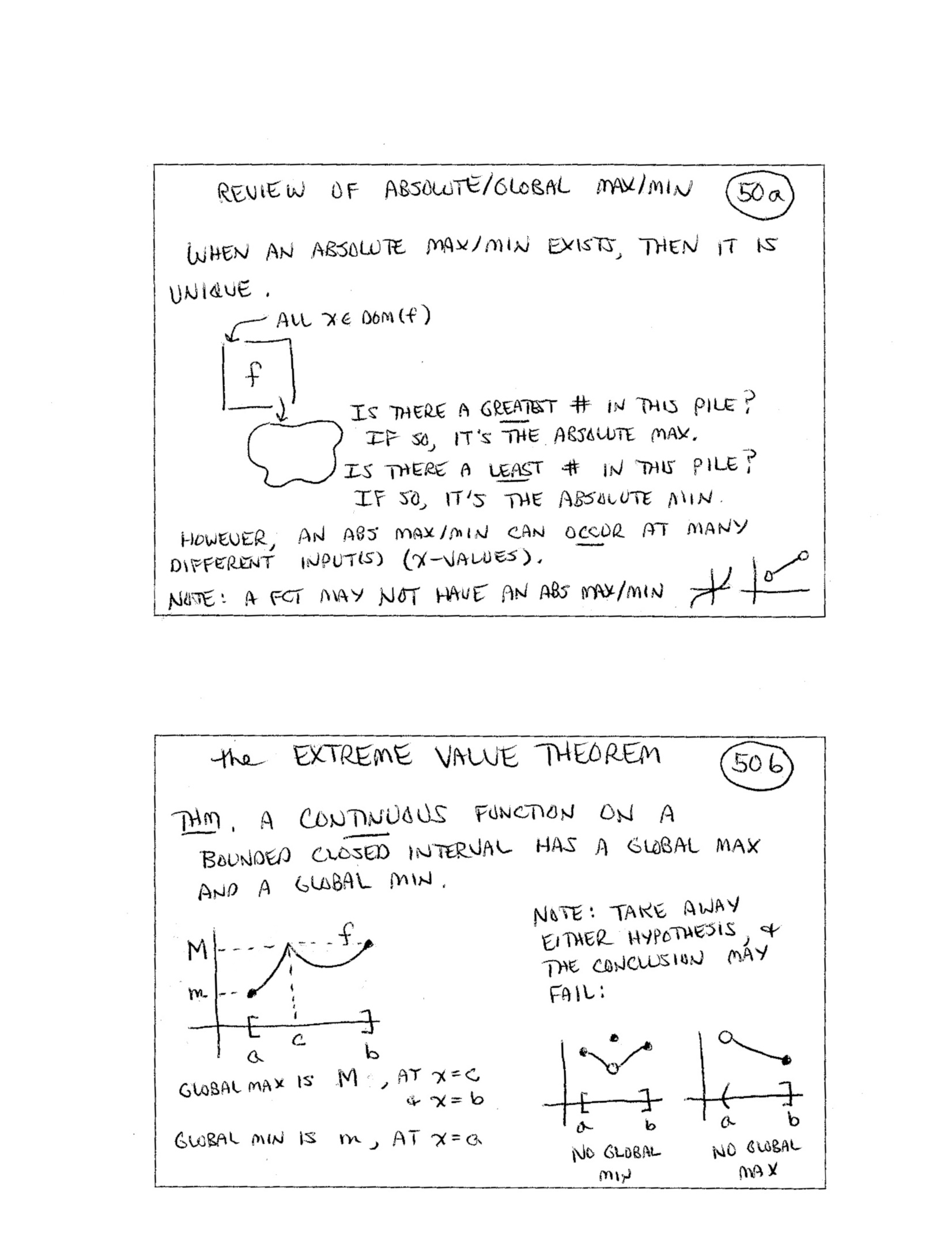51.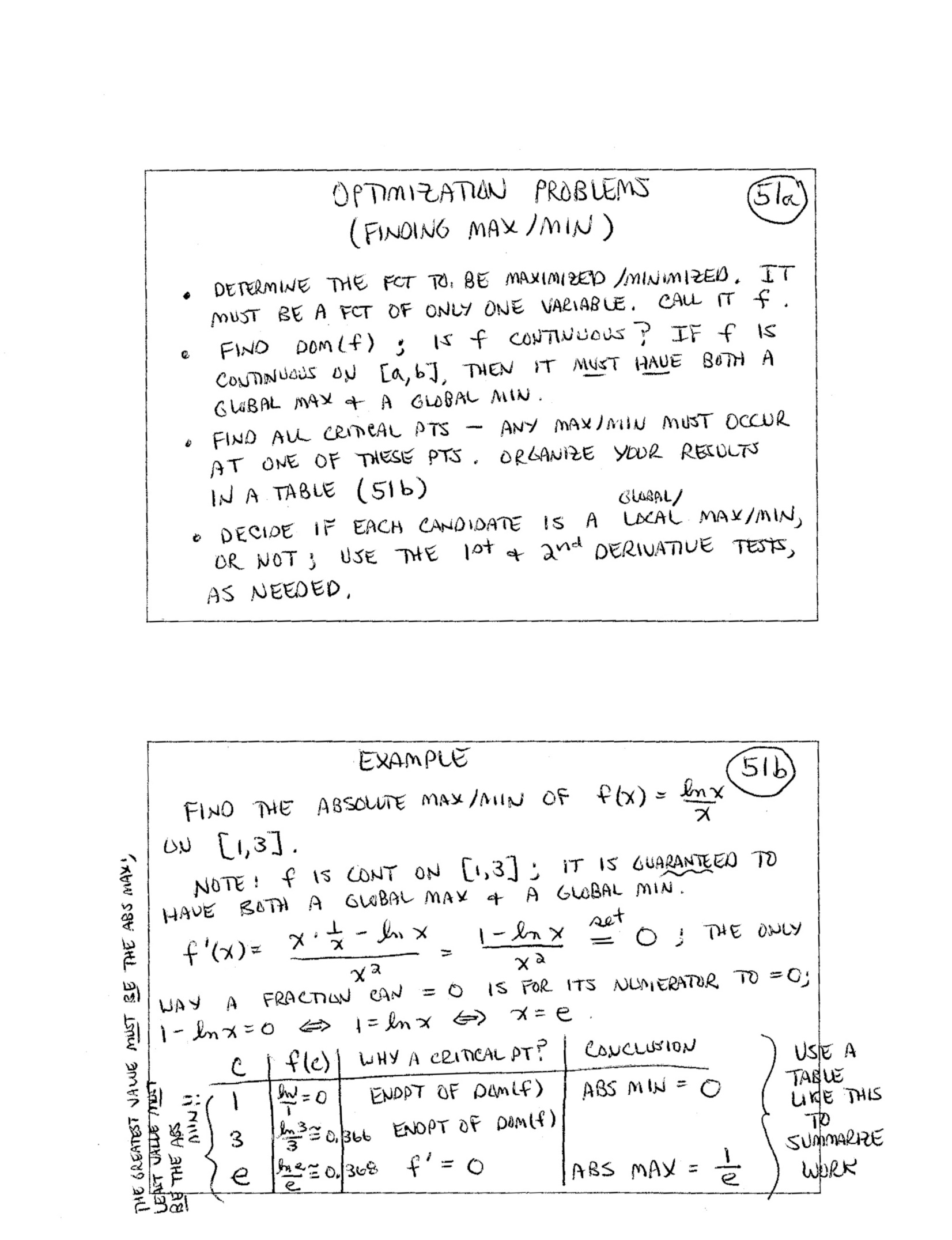52.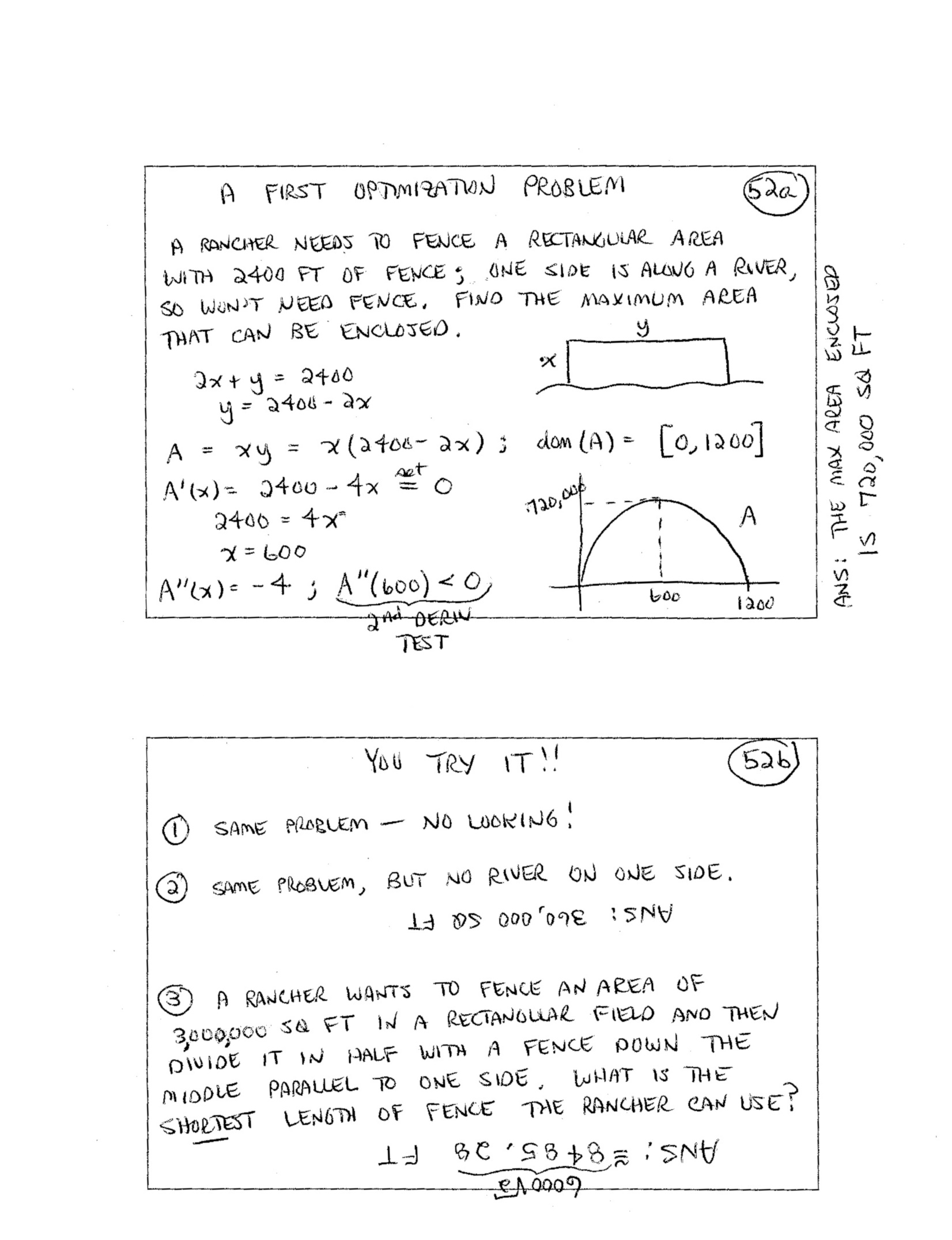53ab.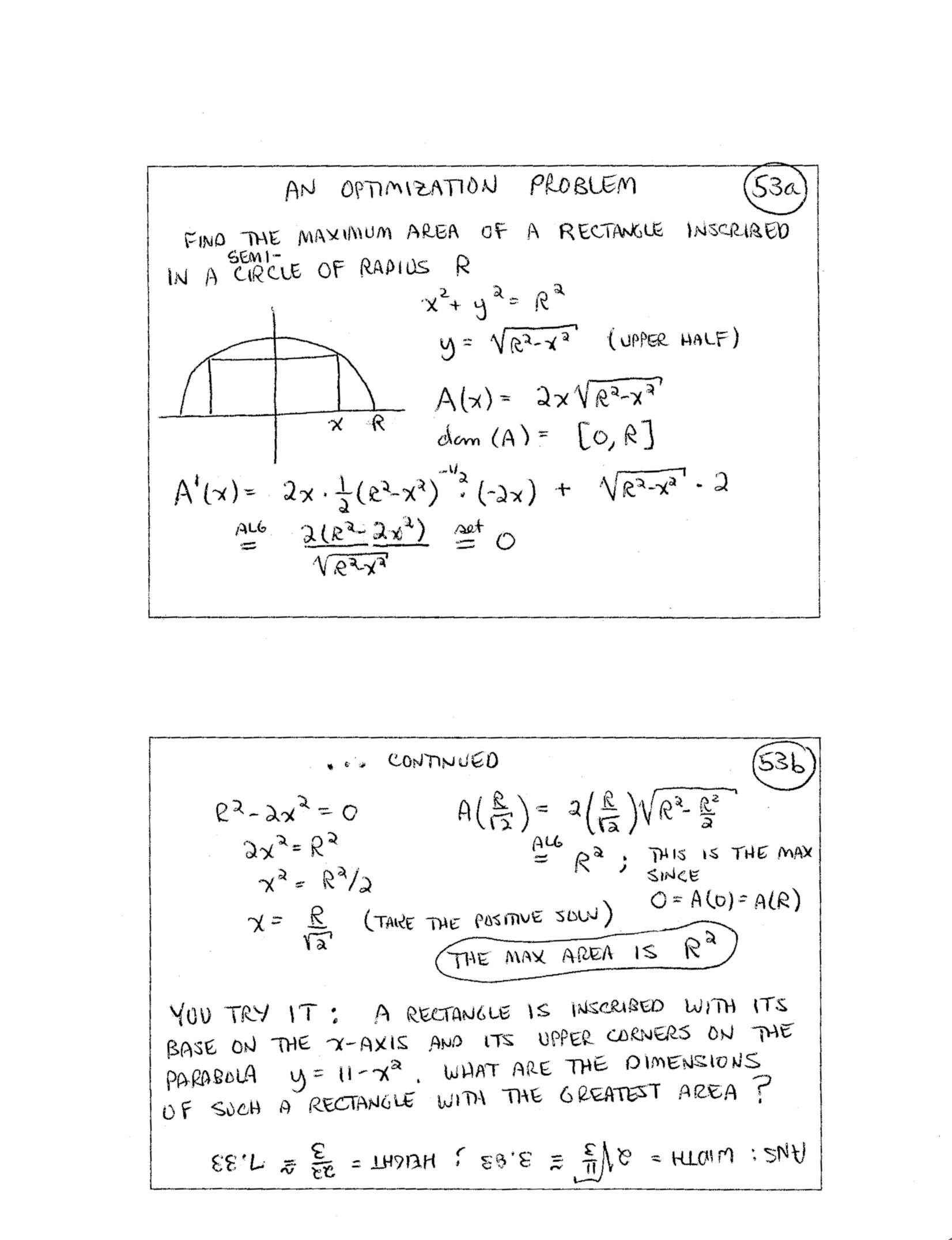53cd.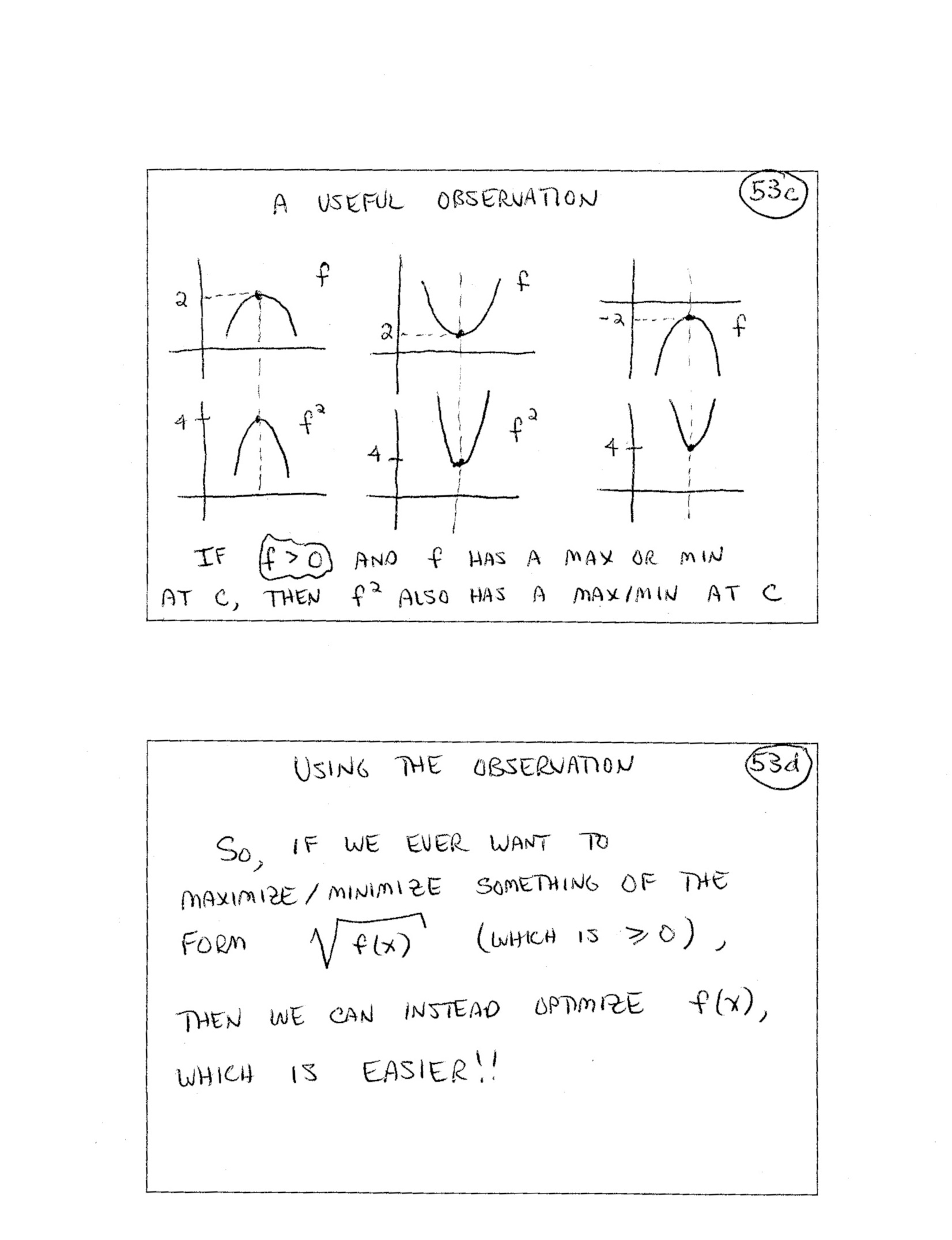54.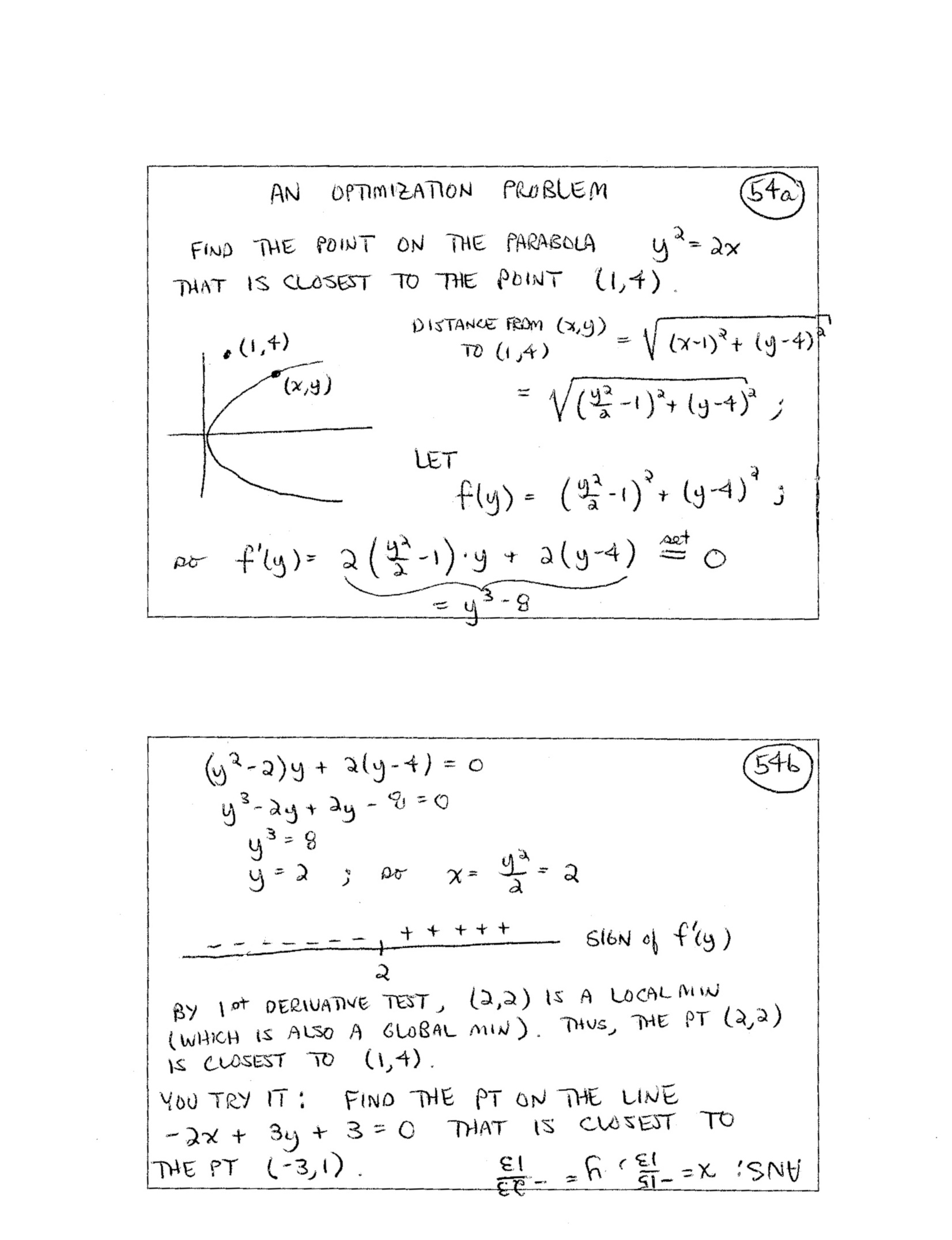55.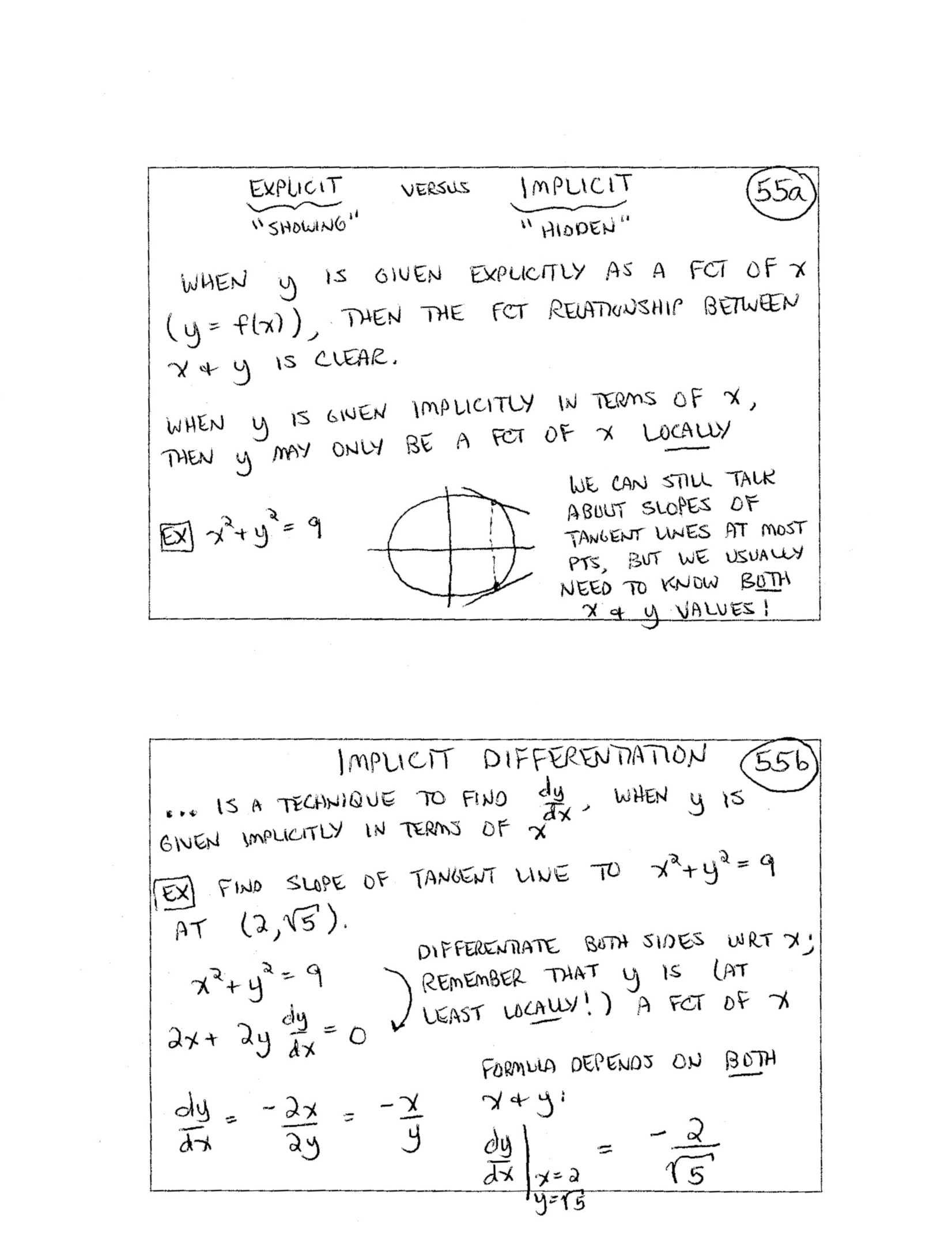56.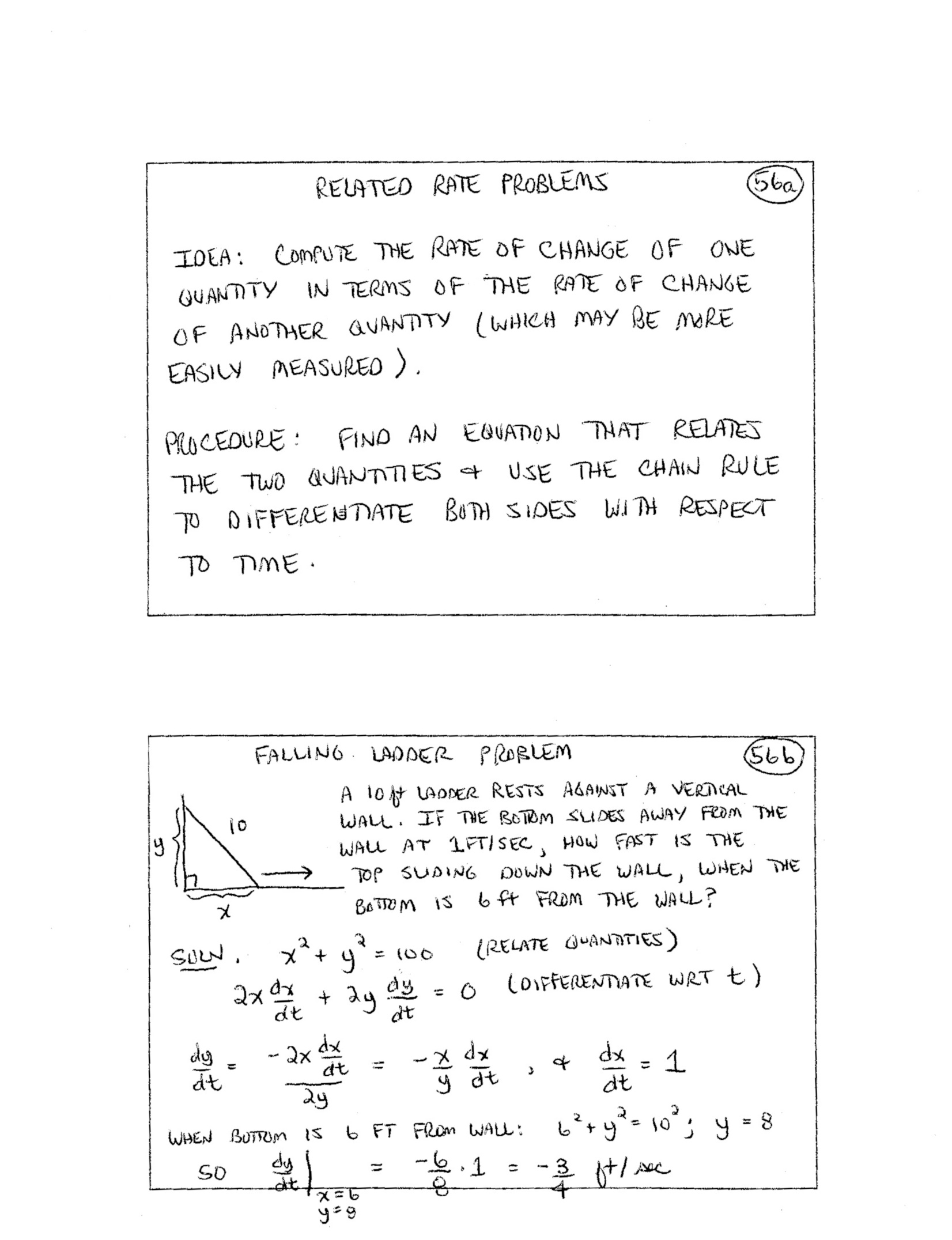(included forhigher-level course) 57.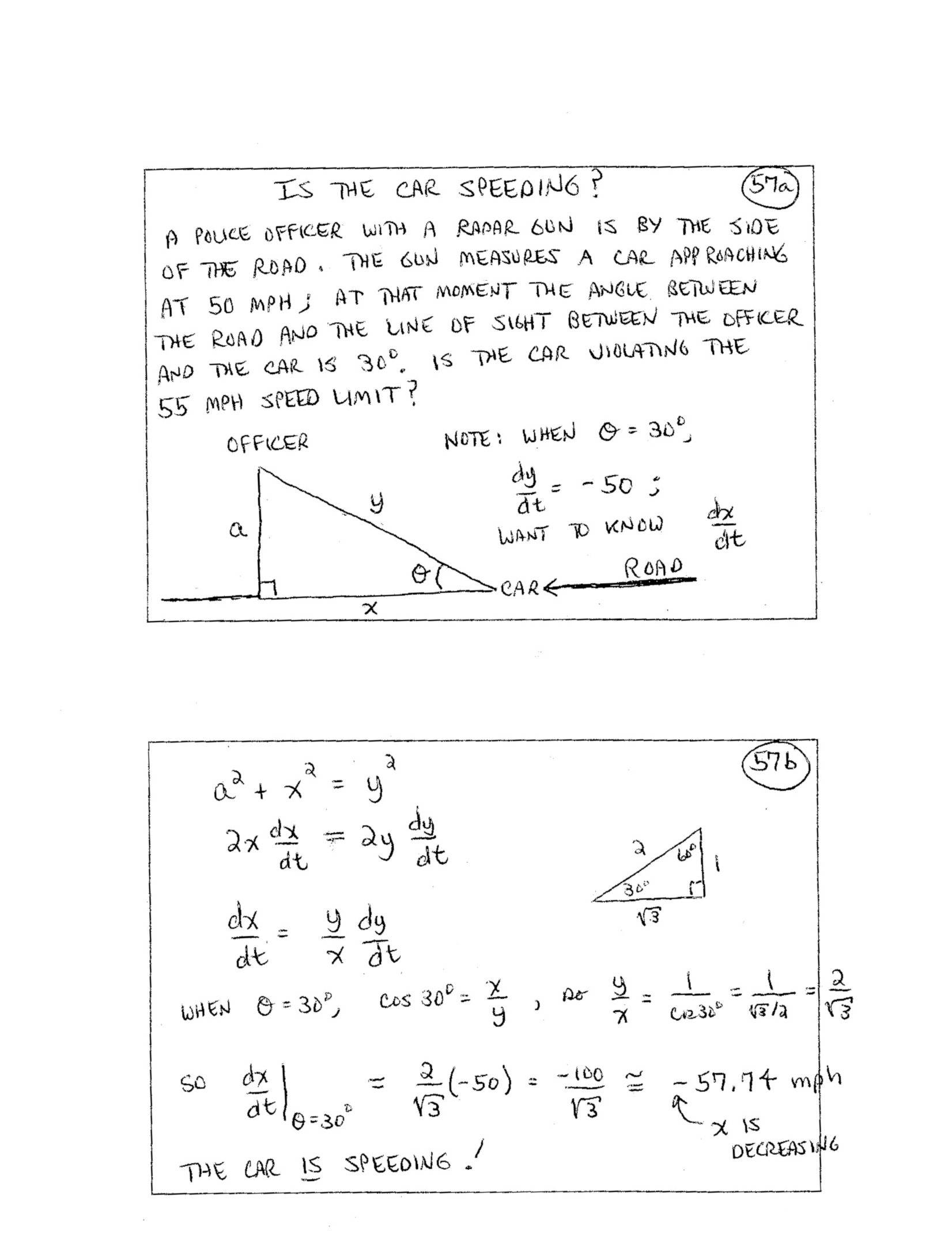(included forhigher-level course) 58.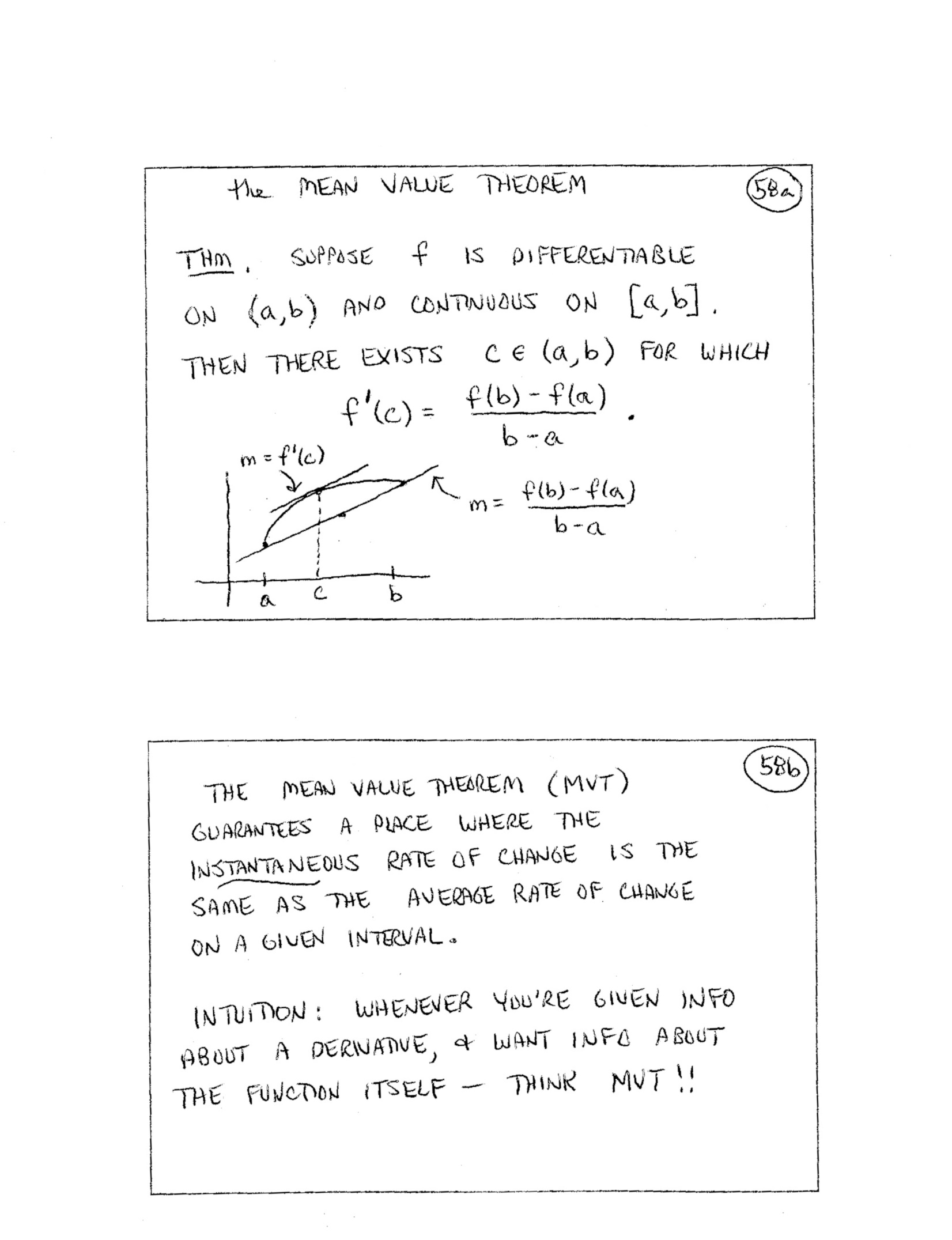59.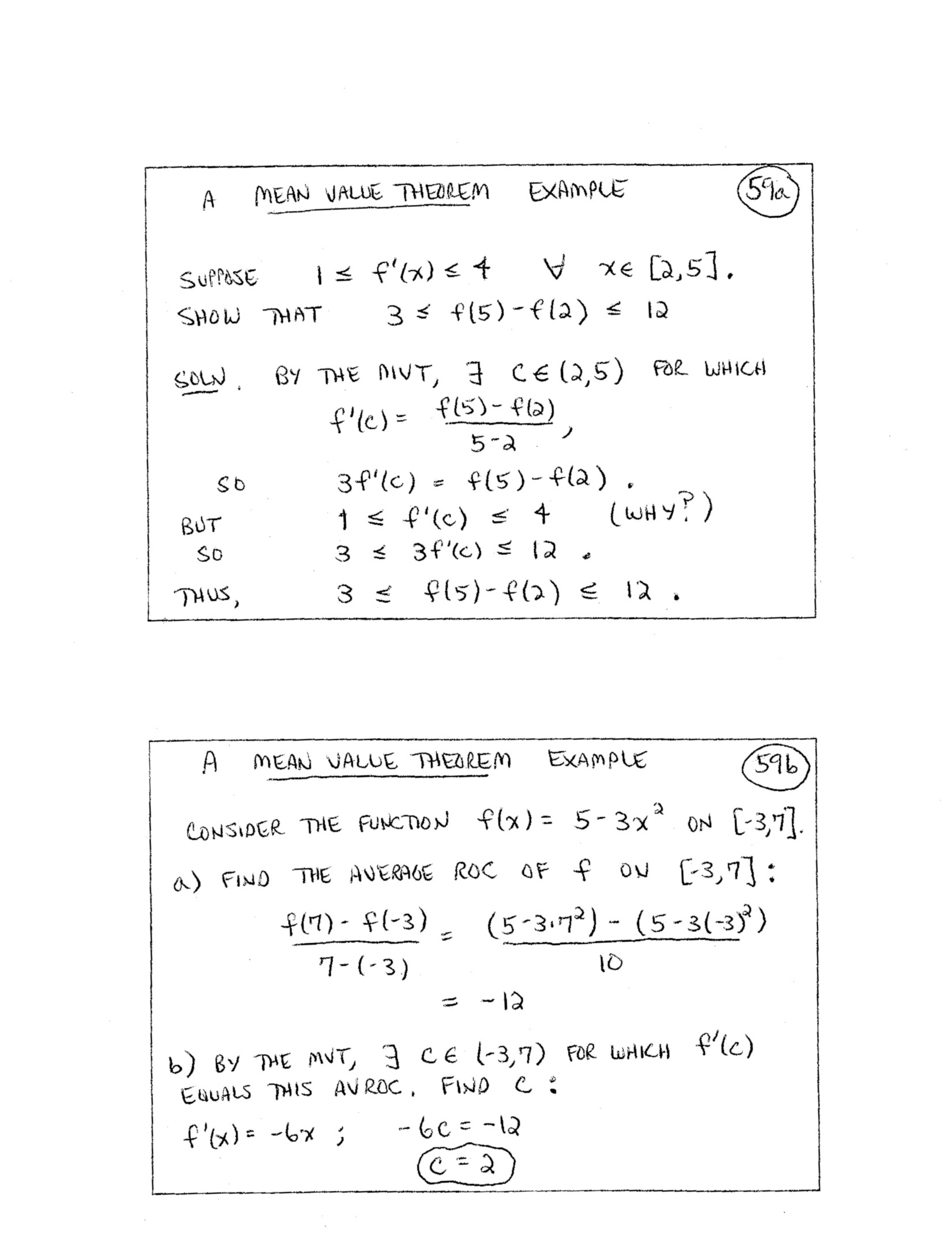60.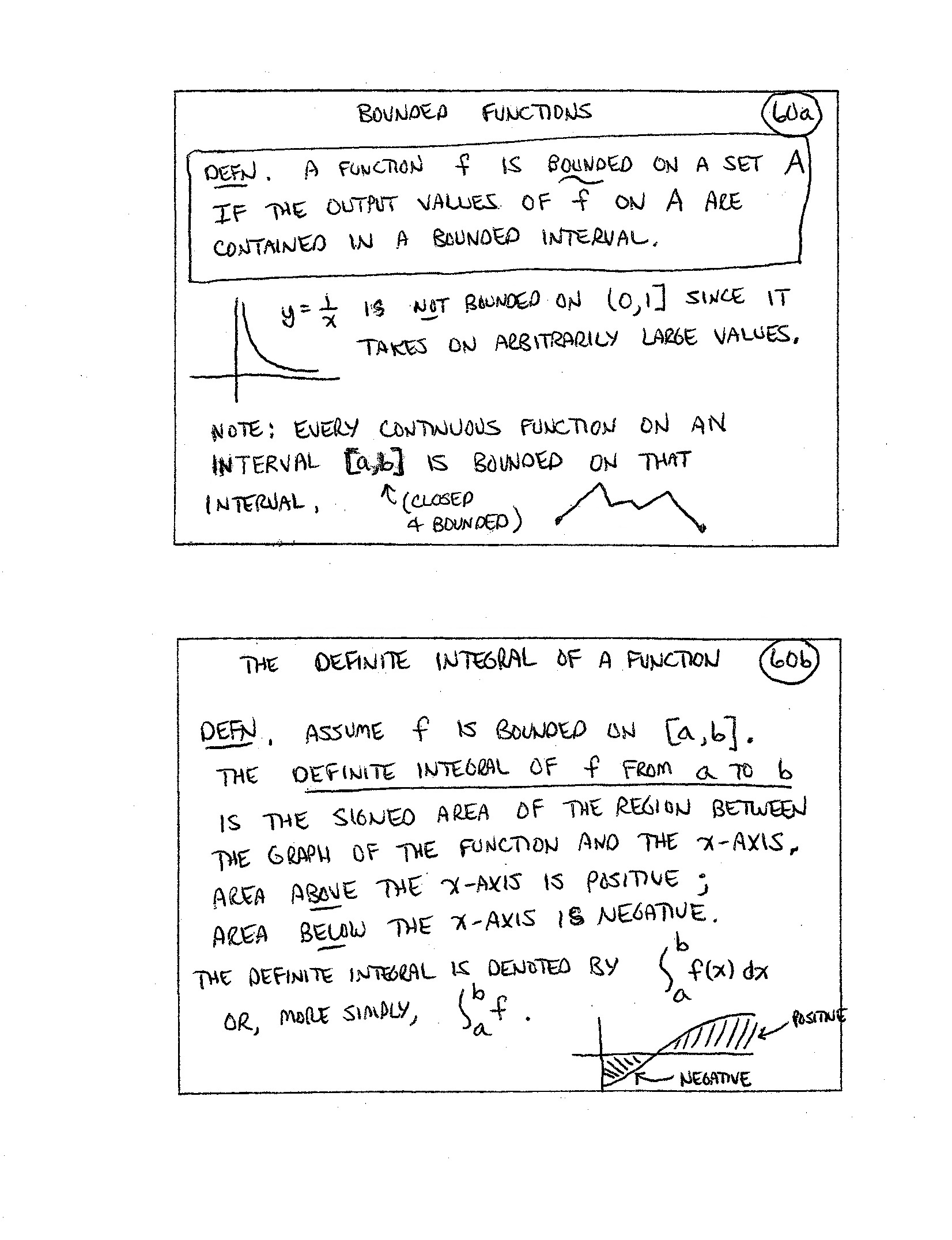61.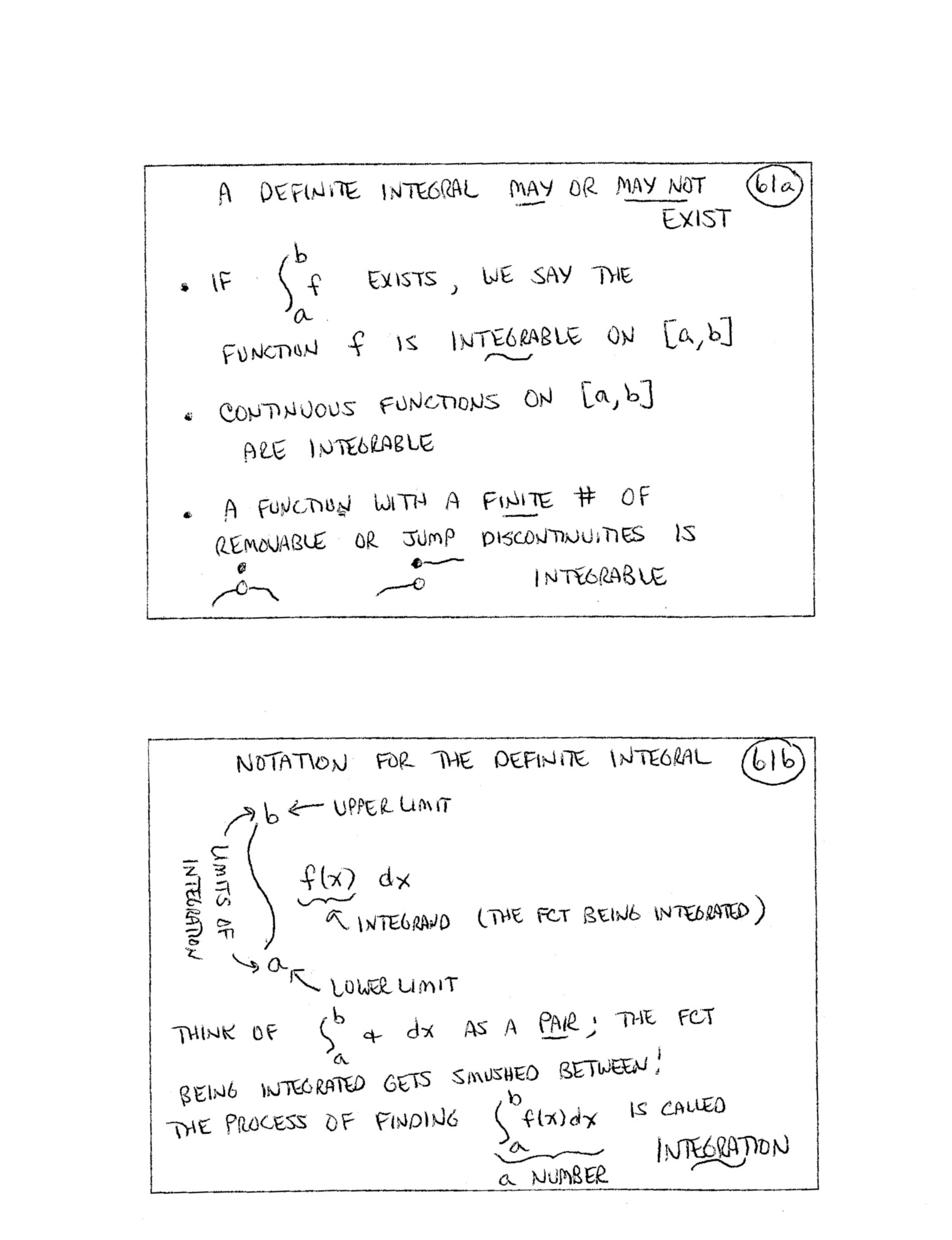62.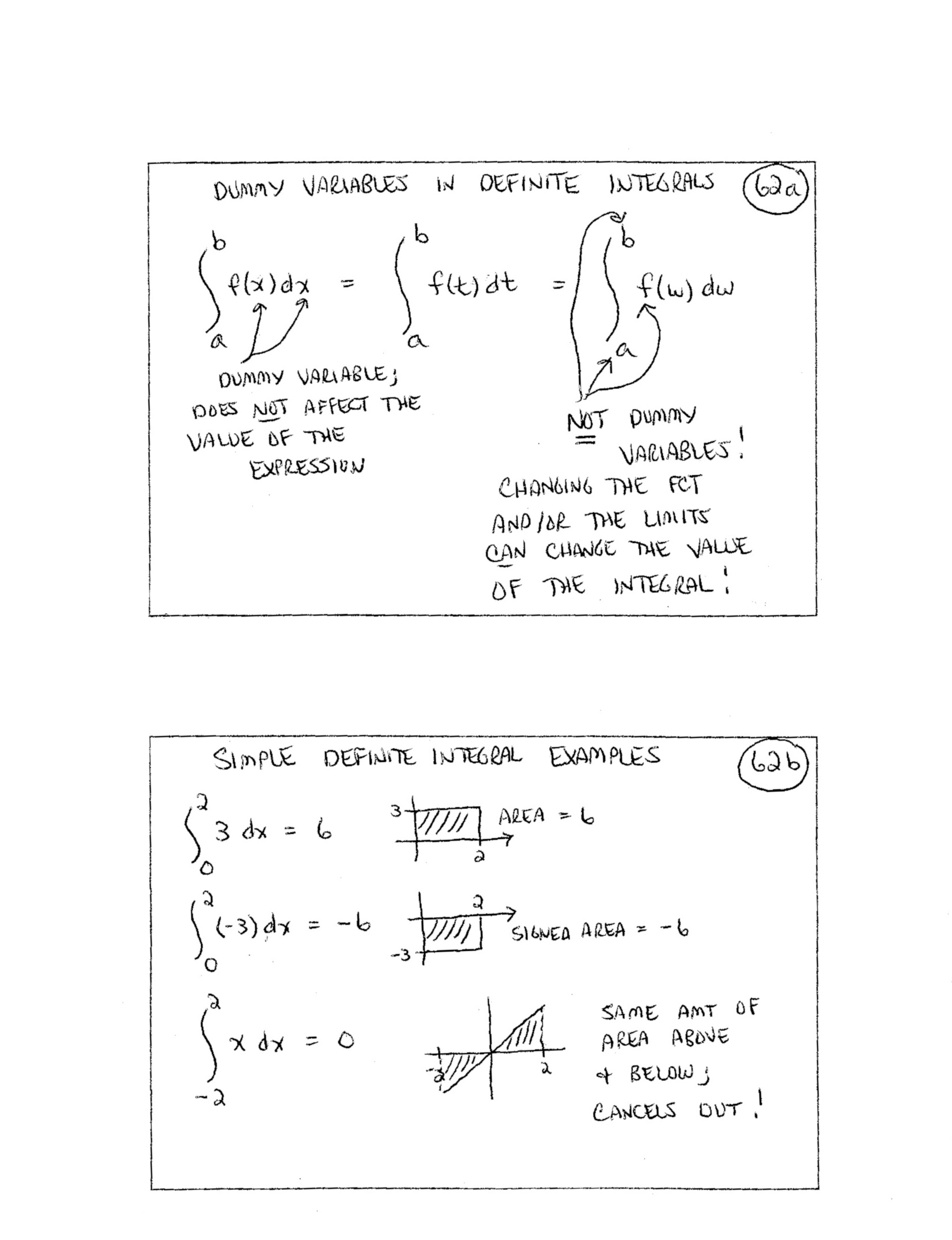63.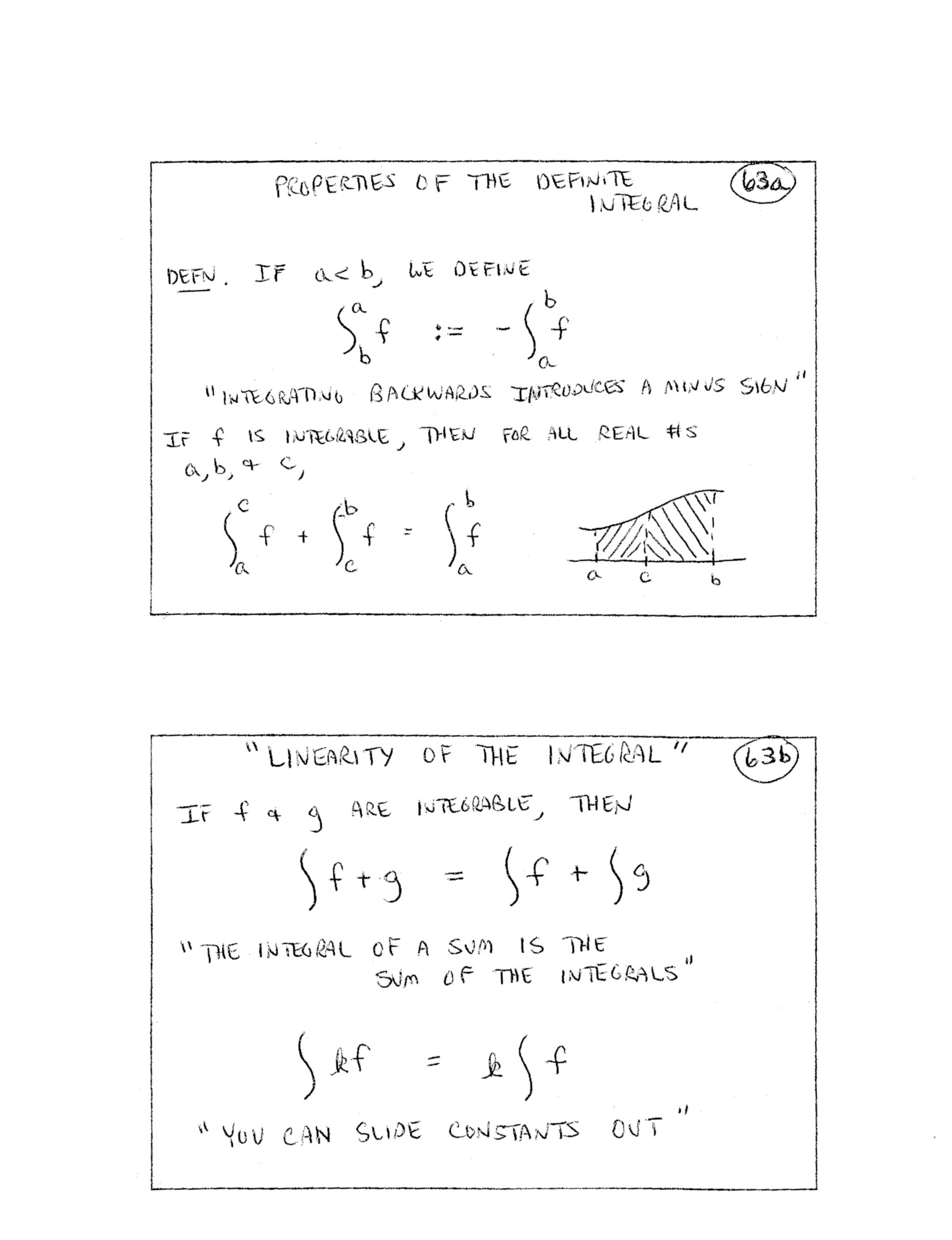64.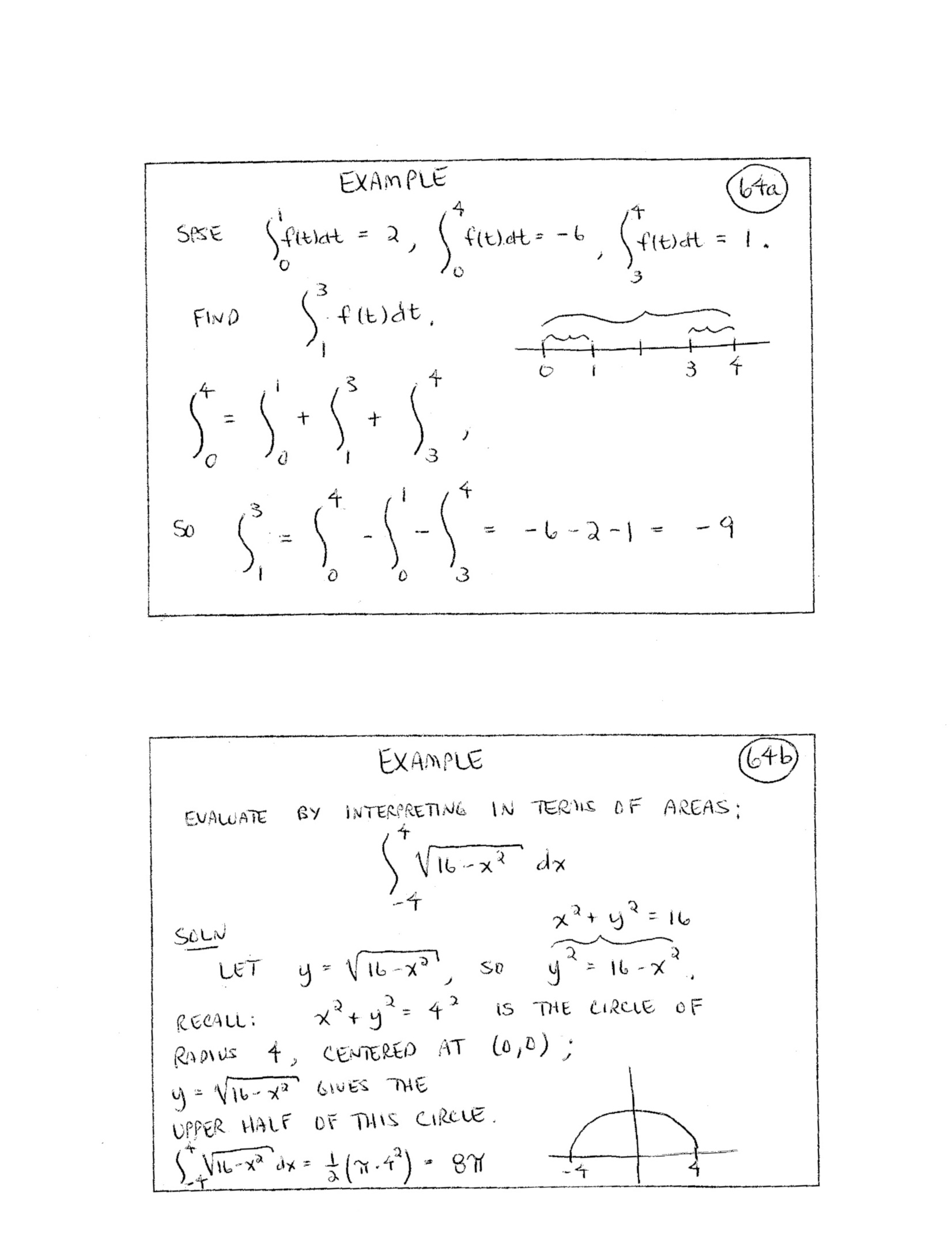65.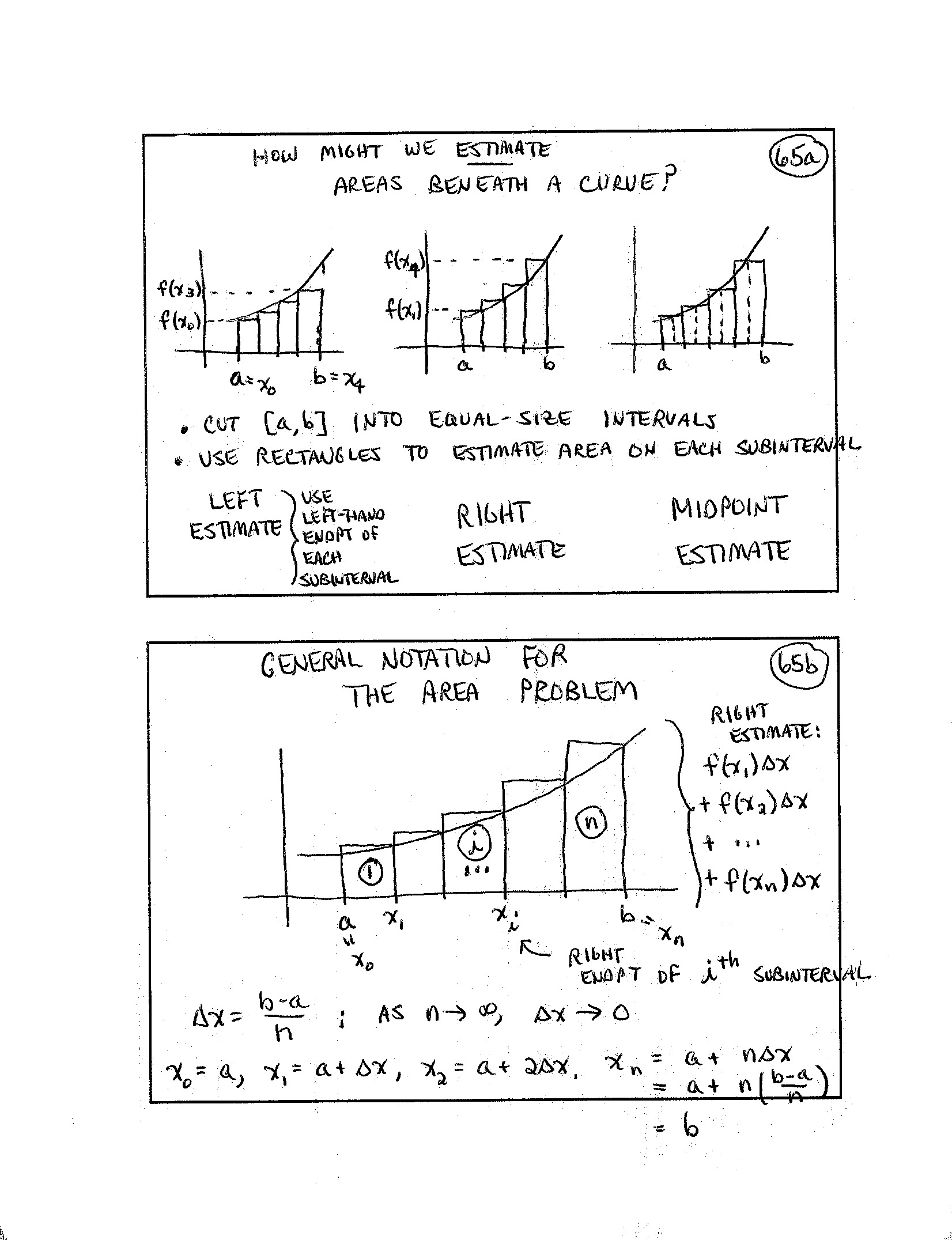66.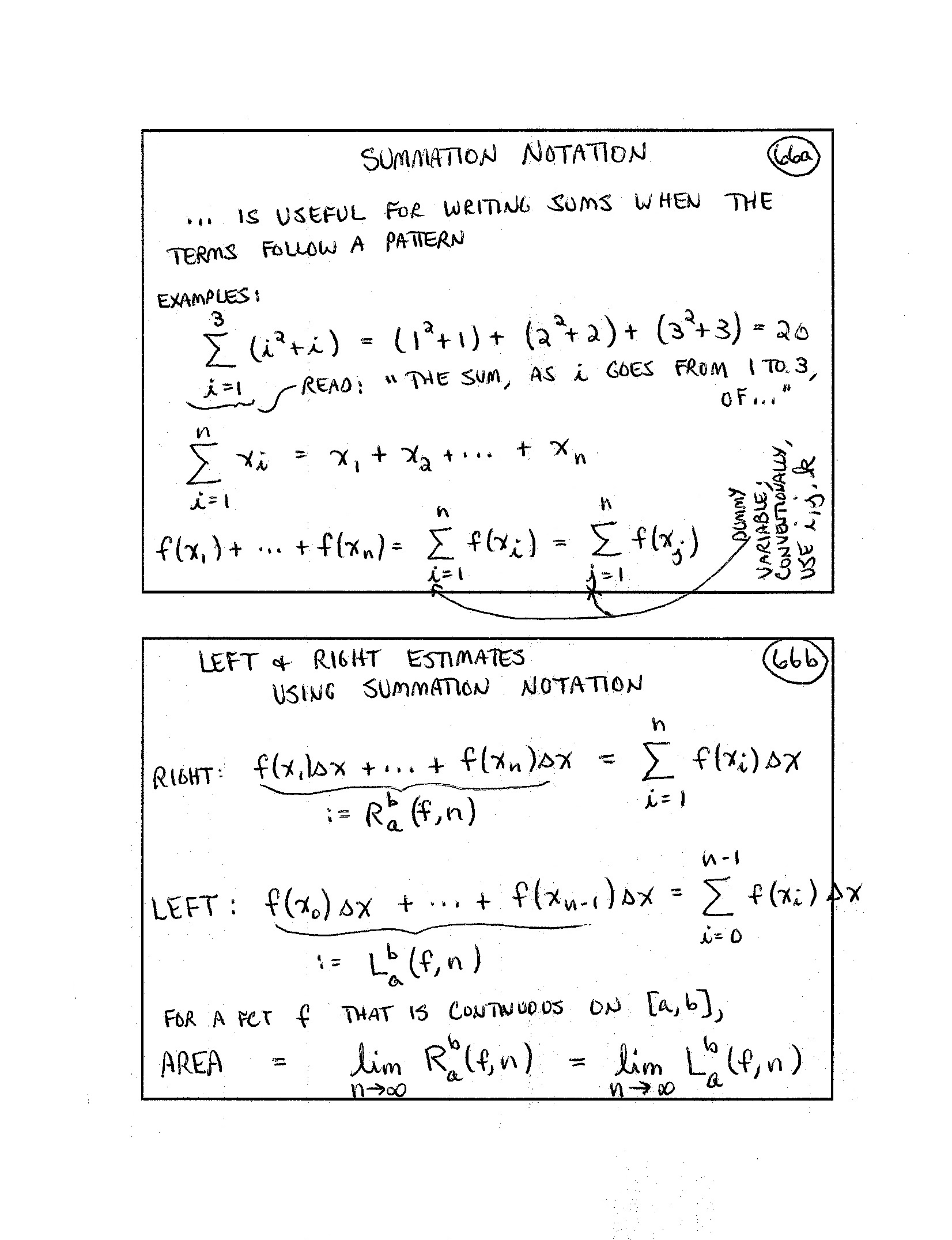67.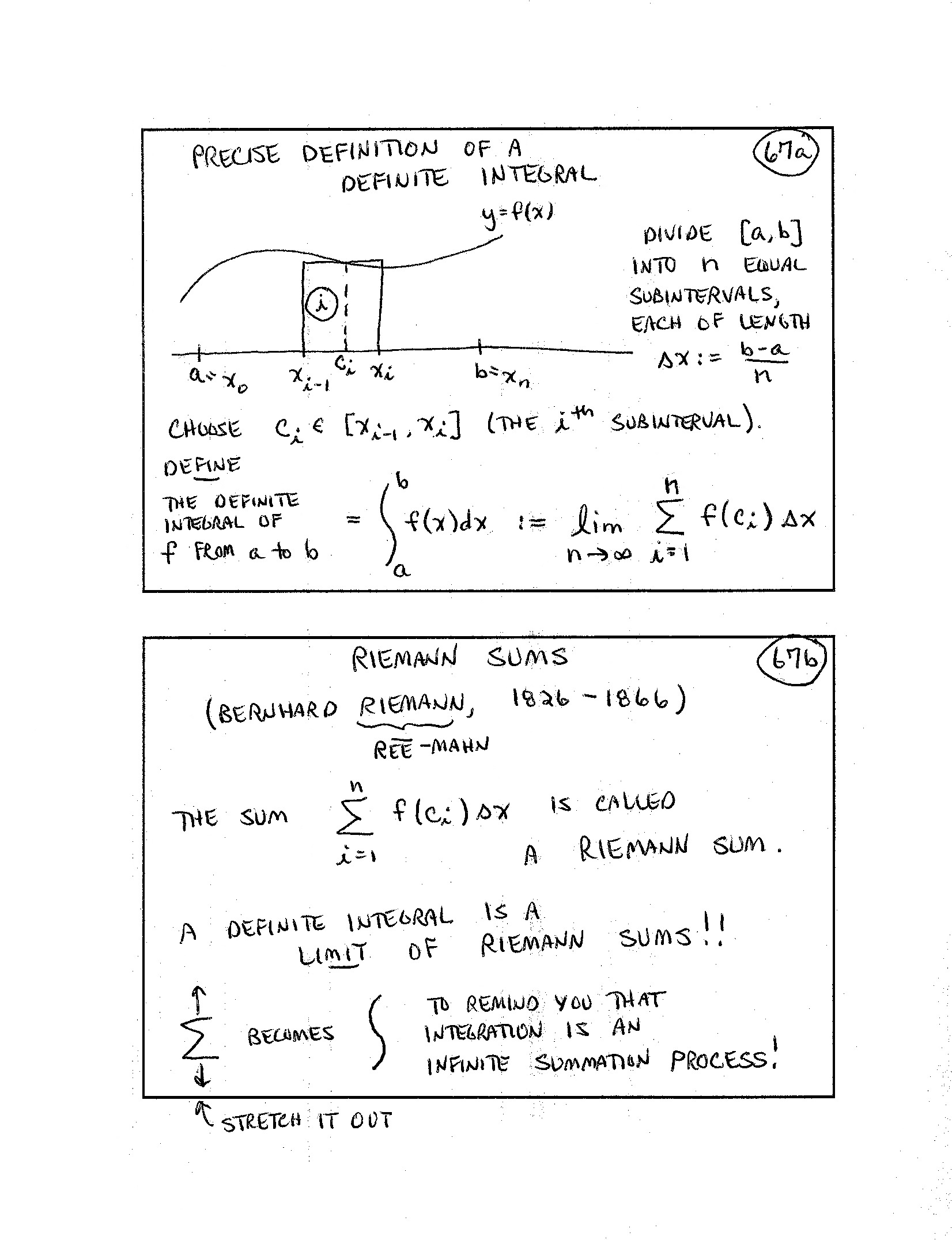68.69.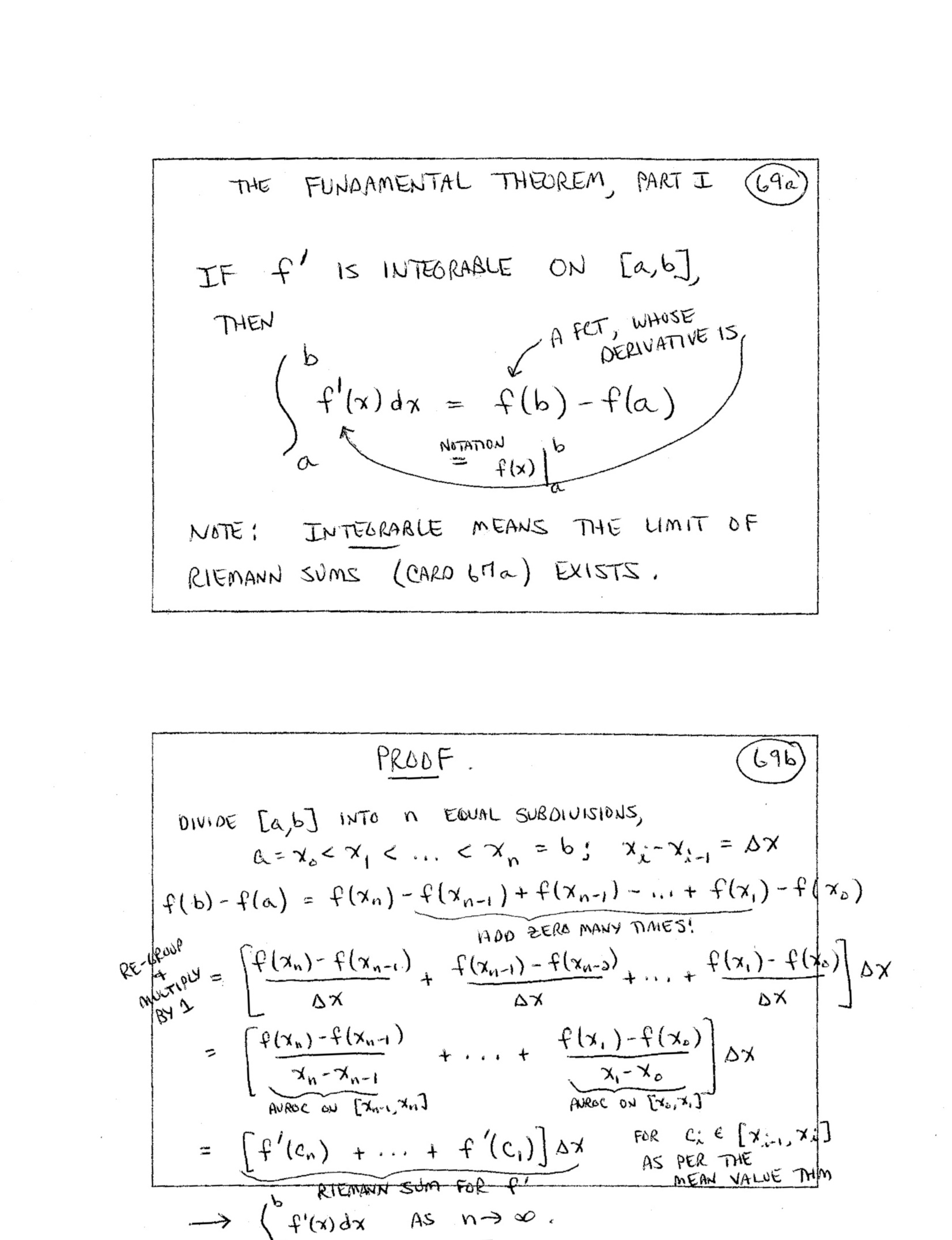(included forhigher-level course) 70.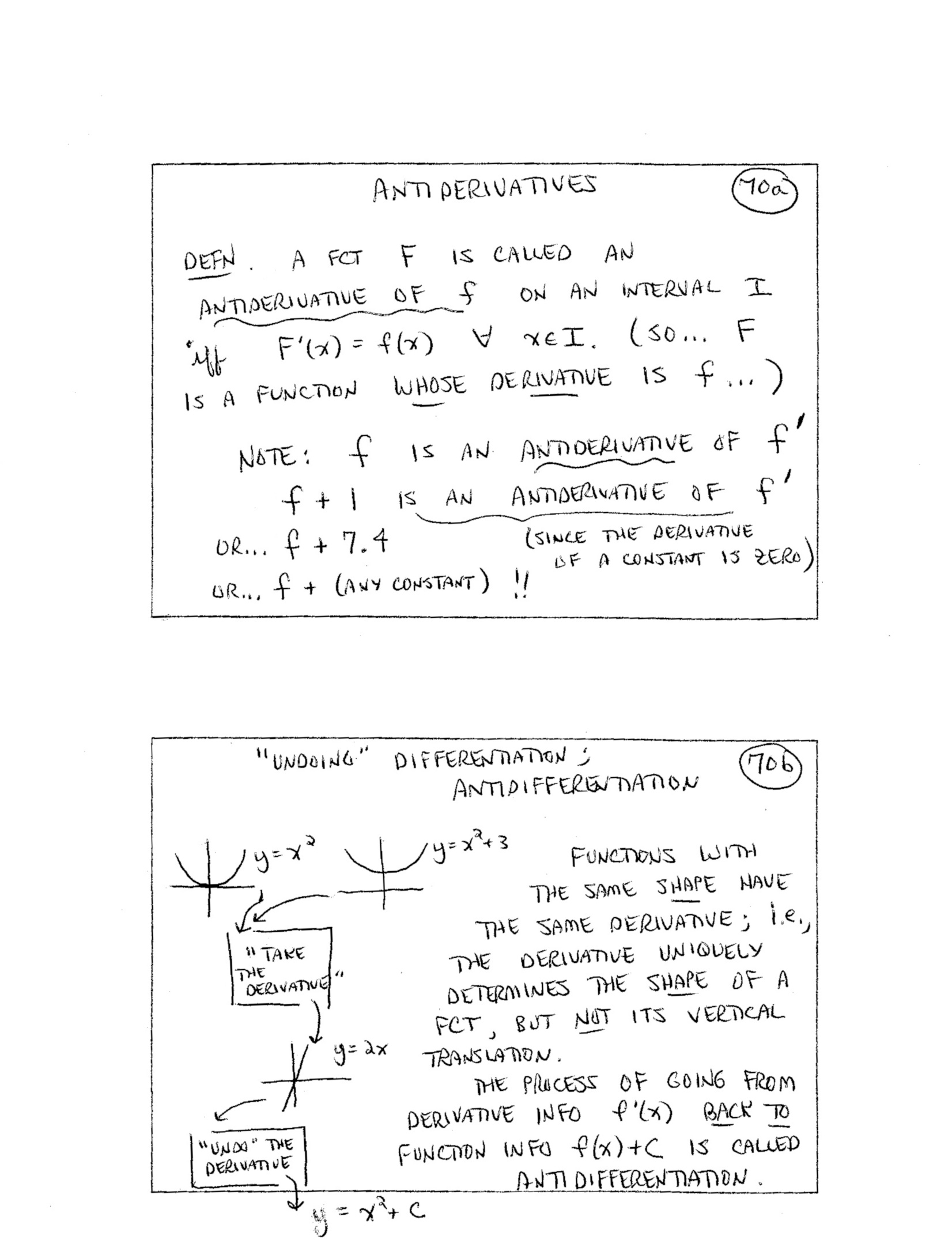71.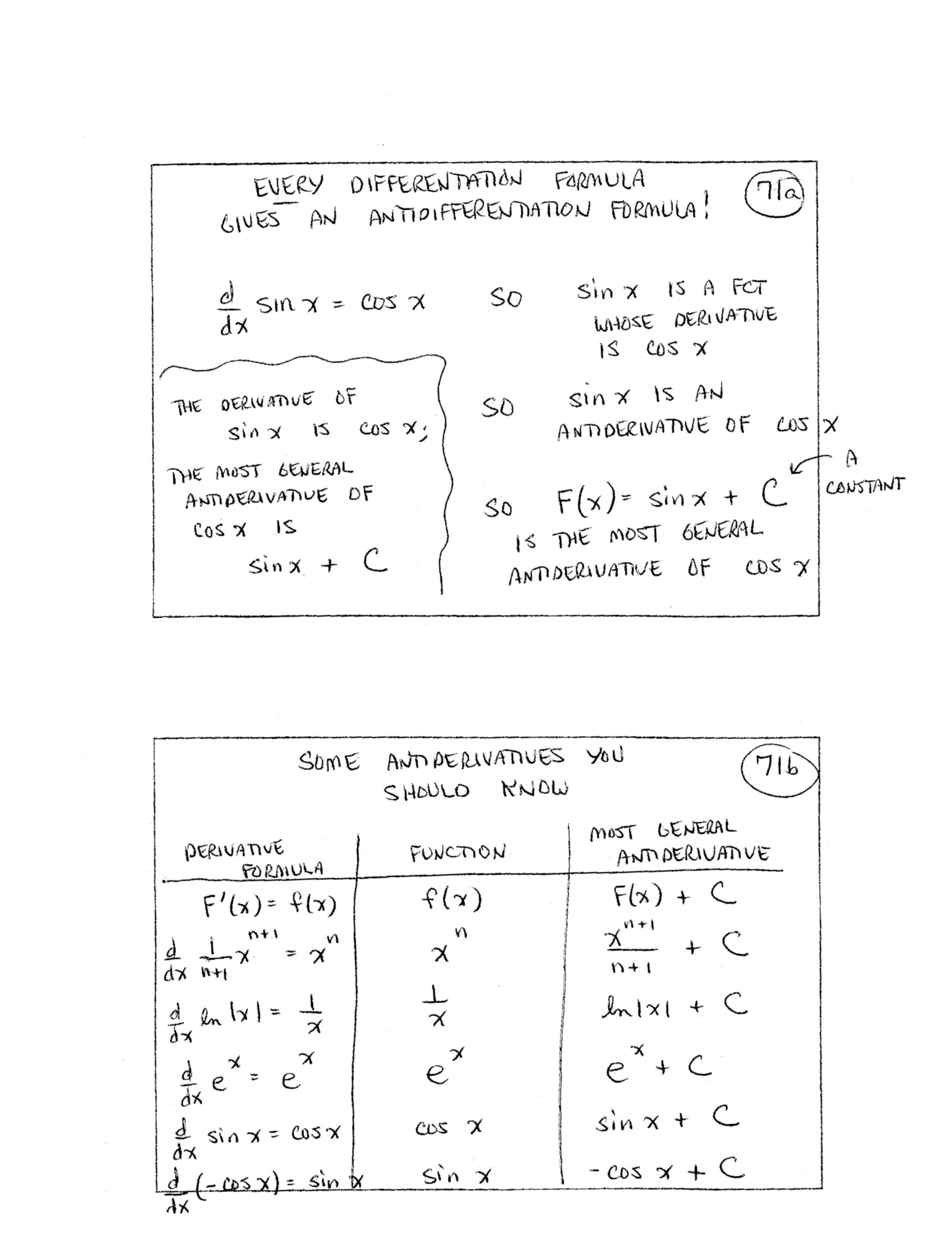72.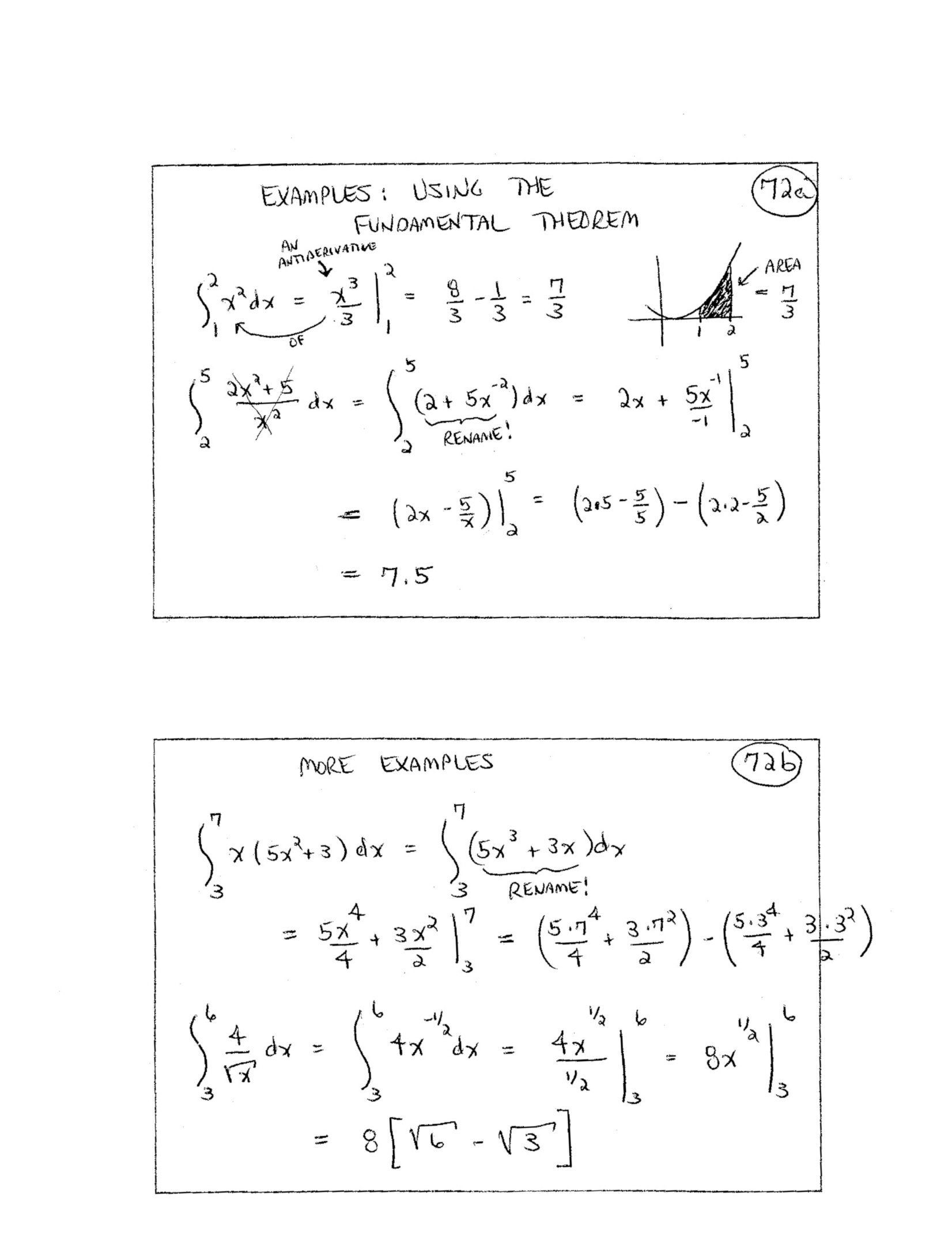73.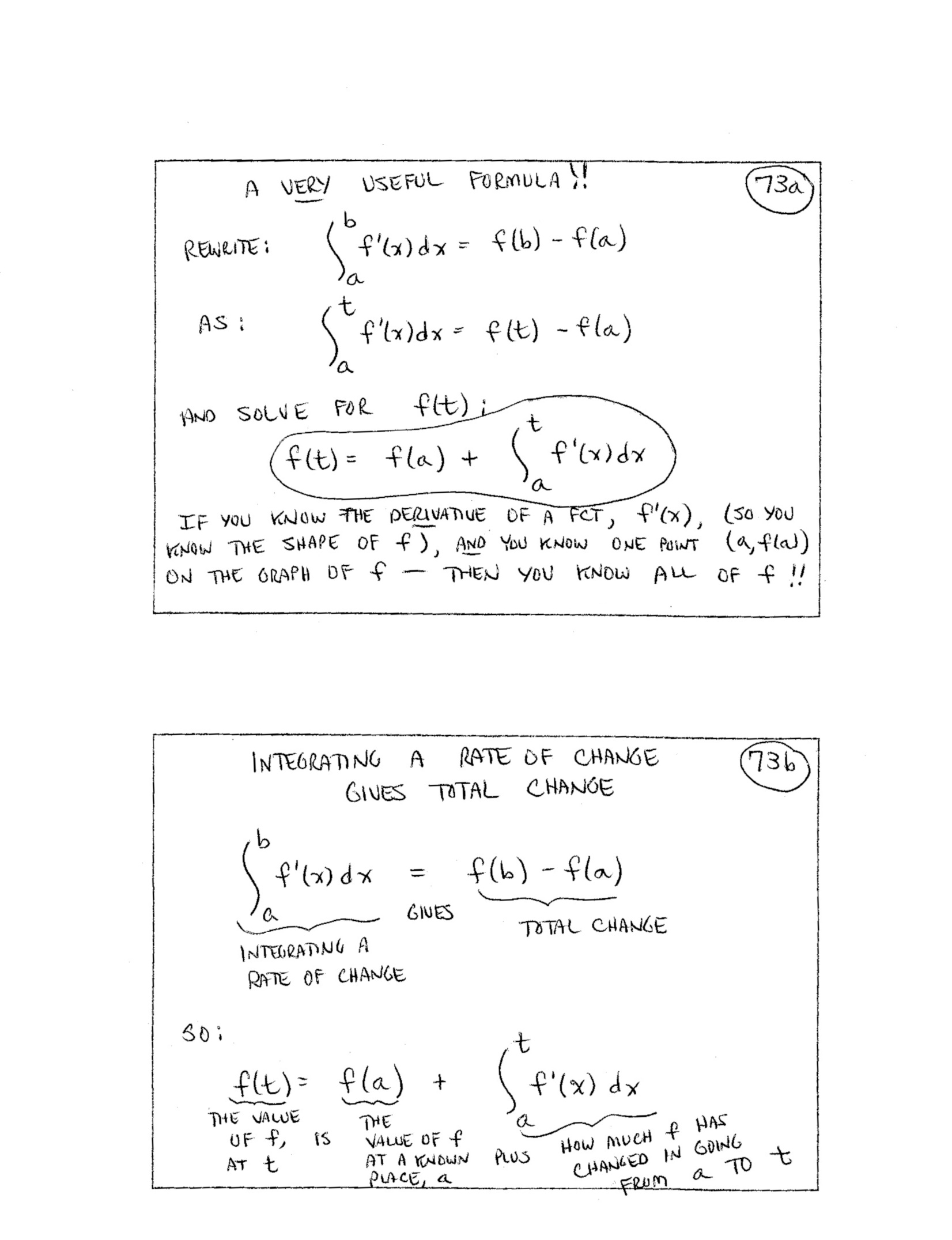74.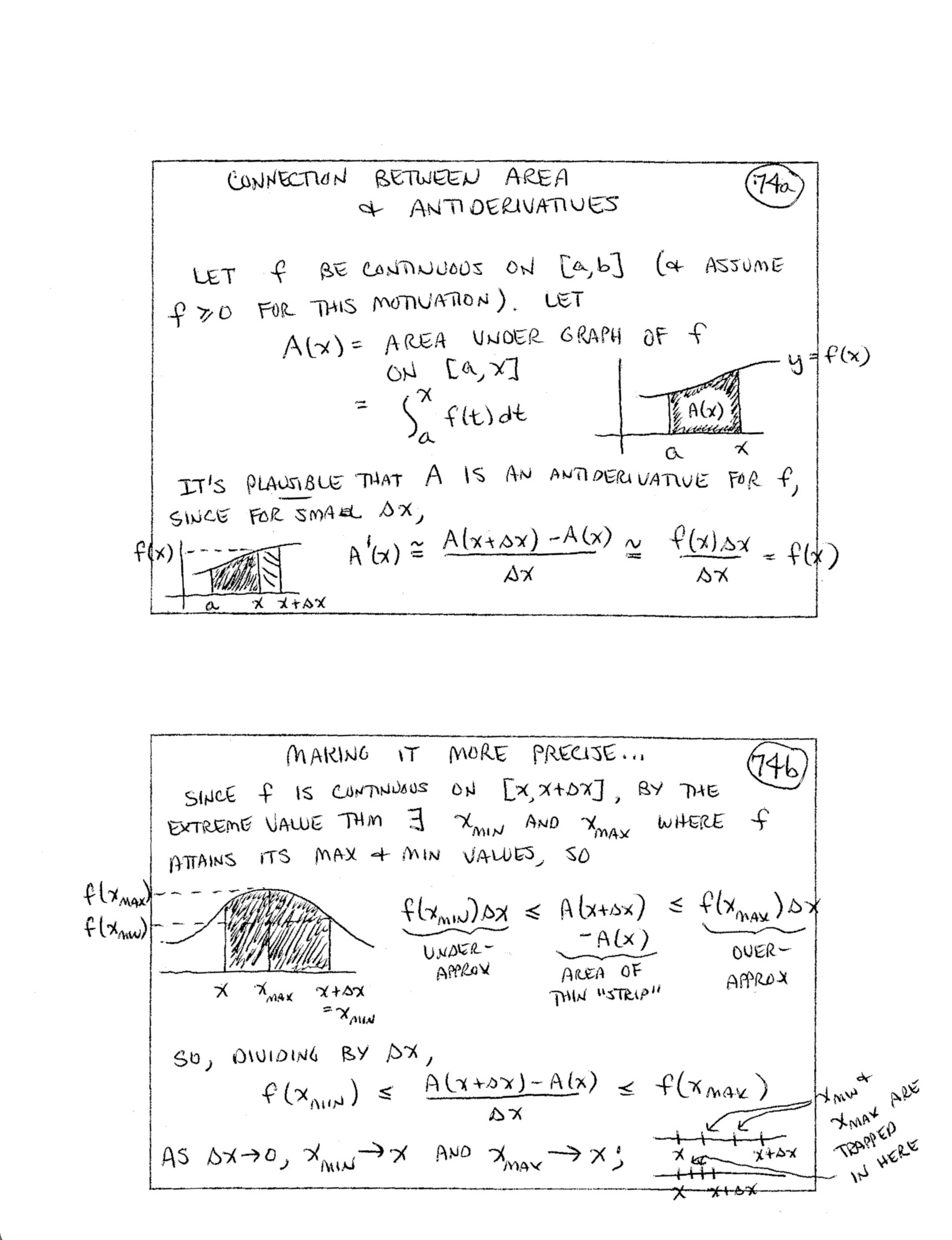75.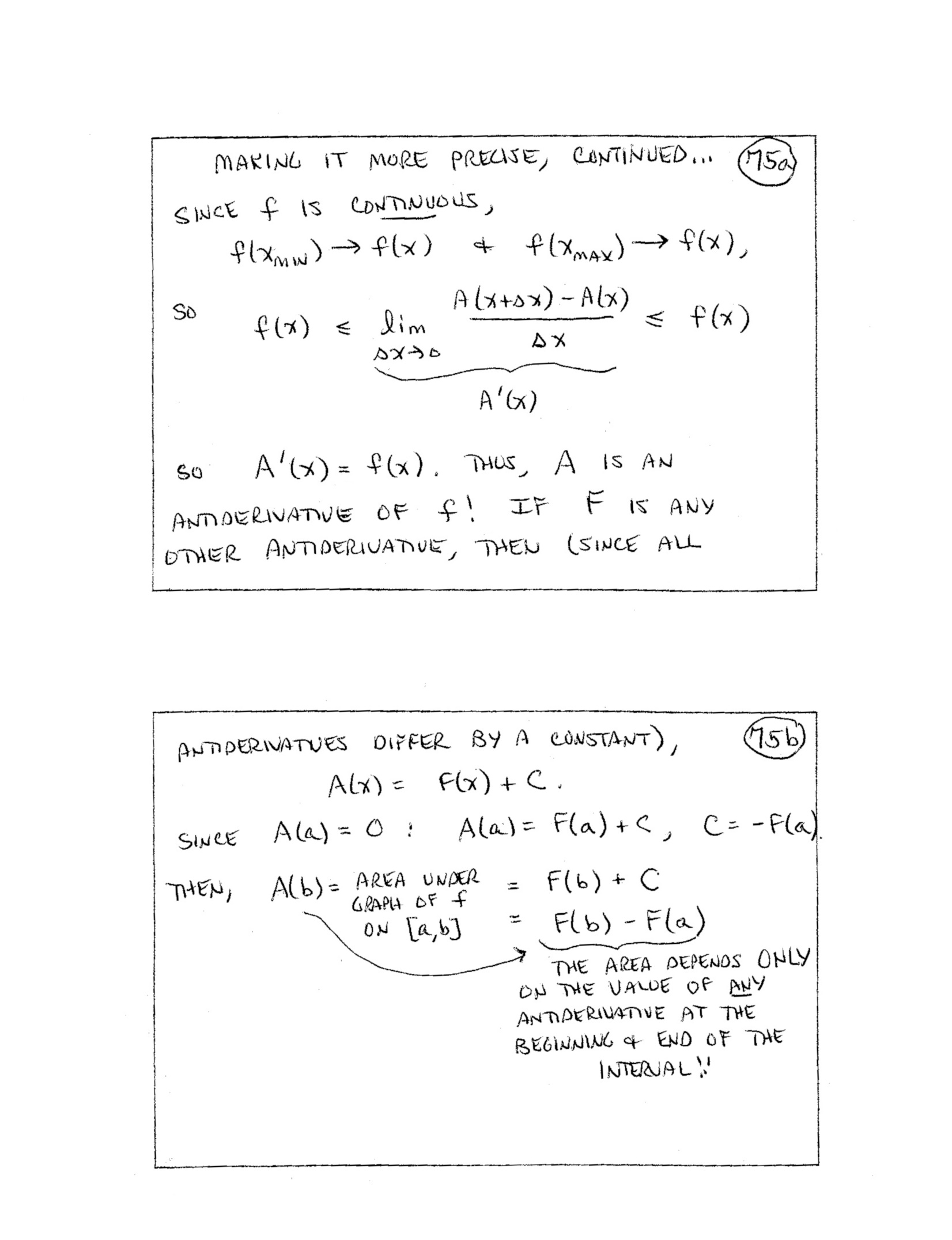76.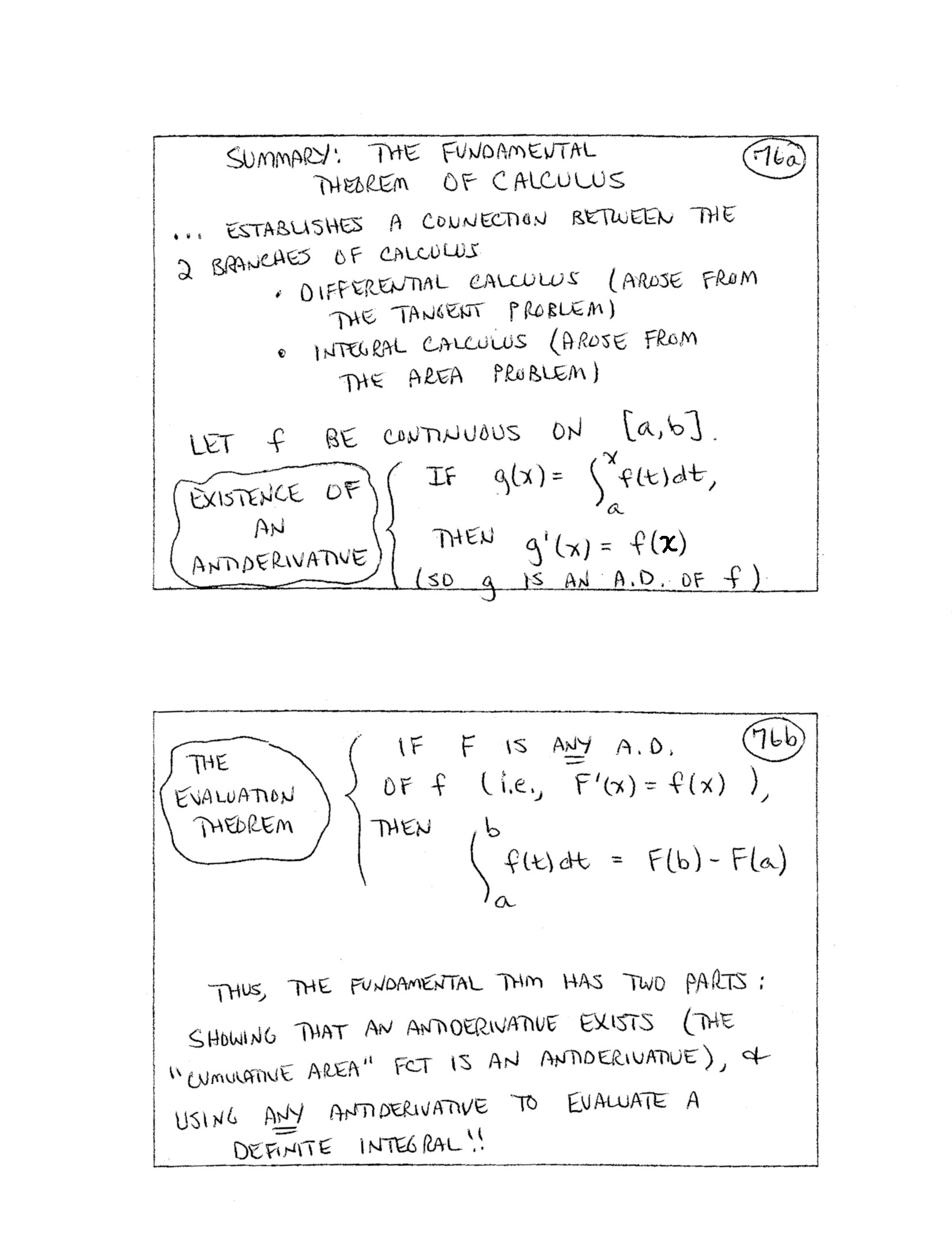77.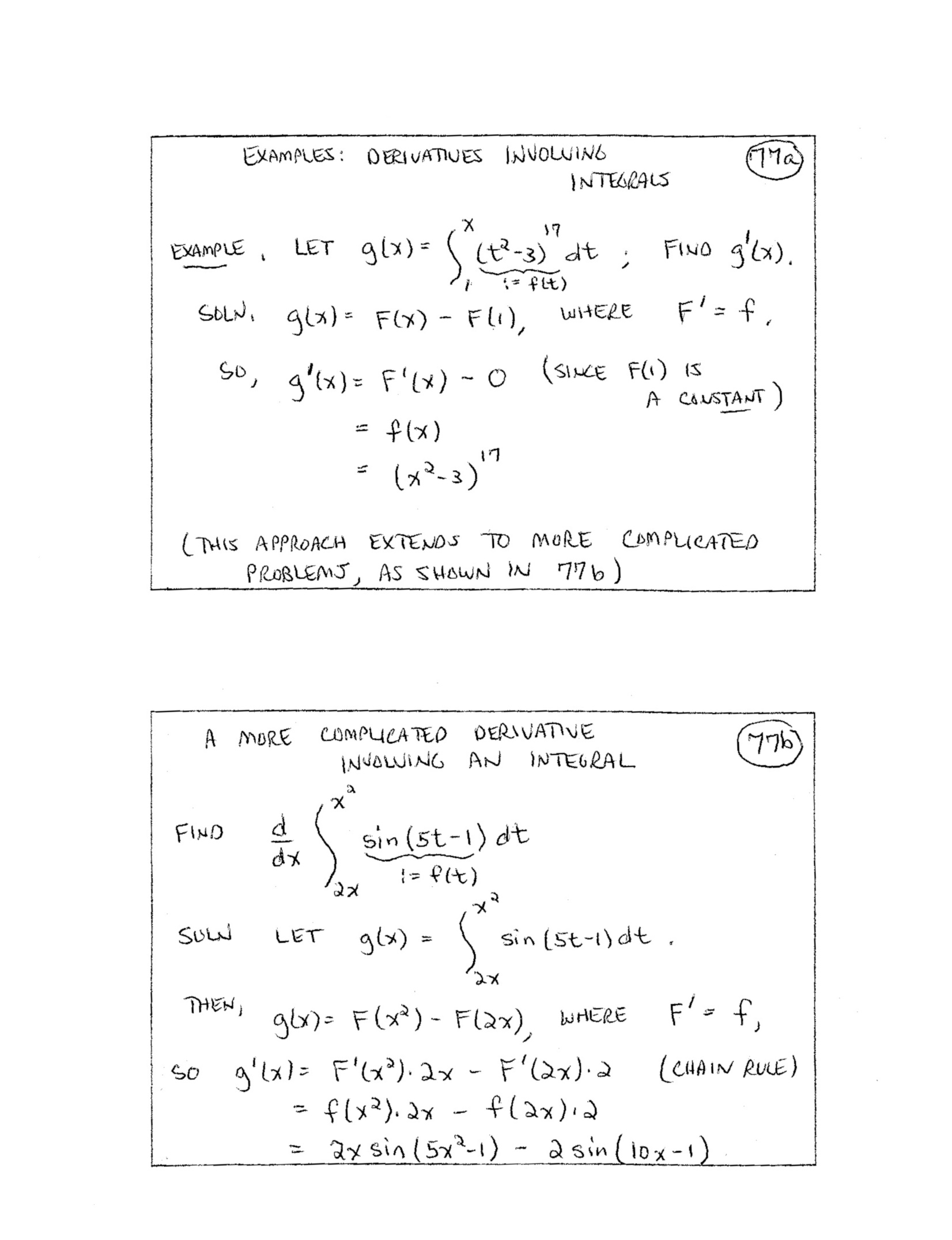(included forhigher-level course) 78.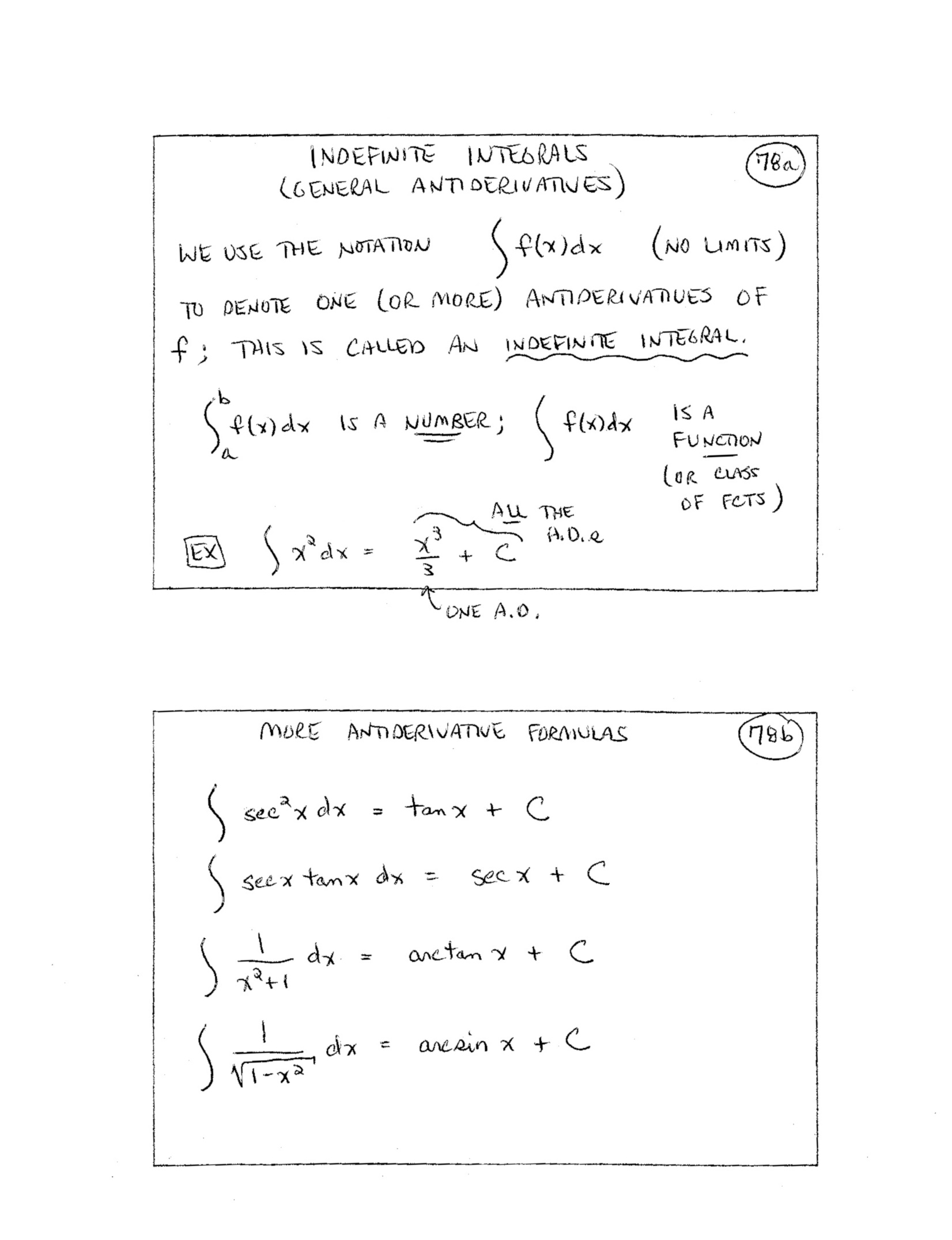79.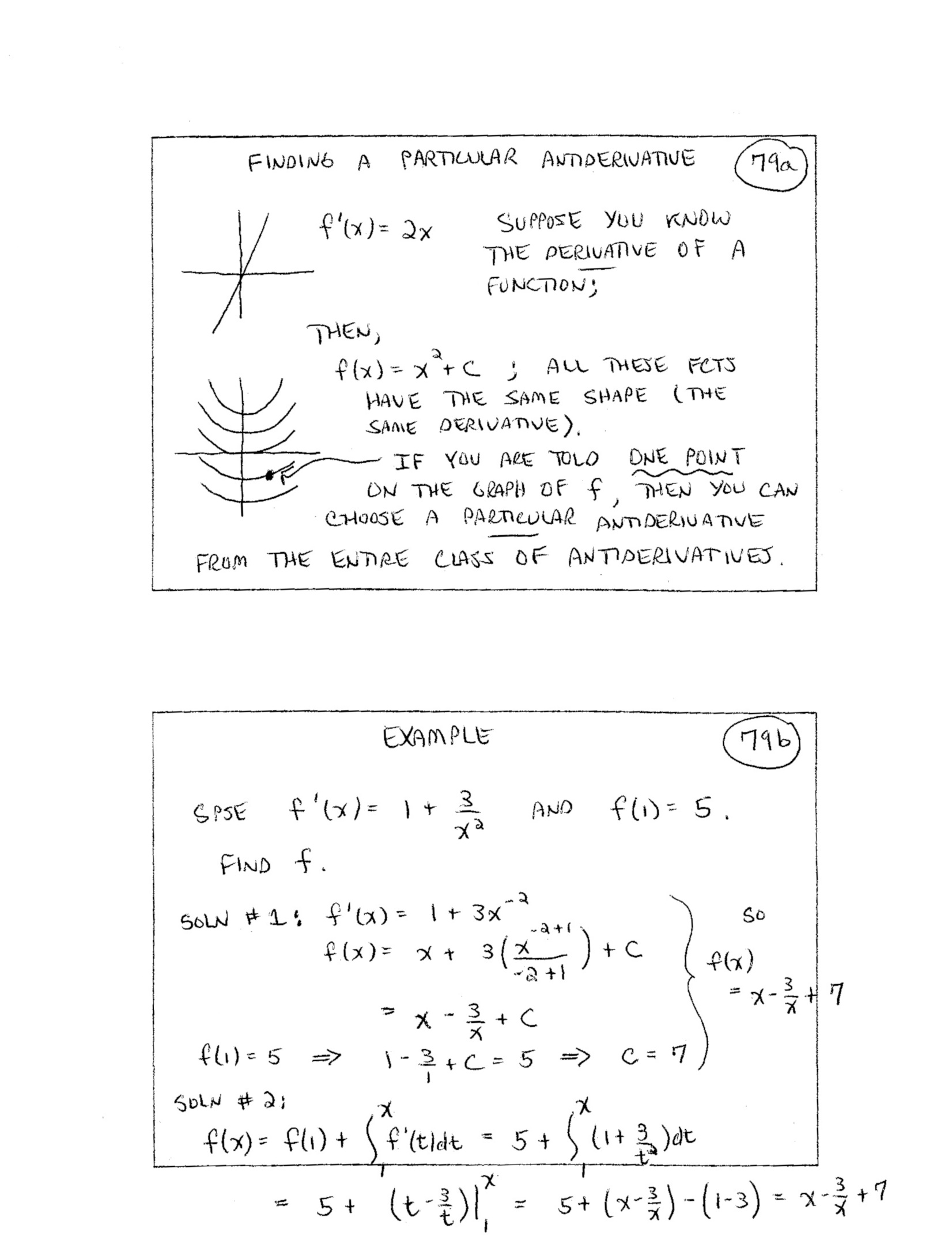80.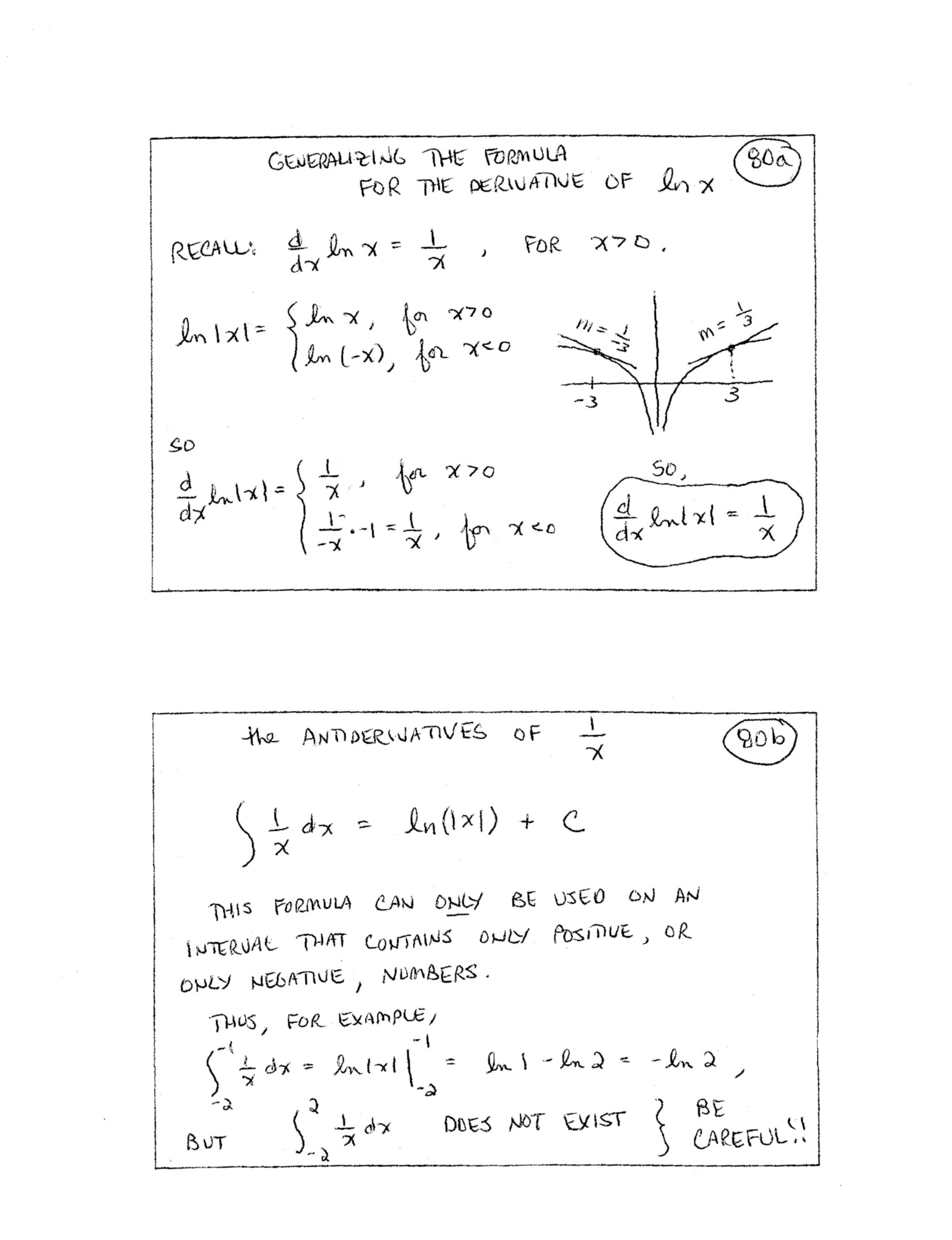81.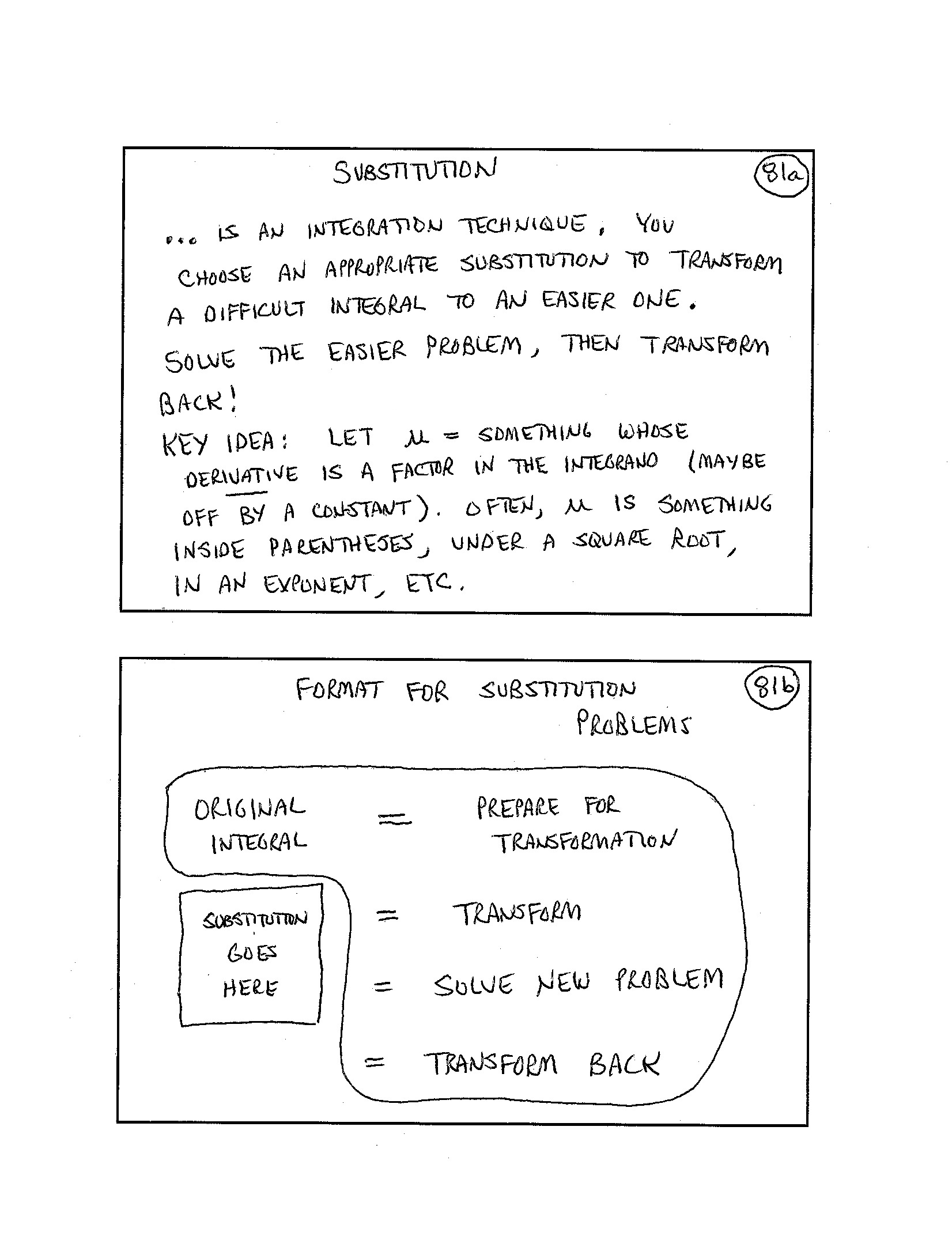82.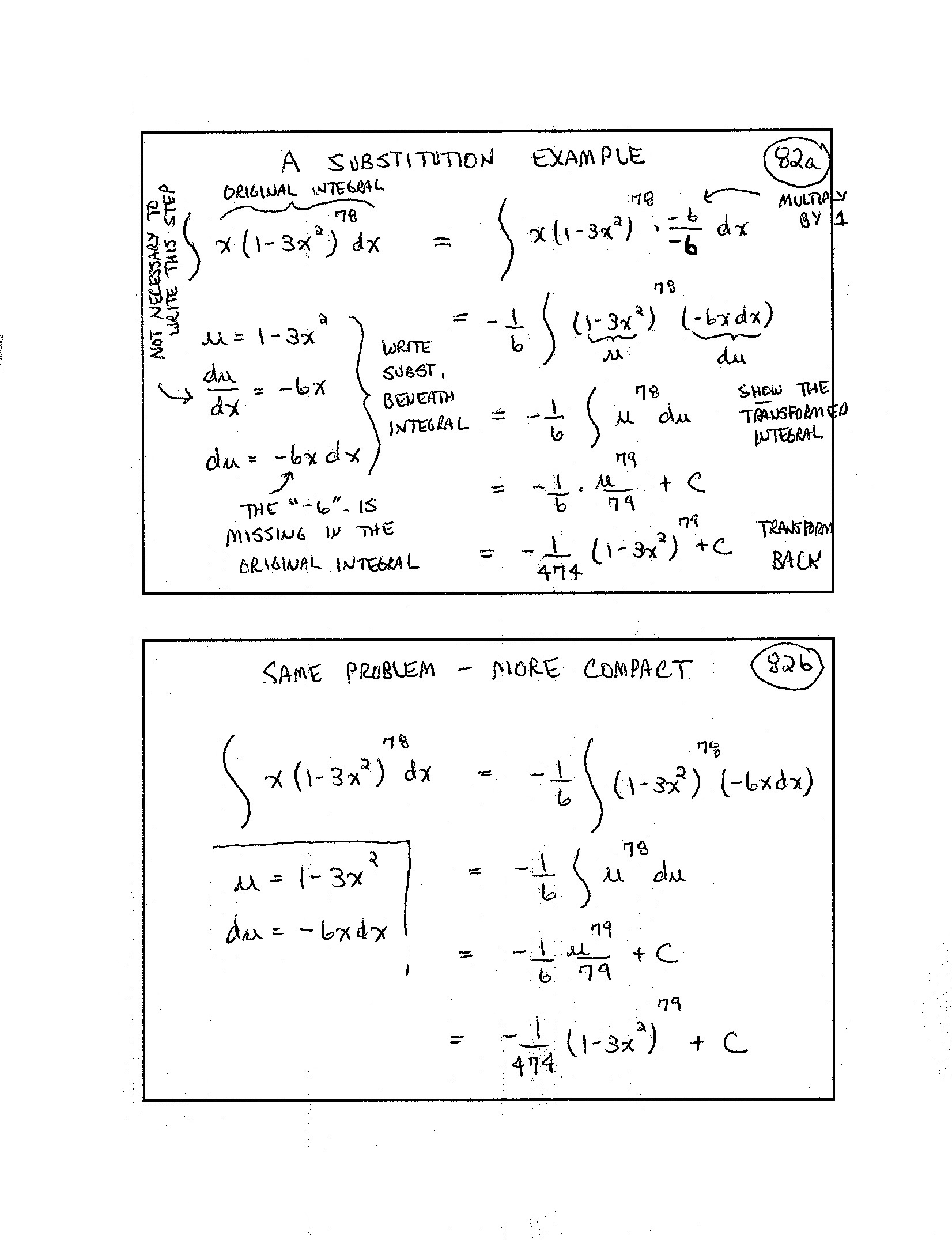83.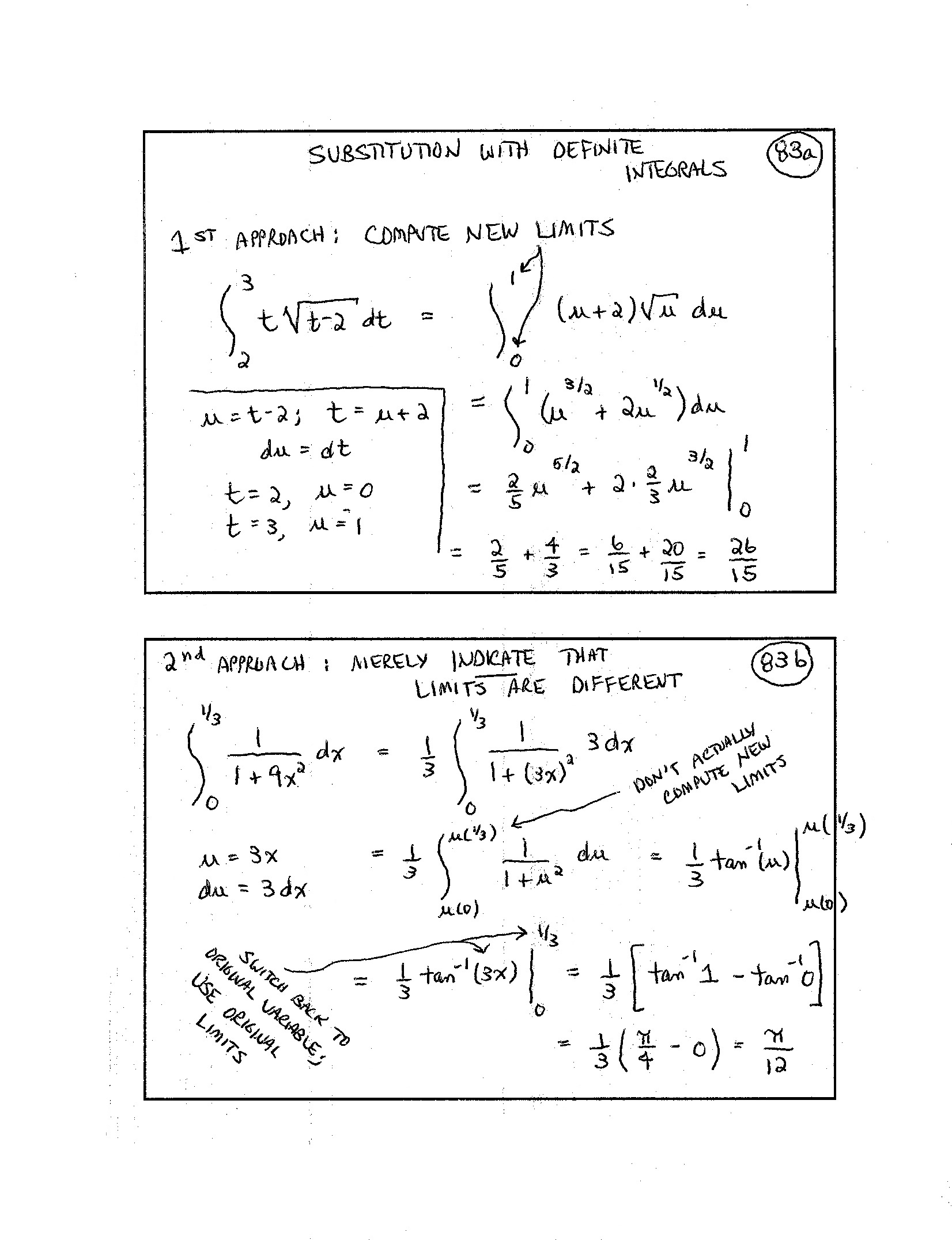84.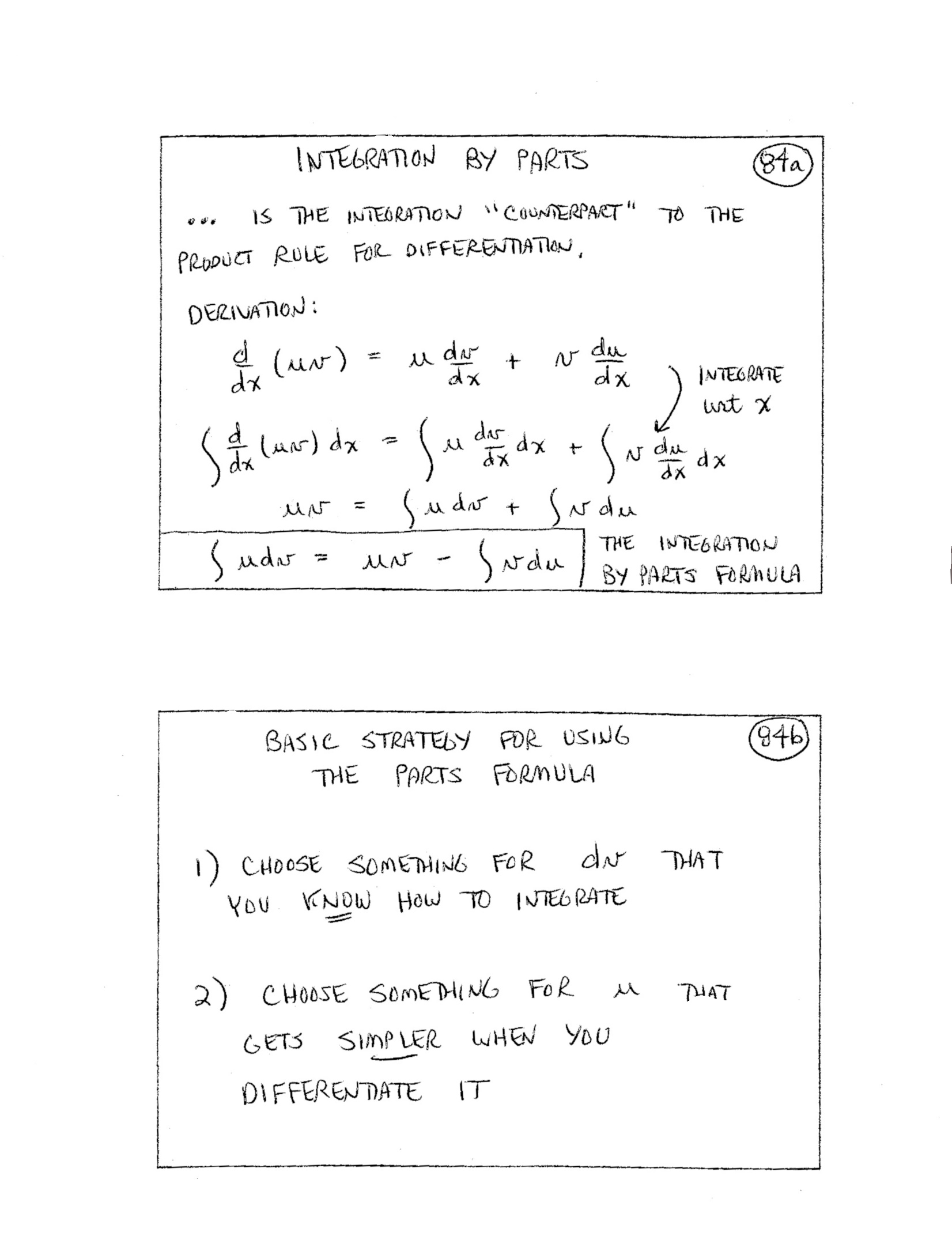85.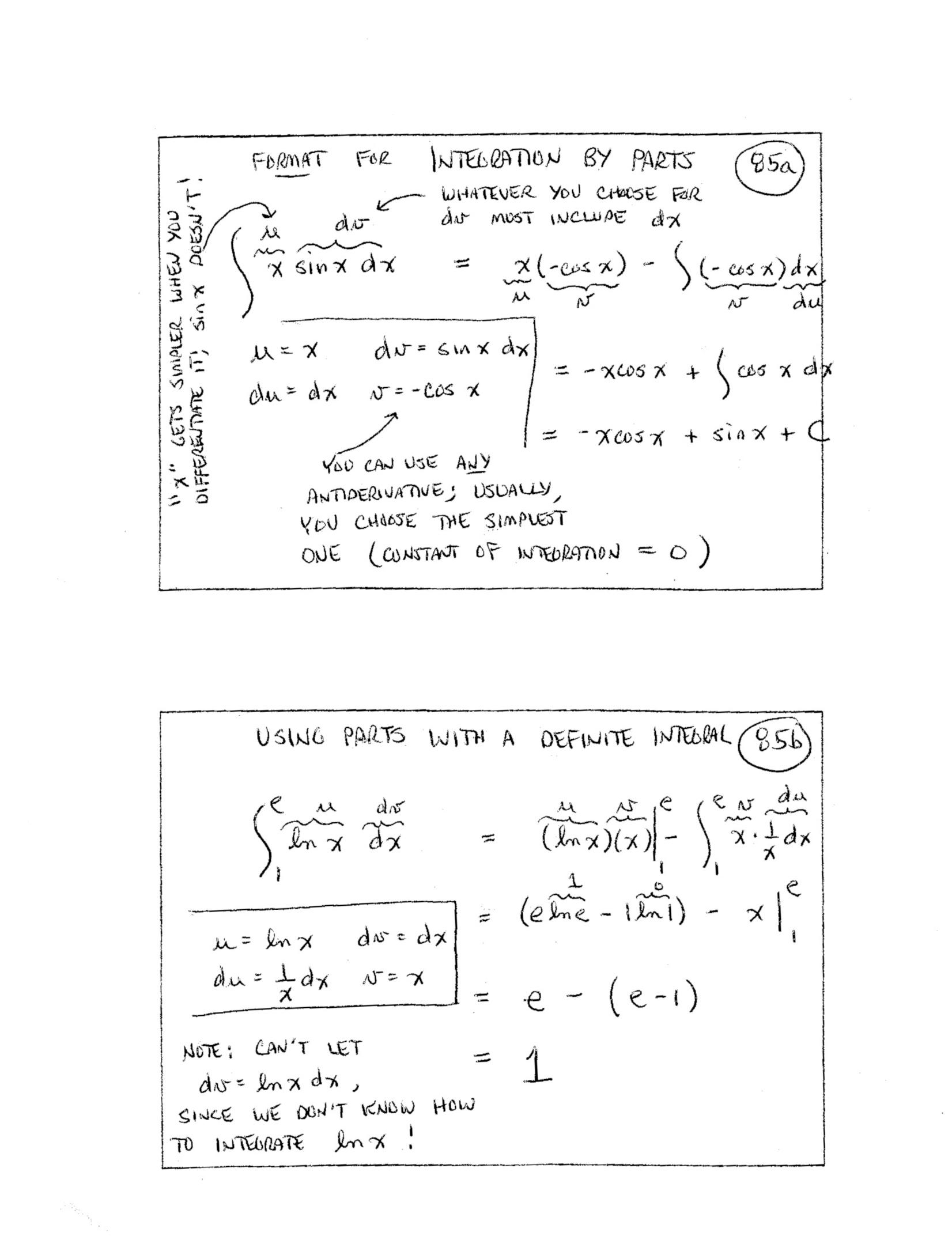86.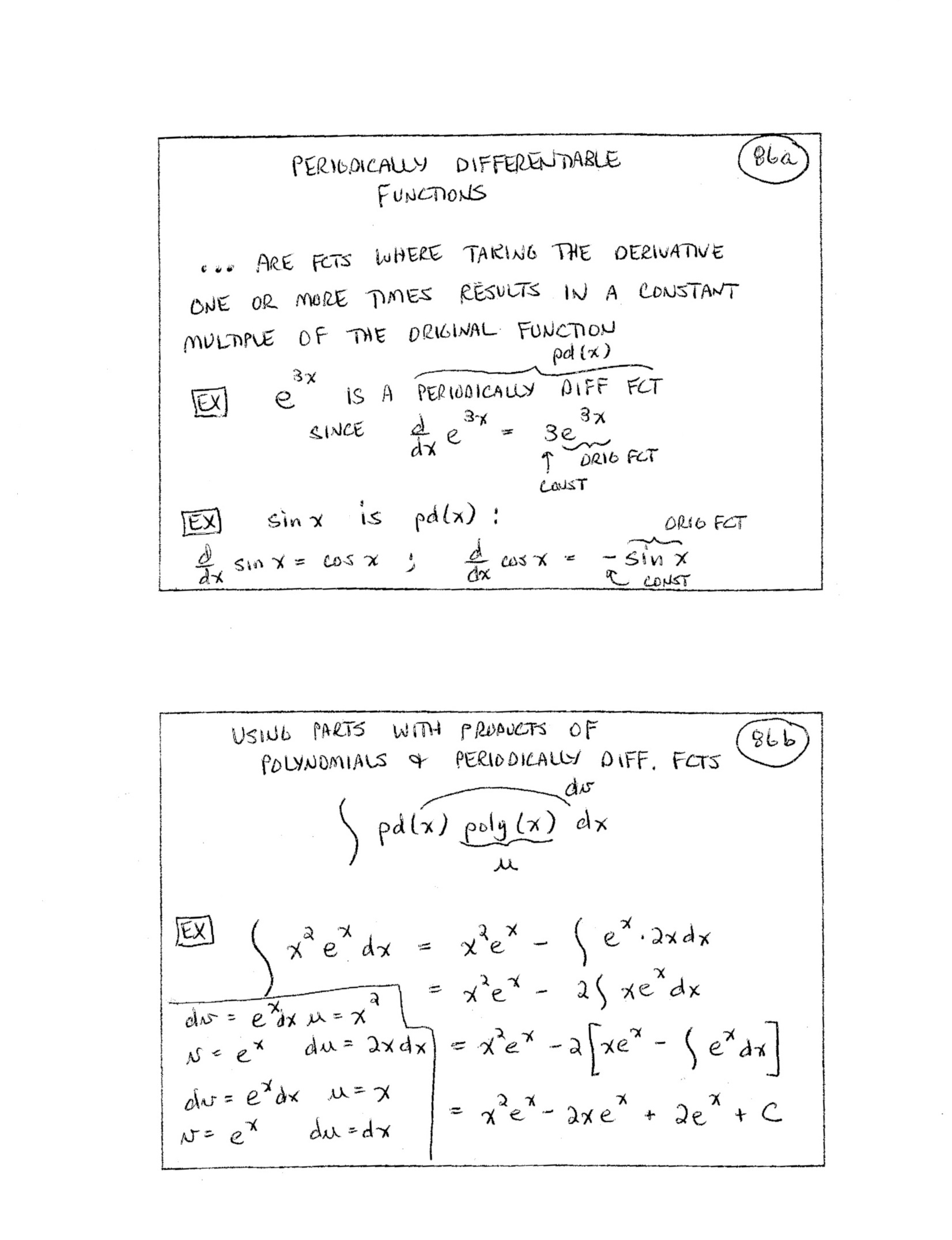(included forhigher-level course) 87.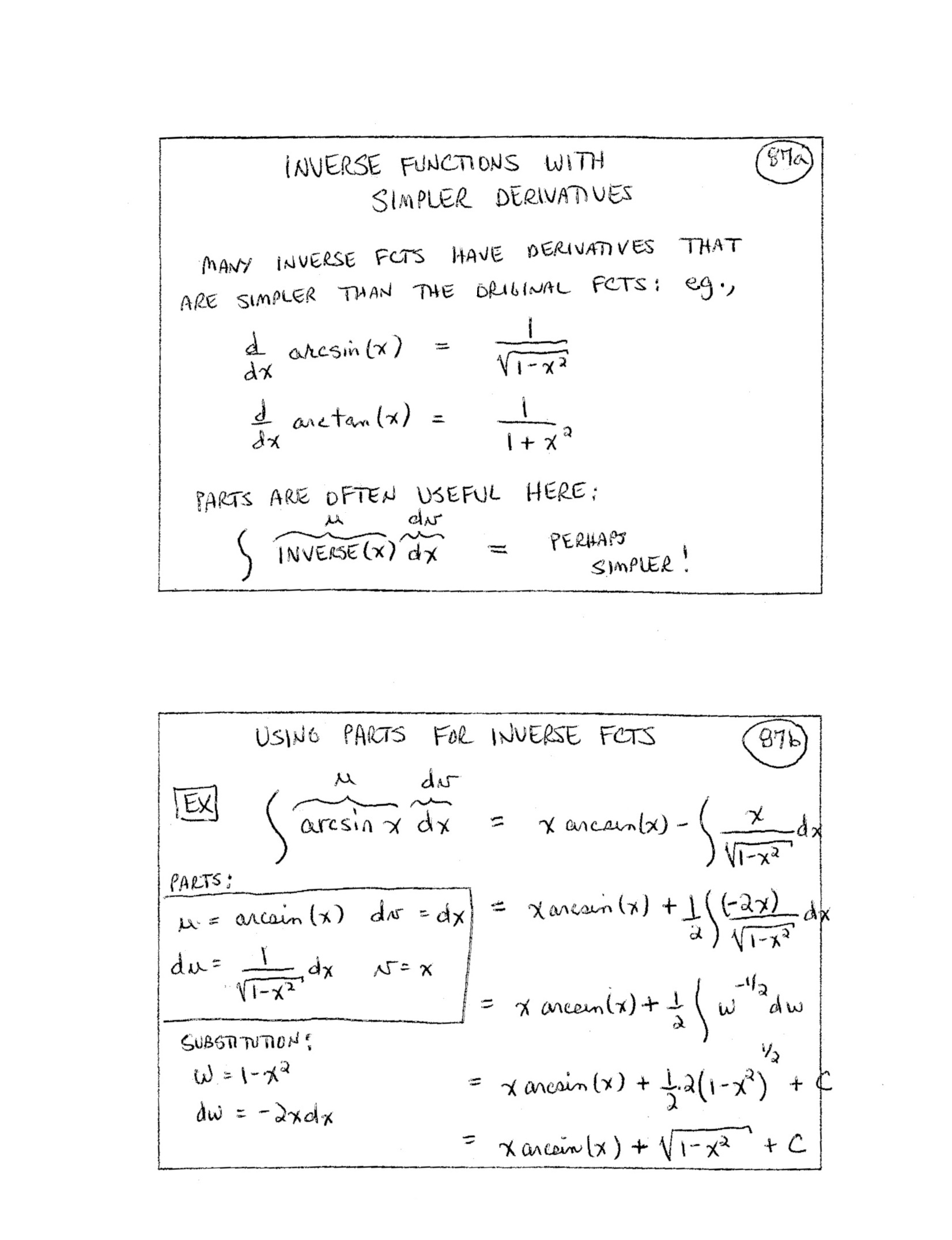(included forhigher-level course) 88.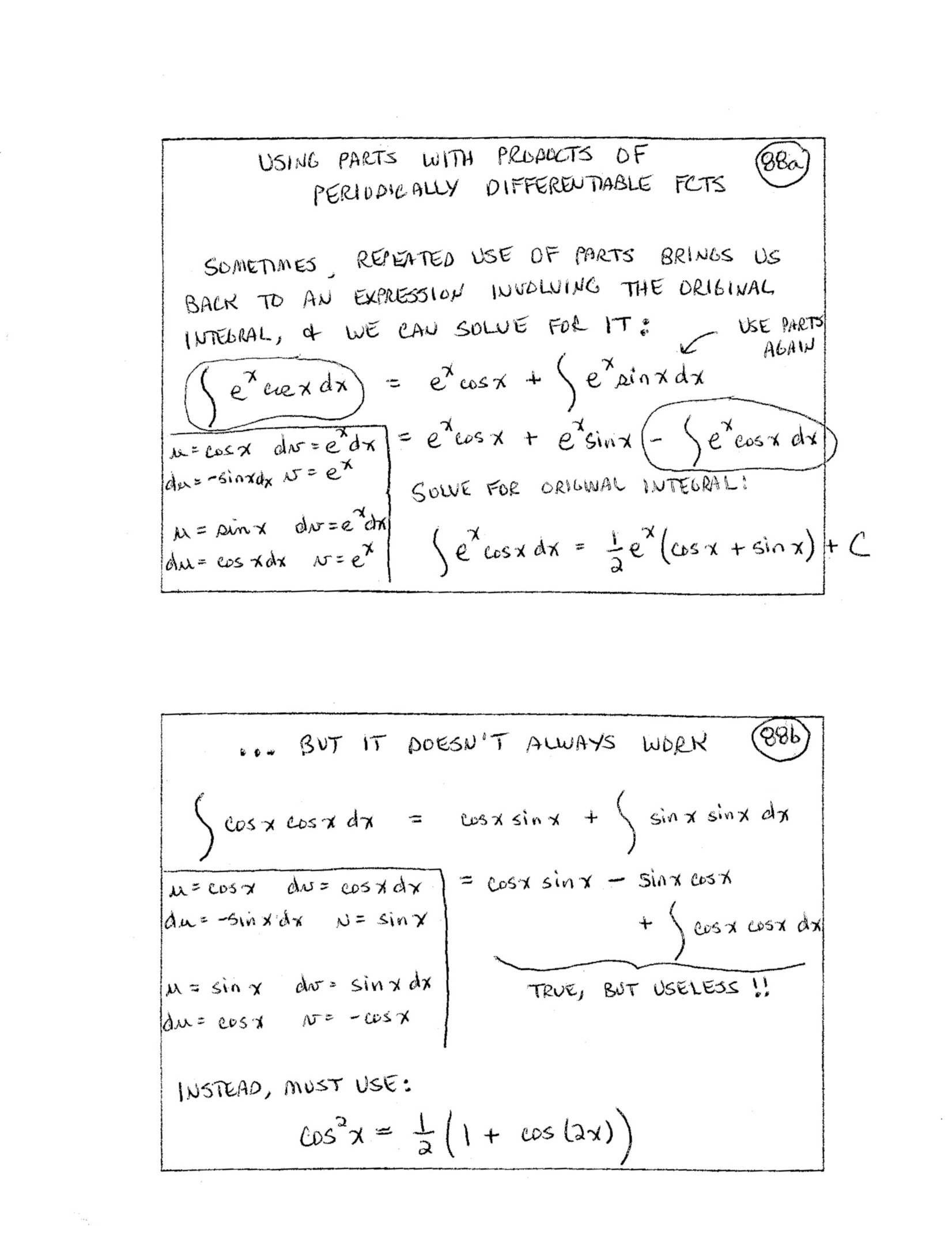(included forhigher-level course) 89.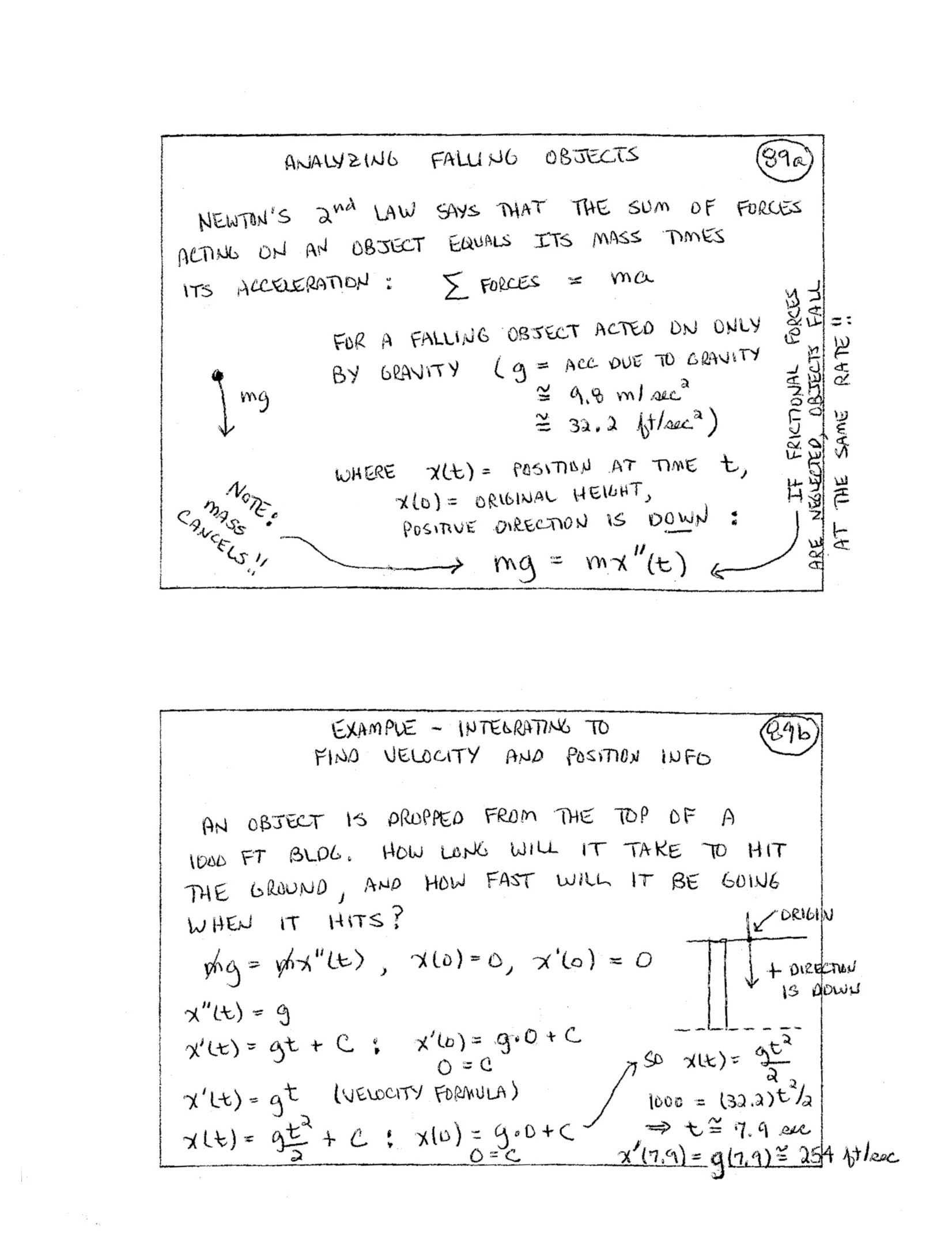# Index Card Titles/Concepts

In each entry, the first line is side (a), the second line is side (b).
Two cards (43 and 53) have additional sides (c) and (d).

1.
average rate of change;
the tangent problem
2.
position functions;
the derivative of a function
3.
intuitive derivative (notation);
example
4.
limit of a function;
3 cases when $\,\lim_{x\rightarrow a} f(x) = \ell\,$
5.
one-sided limits;
the squeeze (pinching) theorem
6.
continuity at a point;
three ways a function can fail to be continuous at $\,c\,$
7.
key idea (for continuity at a point);
example
8.
an important type of continuity problem;
relationship between differentiability and continuity
9.
limit rules;
techniques for evaluating limits (examples)
10.
indeterminate forms;
deciding if you have an ‘indeterminate form’
11.
the derivative of $\,f\,$ at $\,a\,$;
using the definition of derivative
12.
an alternative approach (compare with 11b);
another example: using the definition of derivative
13.
the linearization of $\,f\,$ at $\,a\,$;
intuition for the linearization
14.
local max/min (extreme values);
global max/min
15.
strictly increasing/decreasing functions;
getting increasing/decreasing behavior from the derivative
16.
concavity;
getting concavity info from the second derivative
17.
power rule for differentiation;
examples: using the power rule
18.
partial proof of the power rule (both sides)
19.
derivative of a constant times a function;
finding $\,\frac{d}{dx} (kx^n)\,$
20.
derivatives of sums and differences;
proof
21.
differentiating composite functions: motivation;
differentiating composite functions: the idea
22.
the chain rule (prime notation);
the chain rule (Leibnitz notation)
23.
Why is it called the ‘chain rule’?
Using the chain rule to differentiate $\,\bigl( f(x) \bigr)^n\,$
24.
pattern for generalizing all the basic differentiation formulas;
examples
25.
the product rule for differentiation;
proof of the product rule
26.
When is $\,\lim_{h\rightarrow 0} f(x+h) = f(x)\,$?
When is this true? As $\,x\rightarrow c\,$, $\,f(x)\rightarrow f(c)\,$
27.
the quotient rule for differentiation;
proof of the quotient rule
28.
the irrational number $\,\text{e}\,$;
equivalent limit statements defining $\,\text{e}\,$
29.
evaluating a limit involving $\,\text{e}\,$;
Why is $\,\lim_{n\rightarrow\infty} \left[ (1 + \frac 1n)^n \right]^{2k} = \text{e}^{2k}\,$?
30.
derivatives of logarithms;
proof that $\,\frac d{dx} (\ln x) = \frac 1x\,$
31.
more notation for derivatives;
notation: evaluating a derivative at a point
32.
differentiating $\,{\text{e}}^x\,$;
differentiating $\,a^x\,$
33.
what is $\,\lim_{x\rightarrow 0} \frac{\sin x}{x}\,$?
example
34.
derivatives of sine and cosine;
derivatives of tangent and cotangent
35.
derivatives of secant and cosecant
a useful observation—derivatives of co-functions
36.
finding the derivative of an inverse function;
two methods: finding the derivative of an inverse function
37.
What does $\,(f^{-1})'(x) = \frac{1}{f'\bigl(f^{-1}(x)\bigr)}\,$ really mean?
summary
38.
derivatives of the inverse trigonometric functions;
a typical derivation
39.
differentiating variable stuff to variable powers: the log trick;
example
40.
l'Hopital's rule;
motivation, ‘$\,\frac 00\,$’ case
41.
basic use of l'Hopital's rule;
more advanced use of l'Hopital's rule
42.
Where can a function change its sign?
negating ‘and’ and ‘or’ sentences
43ab.
(a) info given by $\,f\,$, $\,f'\,$, $\,f''\,$;
(b) a basic sign analysis of a function
43cd.
(c) implications (and equivalent sentences);
(d) the contrapositive of an implication
44.
Where can a function have a local max/min?
If $\,f\,$ has a local max/min at $\,c\,$, then ...
45.
critical points, critical numbers;
Careful!! A critical point does not have to be a max or min!!
46.
Where can a function have an inflection point?
If $\,f\,$ has an inflection point at $\,c\,$, then $\,\bigl(f''(c) = 0\ \ \text{or}\ \ f''(c) \text{ DNE}\bigr)\,$.
47.
an algorithm for thorough function analysis;
example—thorough function analysis
48.
Are critical points actually max or min? Two tests: 1st and 2nd derivative tests;
the first derivative test
49.
open/closed intervals;
bounded subsets of $\,\Bbb R\,$
50.
review of absolute/global max/min;
the Extreme Value Theorem
51.
optimization problems (finding max/min);
example
52.
a first optimization problem;
You try it!
53ab.
(a) an optimization problem;
(b) ... continued
53cd.
(c) a useful observation;
(d) using the observation
54.
an optimization problem;
... continued
55.
explicit (‘showing’) versus implicit (‘hidden’);
implicit differentiation
56.
related rate problems;
falling ladder problem
57.
Is the car speeding?
... continued
58.
the Mean Value Theorem;
the Mean Value Theorem (MVT) intuition
59.
a Mean Value Theorem example;
a Mean Value Theorem example
60.
bounded functions;
the definite integral of a function
61.
a definite integral may or may not exist;
notation for the definite integral
62.
dummy variables in definite integrals;
simple definite integral examples
63.
properties of the definite integral;
linearity of the integral
64.
definite integral example;
definite integral example
65.
How might we estimate areas beneath a curve?
general notation for the area problem
66.
summation notation;
left and right estimates using summation notation
67.
precise definition of a definite integral;
Riemann sums
68.
estimating a definite integral;
finding a definition integral using the definition (for a very simple function)
69.
the Fundamental Theorem, Part I;
proof
70.
antiderivatives;
‘undoing’ differentiation; antidifferentiation
71.
Every differentiation formula gives an antidifferentiation formula!
some antiderivatives you should know
72.
examples: using the Fundamental Theorem;
more examples
73.
A very useful formula!!
integrating a rate of change gives total change
74.
connection between area and antiderivatives;
making it more precise...
75.
making it more precise, continued (both sides)
76.
summary: the Fundamental Theorem of Calculus, Existence of an Antiderivative;
the Evaluation Theorem
77.
examples: derivatives involving integrals;
a more complicated derivative involving an integral
78.
indefinite integrals (general antiderivatives);
more antiderivative formulas
79.
finding a particular antiderivative;
example
80.
generalizing the formula for the derivative of $\,\ln x\,$;
the antiderivatives of $\,\frac 1x\,$
81.
substitution;
format for substitution problems
82.
a substitution example;
same problem, more compact
83.
substitution with definite integrals;
2nd approach: merely indicate that limits are different
84.
integration by parts;
basic strategy for using the parts formula
85.
format for integration by parts;
using parts with a definite integral
86.
periodically differentiable functions;
using parts with products of polynomials and periodically differentiable functions
87.
inverse functions with simpler derivatives;
using parts for inverse functions
88.
using parts with products of periodically differentiable functions;
... but it doesn't always work
89.
analyzing falling objects;
example: integrating to find velocity and position information
(just added on June 7!)